entropy2333 https://entropy2333.github.io/myavatar.jpg 2023-06-22T13:29:39.848Z https://entropy2333.github.io/ entropy2333 Hexo Singing Voice Conversion https://entropy2333.github.io/2023/06/22/Singing-Voice-Conversion/ 2023-06-22T13:24:03.000Z 2023-06-22T13:29:39.848Z 基于so-vits-svc的唱歌声音转换。

# Singing Voice Conversion

## 准备工作

• 克隆代码并安装依赖
git clone https://github.com/svc-develop-team/so-vits-svc.gitconda create -n aisinger python=3.8cd so-vits-svcpip install -r requirements.txt
• 下载预训练模型 checkpoint_best_legacy_500.pt，并将其放置在 pretrain 目录下
• 下载自定义模型，包括
• G_xxx.pth
• config.json
• kmeans_xxx.pt（可选）
• 使用 spleeter 来分离人声和伴奏

spleeter separate -o output/ xxx.mp3

docker run -v (pwd)/output:/output deezer/spleeter:3.8 separate -o /output xxx.mp3 它将生成两个文件：vocals.wavaccompaniment.wavvocals.wav 是用于转换的。 ## 入门 我们可以打开 webui 来转换 wav python -u webUI.py 或者直接使用 cli 进行转换 python inference_main.py \ -m "logs/44k/G_27200.pth" \ -c "configs/config.json" \ -n "/path/to/vocals.wav" \ -t 0 \ -s "sun" \ -cm "logs/44k/kmeans_10000.pt" 生成的 wav 存放在 results 目录下，然后我们使用 ffmpeg 合并两个 wav ffmpeg -i ../../results/sun_1687426444.wav -i accompaniment.wav -filter_complex amix=inputs=2:duration=longest output_1687426444.wav 使用 ffmpeg 将 wav 转换为 mp3 ffmpeg -i output_1687426444.wav -vn -ar 44100 -ac 2 -b:a 192k output_1687426444.mp3 我们还可以将两个步骤合并为一个步骤 ffmpeg -i ../../results/sun_1687426444.wav -i accompaniment.wav -filter_complex amix=inputs=2:duration=longest -vn -ar 44100 -ac 2 -b:a 192k output_1687426444.mp3 ## 问题 RuntimeError: Given groups=1, weight of size [xxx, 256, xxx], expected input[xxx, 768, xxx] to have 256 channels, but got 768 channels instead • 这是由于编码器配置和模型权重之间的不匹配。我们可以修改 config.json 中的 speech_encoder { "model"+ "speech_encoder": "vec256l9"} ## 参考资料 • https://zhuanlan.zhihu.com/p/630115251 • https://www.bilibili.com/read/cv22206231/ ]]> <p>基于so-vits-svc的唱歌声音转换。</p> Visual Prompt Tuning for Few-Shot Text Classification https://entropy2333.github.io/2022/10/17/Visual-Prompt-Tuning-for-Few-Shot-Text-Classification/ 2022-10-17T04:29:48.000Z 2022-10-17T04:29:48.159Z Abstract ## Overview links of paper pdf & code ## Background ## Method ## Experiment ]]> <p>Abstract</p> A Unified Generative Framework based on Prompt Learning for Various Information Extraction Tasks https://entropy2333.github.io/2022/10/15/A-Unified-Generative-Framework-based-on-Prompt-Learning-for-Various-Information-Extraction-Tasks/ 2022-10-15T07:32:08.000Z 2022-11-01T02:58:14.906Z 来自复旦邱组，提出了一种基于prompt的生成式框架，可以用于各种信息抽取任务。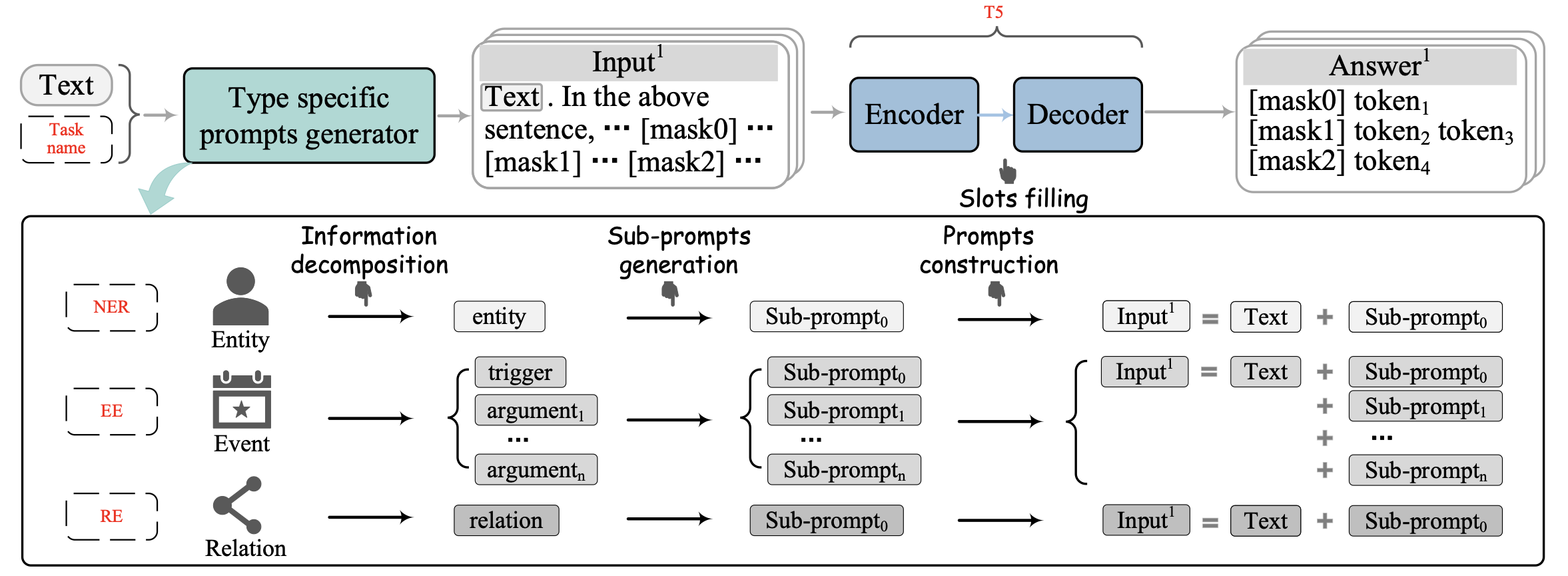## Overview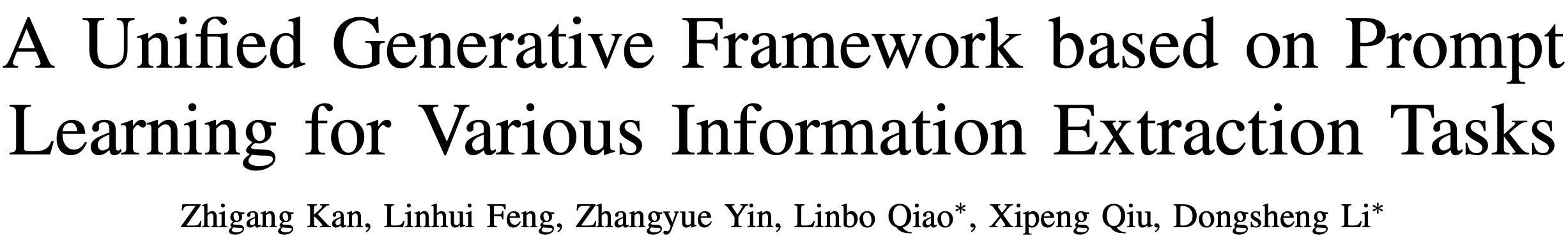## Background 在信息抽取领域，传统方法大多基于微调范式。它们针对下游任务精心设计网络结构，但是至少有两个因素会导致预训练和微调之间不可忽略的gap。 • 采用额外的网络会导致预训练和微调的结构化差异 • 预训练任务和下游任务存在显著差异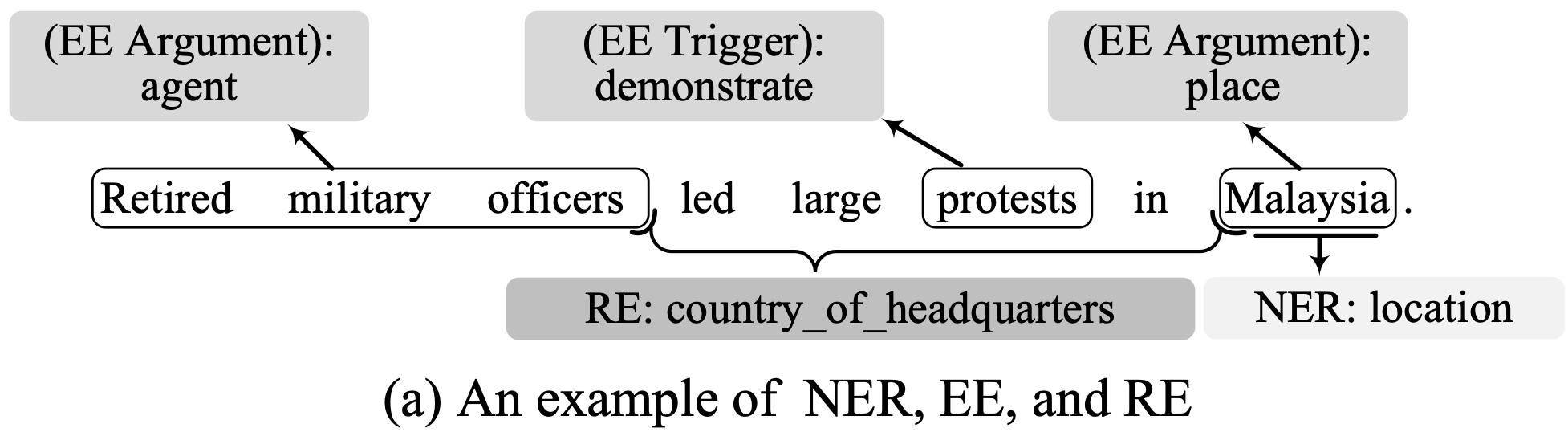prompt范式正是为了修复预训练与微调的不一致，在这种范式下，各种下游任务都转化为类似于预训练的任务。 • LightNER针对NER任务提出了一种生成式框架，其引入了prompt指导的注意力层，在低资源NER任务上取得了很好的成绩，但不能应用到其他IE任务上（例如事件抽取）。 • DEGREE为事件抽取提供了一种模版设计的方法，具体来说，它通过恢复特定类型提示中的受损文本来提取事件。然而，DEGREE通过将所有论据角色的代词集合到一个描述事件发生的短句中，构建了事件类型的特定类型提示。这导致了论据子提示和事件类型之间的密切关联。换句话说，这导致了不同事件类型的论据子提示之间存在相当大的差异。此外，DEGREE也不能应用于其他IE任务（例如关系抽取）。 • PTR是一个通用的基于提示的文本分类框架。它通过结合子提示和可学习的虚拟标记来生成提示，并通过仅有编码器的PLM将[MASK]标记映射到候选答案来判断关系的类型。然而，PTR只能用预先设计的子符号和逻辑规则对特定的文本内容进行分类，但不能从文本中提取具有可变长度的信息，如实体和事件。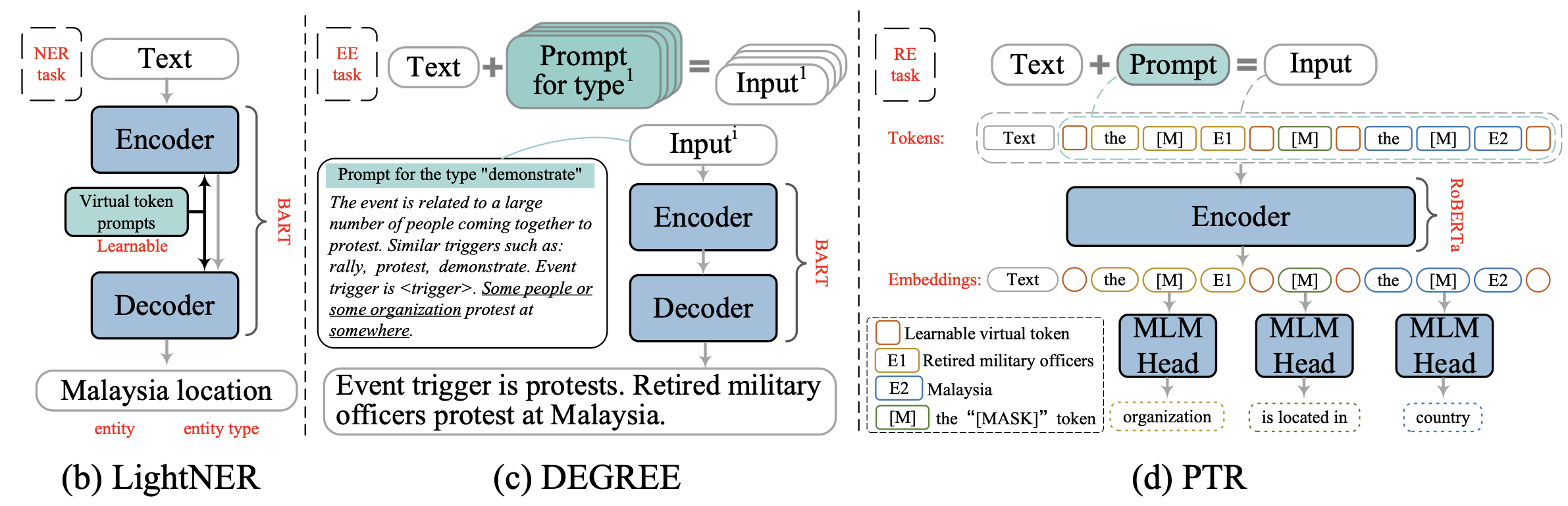为了缓解这些问题，本文为所有的IE任务引入了一个新的统一的基于提示的生成框架（CPGF）。CPGF将各种IE任务转换为完形填空任务，并用T5来预测答案。本文的贡献如下： • 本文提出了一个基于提示学习范式的新型统一生成框架，用于各种信息抽取任务。 • 本文介绍了一种为复杂任务（如EE）构建独立子提示的方法。此外，为了提高框架在数据稀缺的情况下提取事件的通用能力，为事件提取设计了一种由多个模块化子提示组成的可组合提示。 • 本文提出了一种基于提示的方法，通过判断语义矛盾来实现关系抽取，并为其设计了一个相应的模板。 • 一系列关于EE、NER和RE的实验结果表明，该框架在数据丰富和数据稀缺的情况下都很有效。 ## Method 本文提出的框架包含四个部分： • 信息分解表示将复杂的信息分割成多个片段。 • 子提示生成产生信息片段的子提示。 • 提示构建产生信息类型的特定类型或可组合提示（EE独有）。 • 在填槽阶段，采用T5来预测答案。 ### Notation • 原始文本$$S$$ • 目标信息$$\mathcal{Y}=\{\mathcal{Y}^1,\cdots,\mathcal{Y}^t\}$$ • $$\mathcal{Y}^j$$表示类型$$j$$的待提取信息，$$t$$为类型总数 • 对于EE，$$\mathcal{Y}^j$$可能包含多个元素，如事件类型和论元角色 • 提示$$Pr=\{Pr^1,\cdots,Pr^t\}$$ ### Sub-Prompts Generation 依赖于类型的子提示适用于数据丰富的场景，而模块化的子提示是为数据稀缺的场景设计的。下图显示了这两种子提示之间的区别。该图的左边部分显示了依赖类型的子提示和信息类别之间的关系。在这种情况下，子提示是通过用掩码词替换每个信息类型的定义或片段描述中的关键词而独立产生的。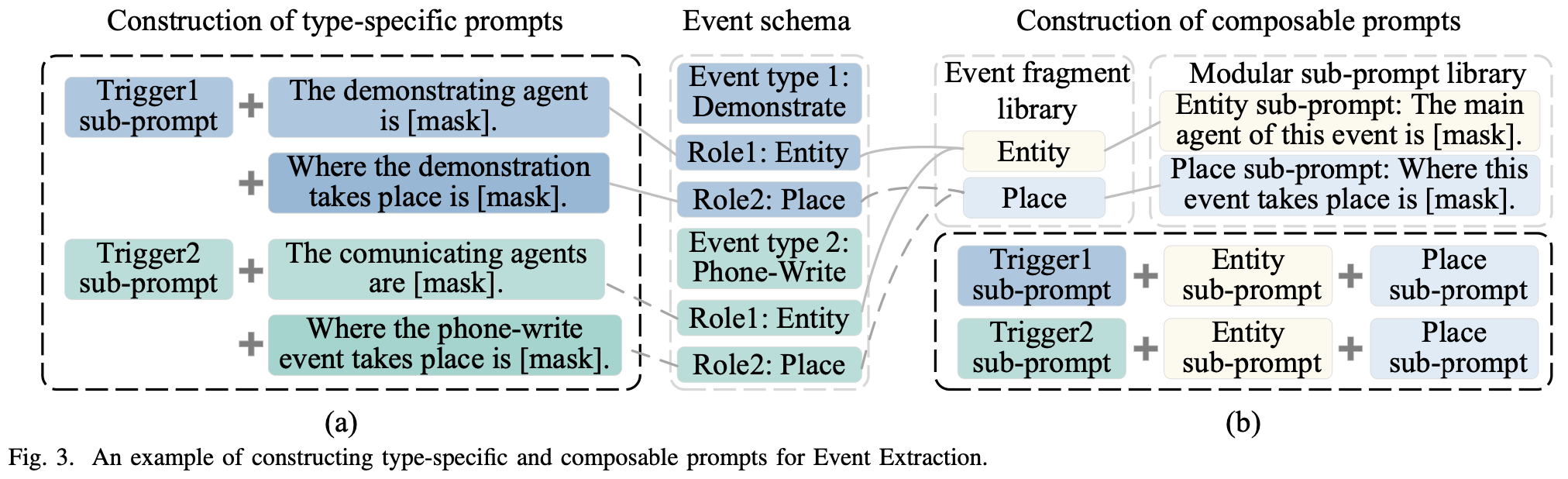在使用模块化子提示的情况下，我们在产生子提示之前合并类似的信息片段。CPGF将具有相同语义但分布在不同类型信息中的元素视为同一信息片段。通过分析训练数据集中的信息元素的语义，手工建立了一个涵盖每种信息类型的所有组成的信息片段库。数据集中的每一类信息都由库中的一个或多个信息片段组成。 我们通过为信息片段库中的每个元素生成一个与类型无关的子提示，组成一个模块化的子提示库$$P=\{p_1,p_2,\cdots,p_{\lvert F\rvert}\}$$，其中$$\lvert F\rvert$$表示信息片段的总数。详细地说，它在一个描述片段和信息类型之间关系的通用句子中替换了关键词，以获得一个模块化的子提示。我们的框架通过从模块化子提示库中搜索其片段来获得目标信息的子提示。通过这种方式，CPGF可以有效地提取具有从未见过的类型的信息元素，如果这些元素出现在片段库中。 ### Prompts Construction $$j$$种信息类型的提示是通过连接原始文本和子提示来构建的： \begin{align}\mathcal{S}_{\text{prompt}}^j &= f_{\text{prompt}}(S) \\ &= S \mathop{\Vert} Pr^j\end{align} 其中$$\mathop\Vert$$表示拼接两段文本，$$Pr^j$$表示组合目标信息中所有相关元素的子提示： $Pr^j = \mathop{\Vert}_{i=1}^n p_i^j$ 对于NER和RE，$$n$$始终为1。对于EE，$$S^j_{\text{promt}}$$被称为特定类型的prompt，如果用来组成的提示语是依赖于类型的，因为这个提示语的每个部分都与信息类型有关。由模块化子提示组成的提示被命名为 "可组合提示"。 ### Answer Generation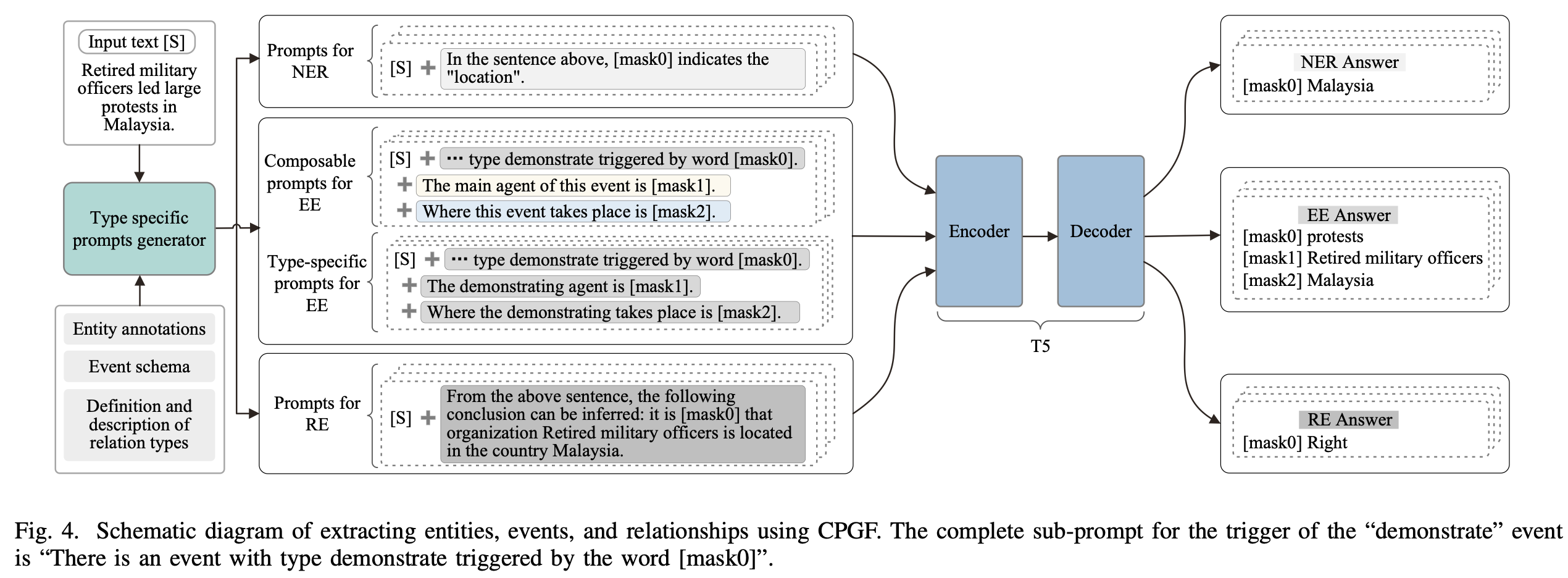在获得样本的一系列提示后，将其送入PLM，通过MLM预测每个提示中MASK词的预测值。 $z_i^j = \text{MLM}(p_i^j)$ 在这个过程中，使用特殊字符 $$\vert$$ 分隔子提示的多个答案。 ## Experiment ]]> <p>来自复旦邱组，提出了一种基于prompt的生成式框架，可以用于各种信息抽取任务。</p> <p><img src="/2022/10/15/A-Unified-Generative-Framework-based-on-Prompt-Learning-for-Various-Information-Extraction-Tasks/image-20221015230422371.png" alt="image-20221015230422371" style="zoom:33%;"></p> A Unified Generative Framework for Various NER Subtasks https://entropy2333.github.io/2022/10/15/A-Unified-Generative-Framework-for-Various-NER-Subtasks/ 2022-10-15T07:31:54.000Z 2022-10-15T08:37:13.594Z ACL 2021，来自复旦邱组，用生成式统一NER。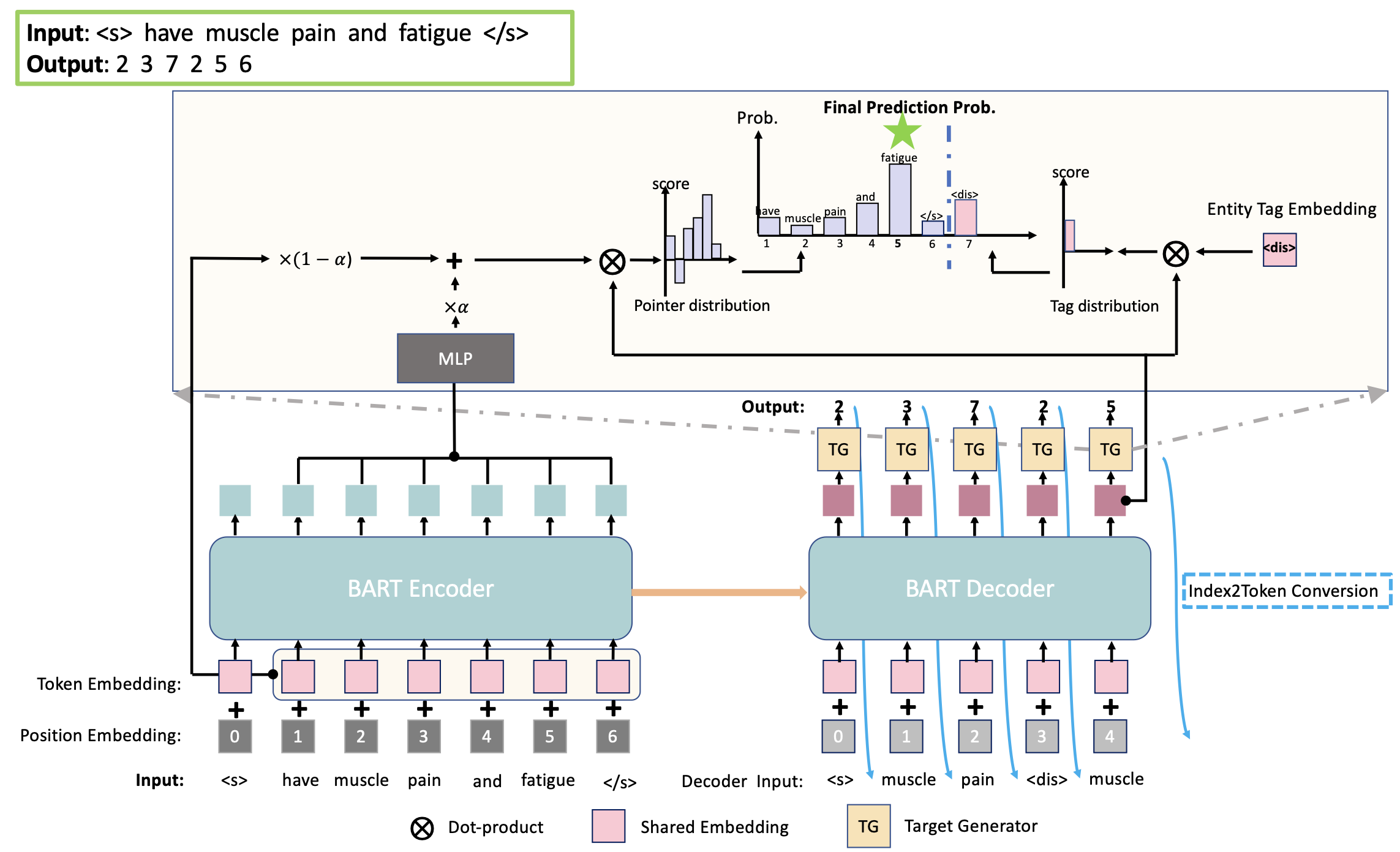## Overview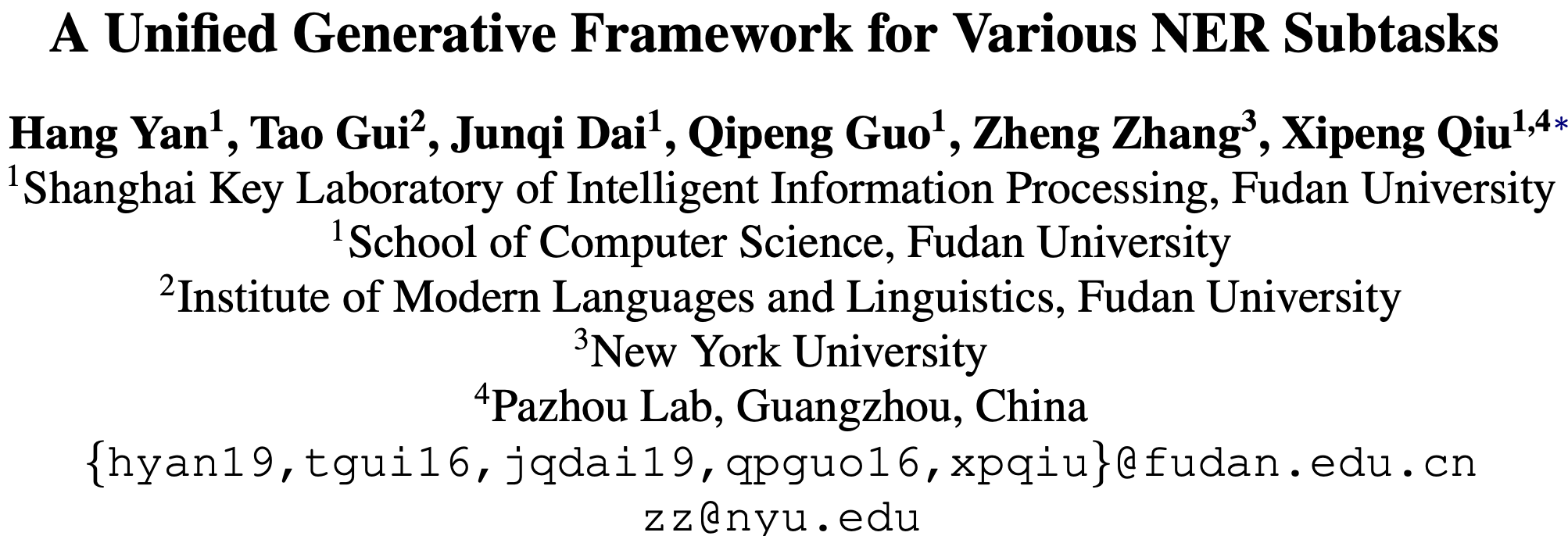## Background NER旨在识别句子中的实体片段，这些实体可能是嵌套的或者是不连续的，因此NER任务可以分为flat NER、nested NER和discontinuous NER。一般而言，这些子任务可以通过序列标注或者span-level的分类解决。 本文的贡献如下： • 提出了一种生成式的统一框架，将NER问题形式化为实体span序列生成问题。 • 框架融合了预训练模型BART，并提出三种实体表示的序列化方法。 • 提出的框架避免了对标注schema和枚举span的复杂设计，在8个英文数据集上取得了SOTA，包括2个flat NER、3个nested NER和3个discontinuous NER。 ## Method ### Notation • 输入句子$$X=[x_1,x_2,\cdots,x_n]$$ • 目标序列$$Y=[s_{11},e_{11},\cdots,s_{1j},e_{1j},t_1,\cdots,s_{i1},e_{i1},s_{ik},e_{ik},t_i]$$ • 其中$$s$$$$e$$表示一个span的起止下标，$$t_i$$表示标记下标。 • 实体类别$$G=[g_1,\cdots,g_l]$$ • $$l$$表示类别总数 • $$t_i\in(n,n+l]$$，移位是为了不与指针下标混淆。 ### Seq2Seq for Unified Decoding 模型主要包括Encoder和Decoder两部分。 对于Encoder而言，将文本编码为隐层表示： $\mathbf{H}^{e} = \text{Encoder}(X)$ 对于Decoder而言，负责计算每一步的下标分布$$P_t = P(y_t|X,Y_{\lt t})$$。因为$$Y_{\lt t}$$包含指针下标和标记下标，所以先按如下规则转换为token： $\hat{y}_t = \begin{cases} X_{yt}&\text{if}\ y_t\le n \\ G_{yt-n}&\text{if}\ y_t > n\end{cases}$ 转换之后，就可以计算最终表示： $h_t^d = \text{Decoder}(\mathbf{H}^e;Y_{\lt t})$ 可以通过下式获得下标的概率分布$$P_t$$\begin{align} \mathbf{E}^e & = \mathrm{TokenEmbed}(X)\\ \mathbf{\hat{H}}^e & = \mathrm{MLP}(\mathbf{H}^e) \\ \mathbf{\bar{H}}^e & = \alpha*\mathbf{\hat{H}}^e + (1-\alpha)*\mathbf{E}^e \\ \mathbf{G}^d & = \mathrm{TokenEmbed}(G) \\ P_t & = \mathrm{Softmax}([\mathbf{\bar{H}^e}\otimes \mathbf{h}_t^d;\mathbf{G}^d\otimes \mathbf{h}_t^d] )\end{align} 其中$$\text{TokenEmbed}$$是Encoder和Decoder共享的embedding，$$\alpha$$为超参数。训练时采用teacher forcing，推理时自回归生成目标序列。解码时采用如下算法，将下标序列转换为实体span。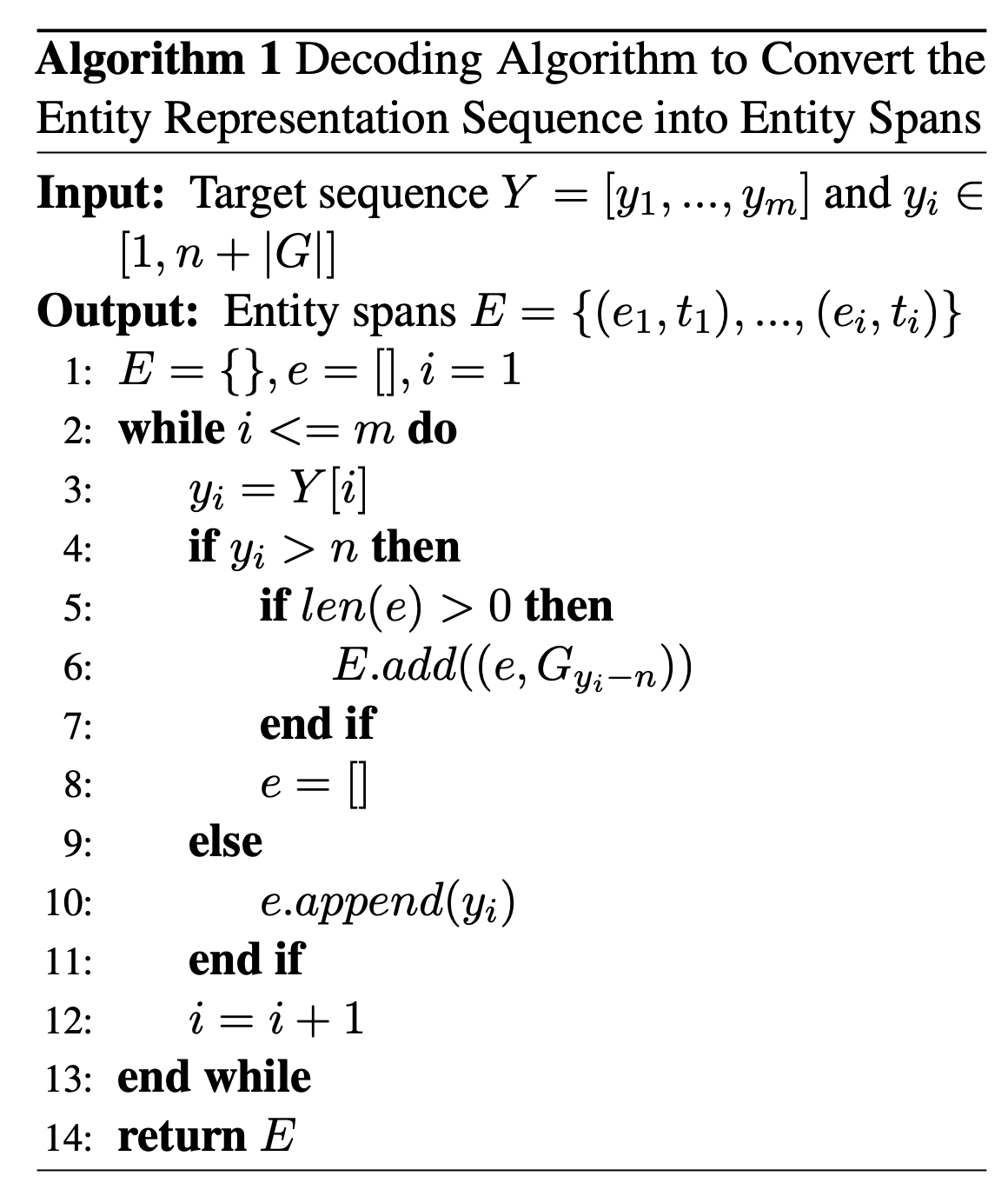BART采用的BPE算法可能会把一个token切分为多个BPE字符，为了更充分利用BART，本文提出了三种基于指针的实体表示，用于定位原始语句中的实体，如下图所示：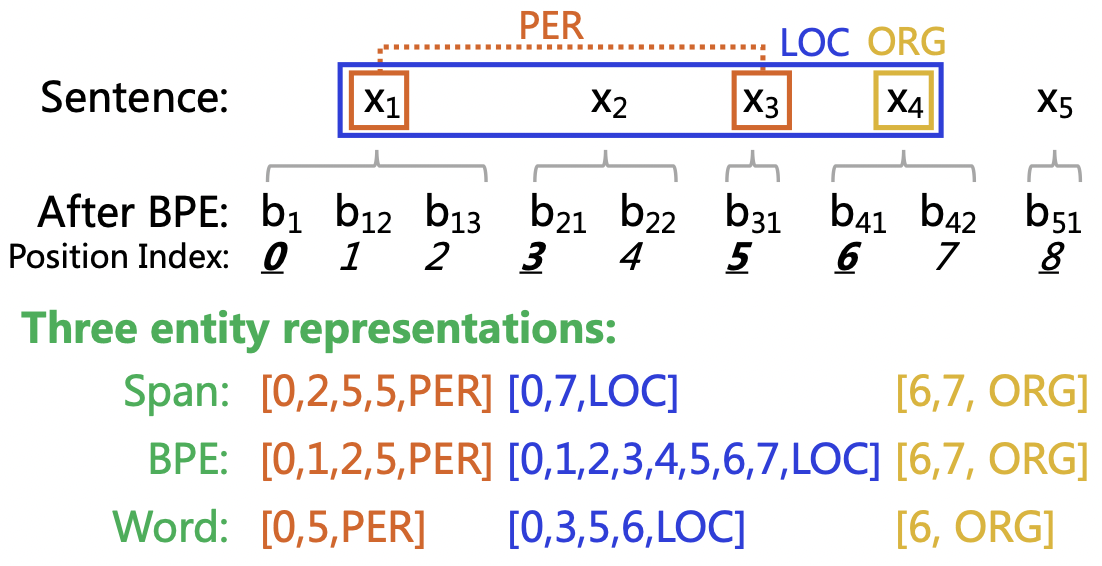• Span：使用实体的起始和截止BPE下标。 • BPE：使用实体的所有BPE下标。 • Word：只使用每个实体的第一个BPE下标。 如果句子中没有实体，那么输出就是空序列（只包含起始字符<s>和终止字符</s>）。 ## Experiment 选用了八个数据集： • Flat NER: CoNLL-2003, OntoNotes • Nested NER: ACE 2004, ACE 2005, Genia • Discontinuous NER: CADEC, ShARe13, ShARe14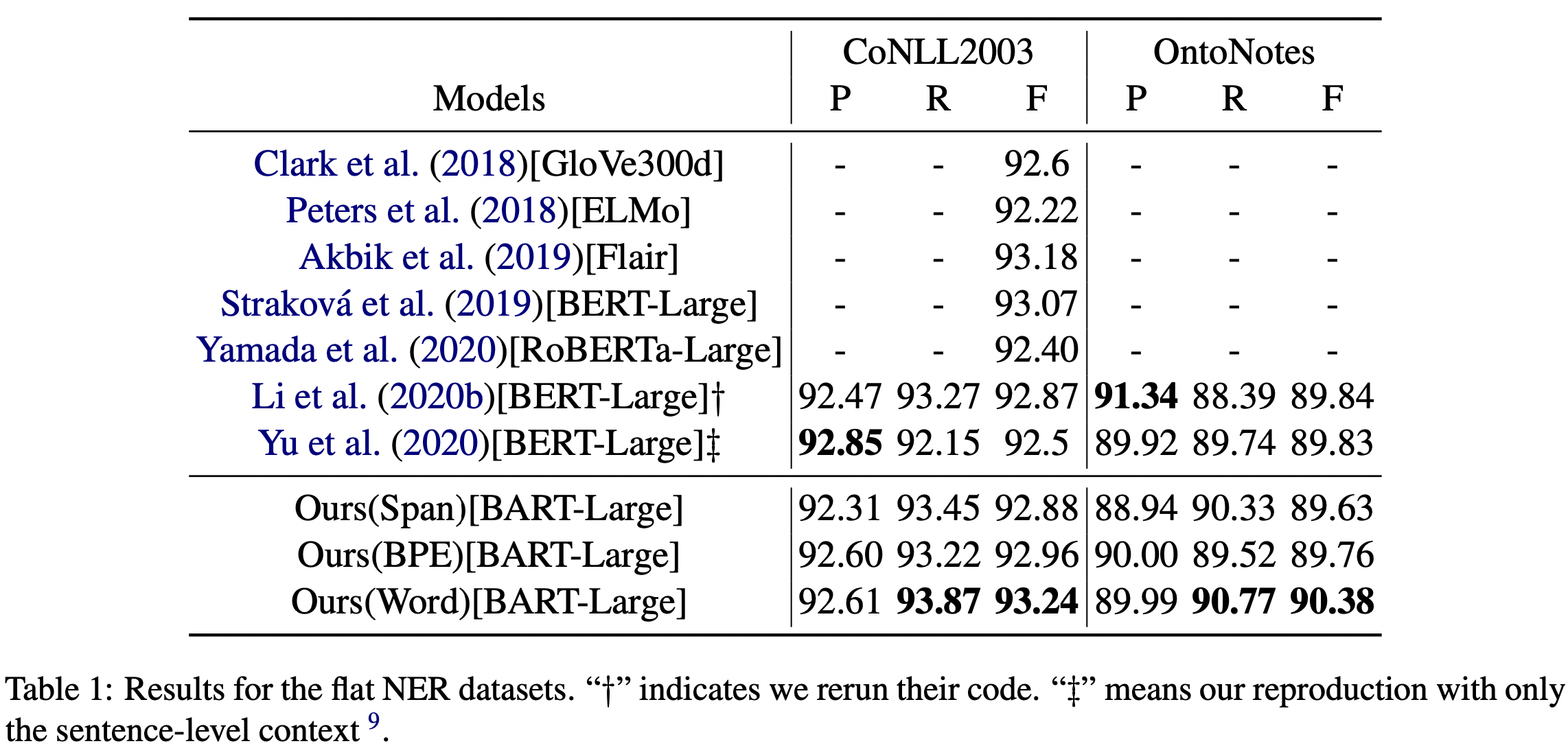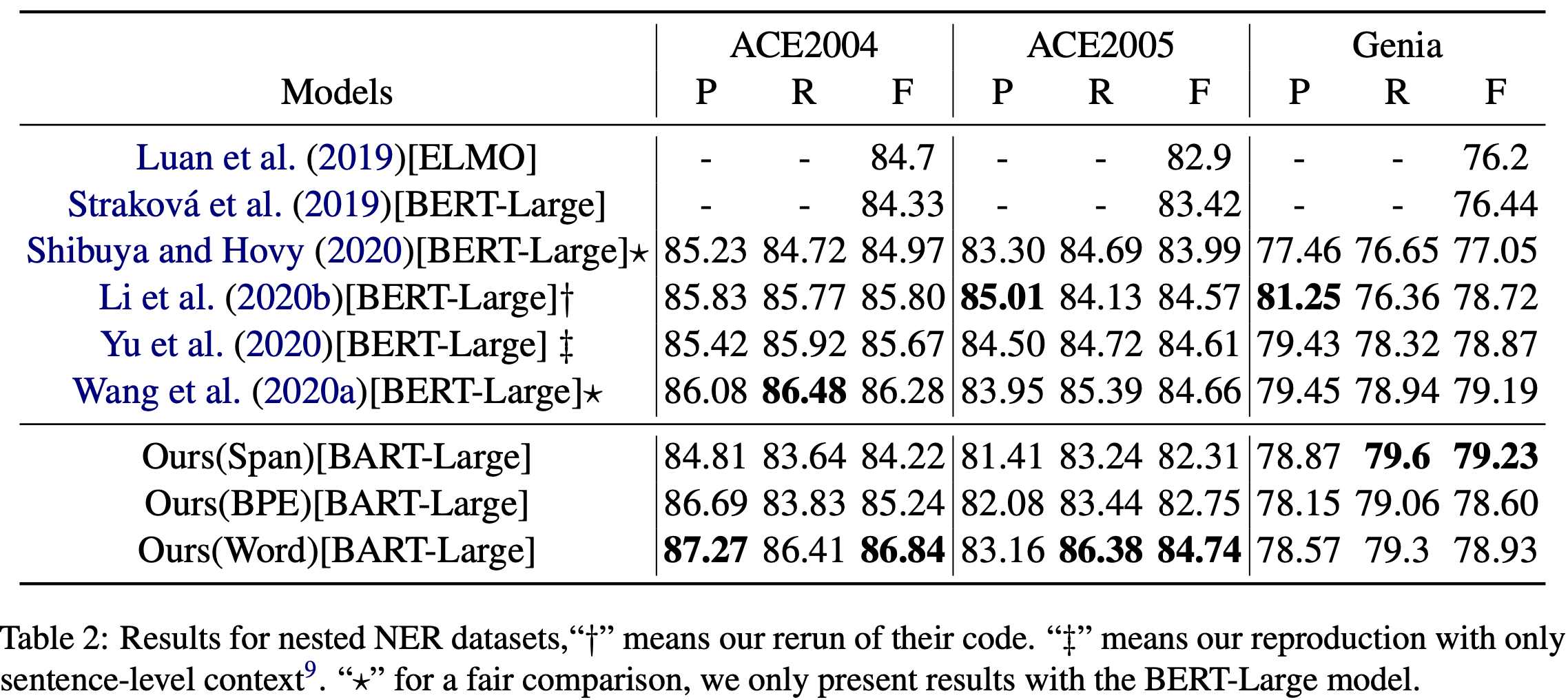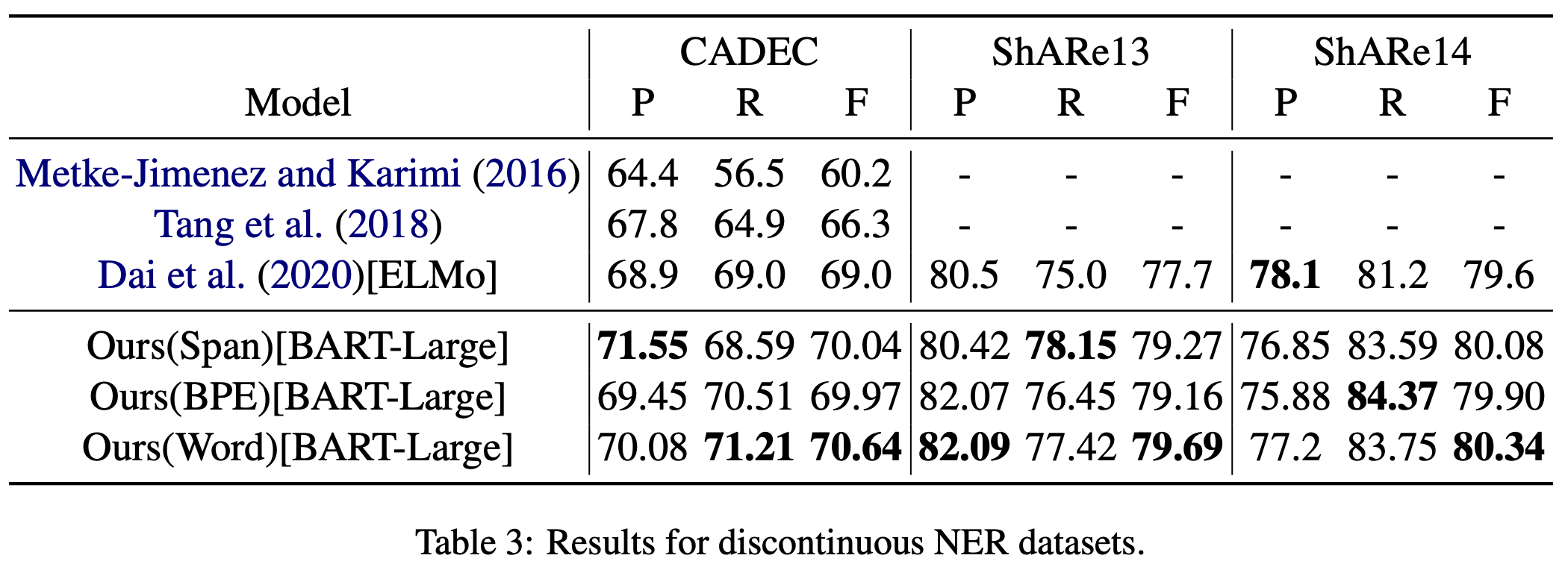对实体表示的消融实验：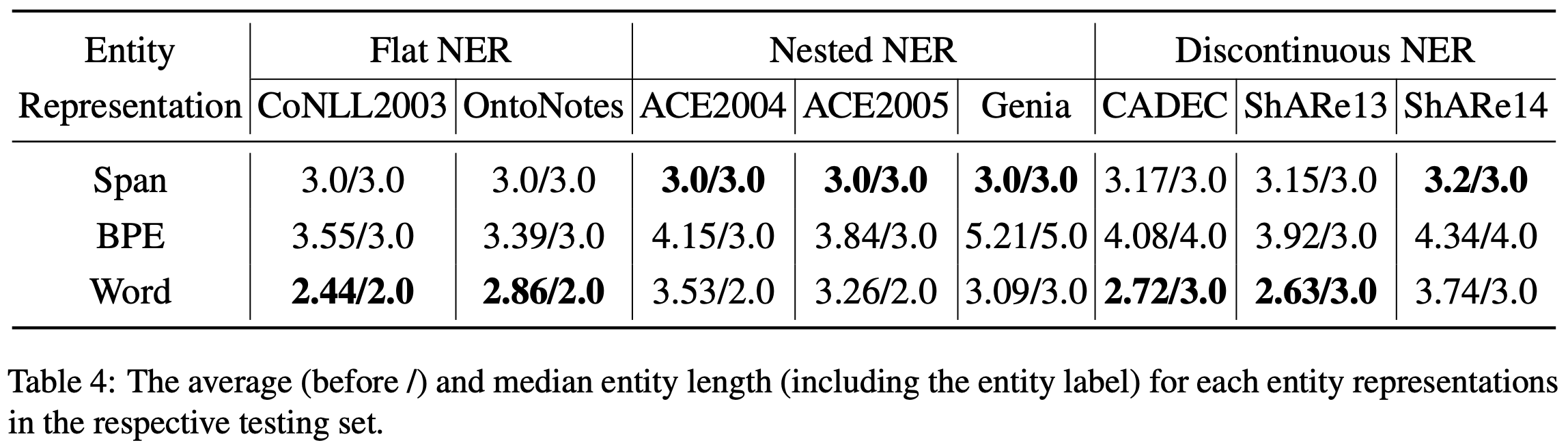Word实体表示比其他两种更好。 ]]> <p>ACL 2021，来自复旦邱组，用生成式统一NER。</p> <p><img src="/2022/10/15/A-Unified-Generative-Framework-for-Various-NER-Subtasks/image-20221015155838142.png" alt="image-20221015155838142" style="zoom: 33%;"></p> 镜像网站配置 https://entropy2333.github.io/2022/06/02/%E9%95%9C%E5%83%8F%E7%BD%91%E7%AB%99%E9%85%8D%E7%BD%AE/ 2022-06-02T14:33:37.000Z 2022-09-07T09:08:34.893Z 配置环境时常常要修改国内镜像，记录常用的镜像网站。 ## 常见镜像站 ## 常用的配置 ## Homebrew 以中科大为例 # 替换brew.gitcd "(brew --repo)"git remote set-url origin https://mirrors.ustc.edu.cn/brew.git# 替换homebrew-core.gitcd "$(brew --repo)/Library/Taps/homebrew/homebrew-core"git remote set-url origin https://mirrors.ustc.edu.cn/homebrew-core.git# 应用生效brew update# 替换homebrew-bottlesecho 'export HOMEBREW_BOTTLE_DOMAIN=https://mirrors.aliyun.com/homebrew/homebrew-bottles' >> ~/.zshrcsource ~/.zshrc ]]> <p>配置环境时常常要修改国内镜像，记录常用的镜像网站。</p> HPT: Hierarchy-aware Prompt Tuning for Hierarchical Text Classification https://entropy2333.github.io/2022/05/08/HPT-Hierarchy-aware-Prompt-Tuning-for-Hierarchical-Text-Classification/ 2022-05-08T01:55:01.000Z 2022-05-13T16:47:43.301Z 用 Prompt 方法做层次文本分类，并融合了层次信息。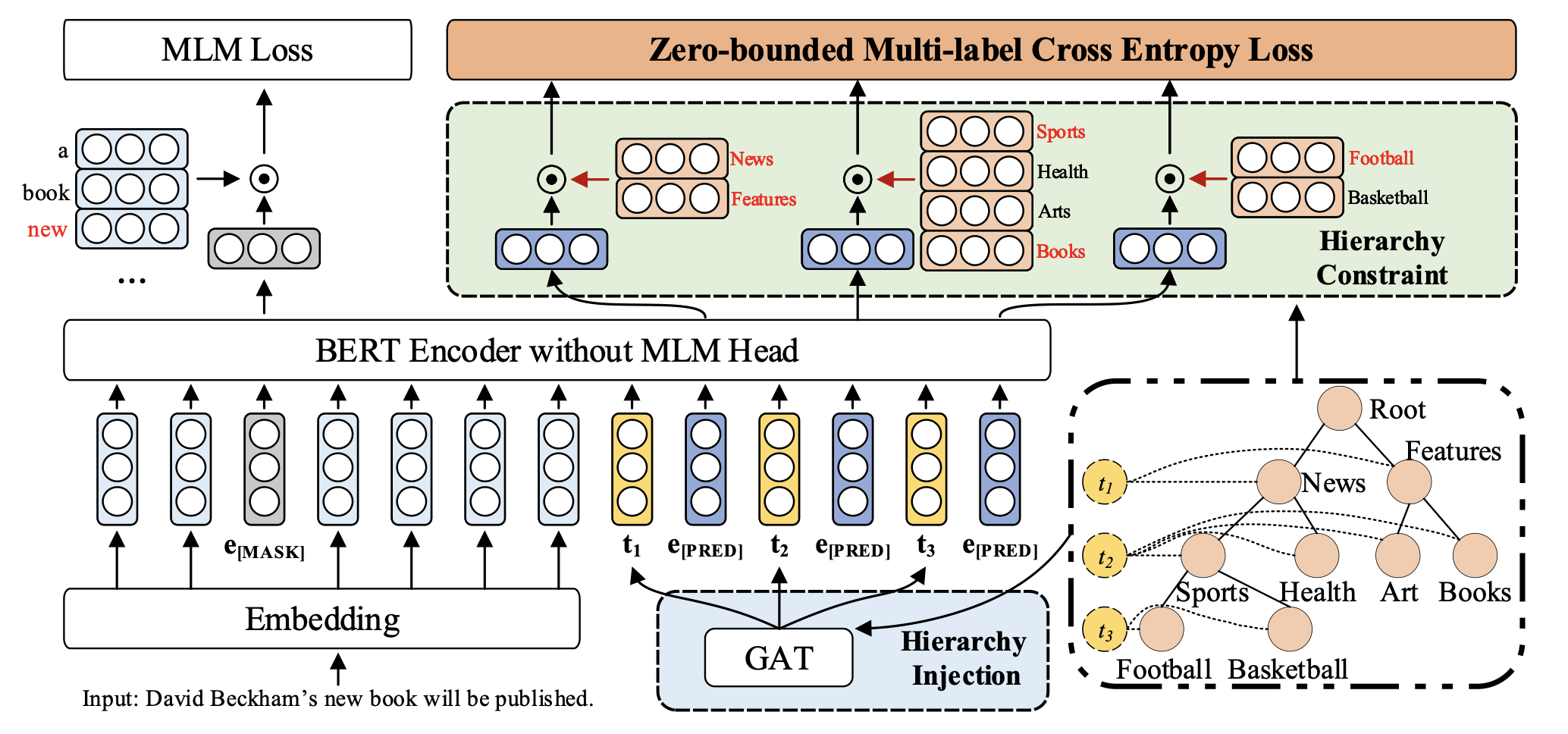## Overview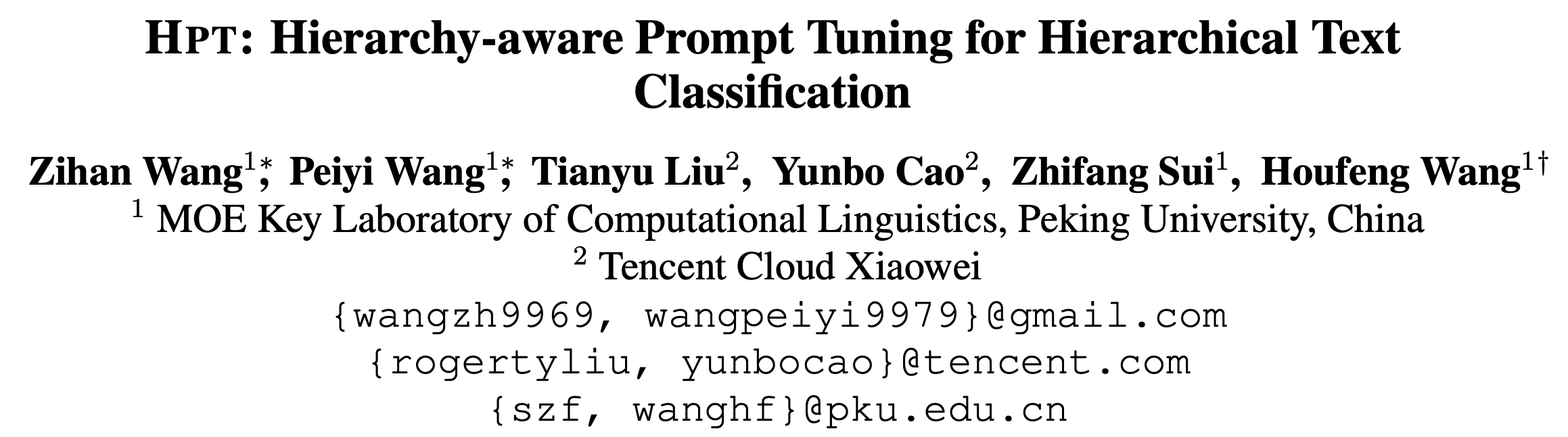## Motivation 对于微调（finetune）范式，在预训练的MLM任务与下游分类任务之间存在 gap ，为此提出了 prompt tuning 的概念，即构造模版让预训练模型做完形填空任务。例如构造模版 $$\text{x is [MASK]}$$，其中$$\text{[MASK]}$$可以代表分类中的标签，利用 MLM 预测 $$\text{[MASK]}$$ 的值，从而可以充分利用PLM的潜力。 在层次分类这种任务上，prompt 也同样适用，例如Label Mask for Multi-Label Text Classification。不过现有方法仍然存在两个问题： • Hierarchy and flat gap：局限于flat prompt，忽略了标签的层次信息。 • Multi-label and multi-class gap：MLM任务是多分类任务，而非多标签任务。 因此作者提出了 Hierarchy-aware Prompt Tuning 方法，并将 HTC 转变为多标签的 MLM 任务。 ## Method ### Perliminaries 标签层次定义为$$\mathcal{H}=(\mathcal{Y},E)$$，给定文本 $$\mathbf{x}$$，预测的标签集合 $$Y$$ 对应于 $$\mathcal{H}$$ 中的一条或多条路径。 对于传统的微调范式而言，需要在语句首尾拼接 $$[CLS]$$$$[SEP]$$，即输入形式为$ 。送入模型后，对输出的 $$h_{[CLS]}$$ 接分类器预测标签即可。 而对于Prompt Tuning，主要有两种： • Hard Prompt：人为构造的模版，如 $$\text{[CLS] } \mathbf{x}\text{ [SEP] the text is about [MASK] [SEP]}$$，模型需要预测被掩码的位置，相当于对词表中的每个词计算分数。此外还需要定义 verbalizer，即标签的映射关系。 • Soft Prompt：不需要手工构造模版，采用可学习的向量作为模版，如 $$\text{[CLS] } \mathbf{x} \text{ [SEP] [V1] [V2] [V3] [MASK] [SEP]}$$，模型预测 $$\text{[MASK]}$$ 的同时学习模版。 上述两种方式可以实现 HTC 的任务，将其转化为多个二分类任务，但存在作者提出的两个问题。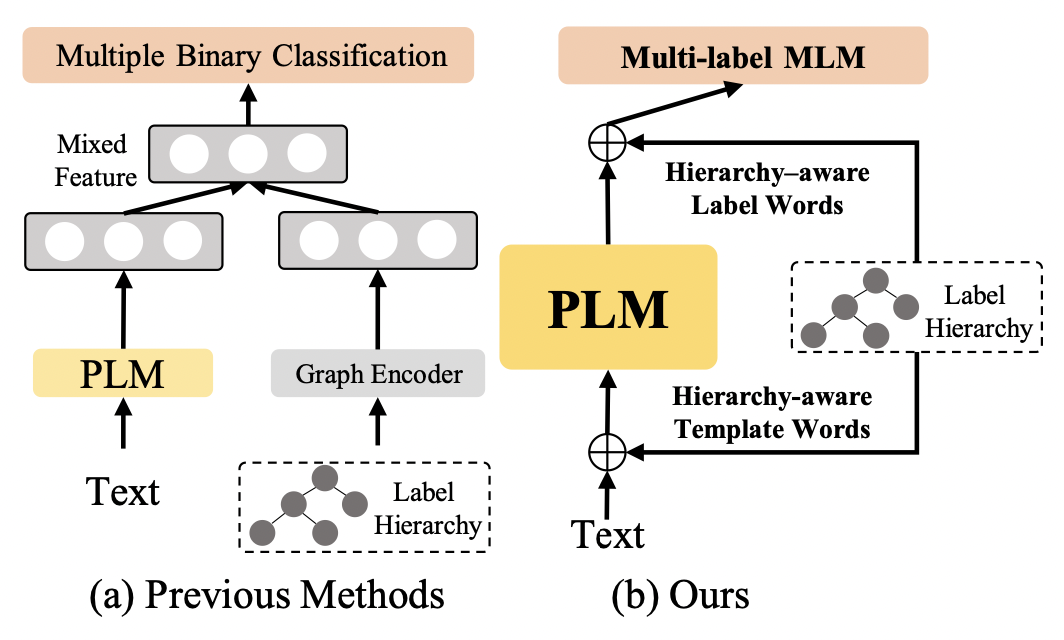### Hierarchy-aware Prompt 给定 $$L$$ 层的层级结构，构造 $$L$$ 个虚拟模版 $$\text{[V1] ... [VL]}$$ ，输入为 $$\text{[CLS] } \mathbf{x}\text{ [SEP] [V1] [PRED] ... [VL] [PRED] [SEP]}$$，其中 $$\text{[PRED]}$$ 相当于 $$\text{[MASK]}$$ 具体而言，使用 BERT 作为文本编码器，那么输入表示为： $\mathbf{T} = [\mathbf{x}_1,\cdots,\mathbf{x}_N,\mathbf{t}_1,\mathbf{e}_P,\cdots,\mathbf{t}_L,\mathbf{e}_P]$ 其中 $$\mathbf{X} = [\mathbf{x}_1,\cdots,\mathbf{x}_N]$$ 表示词向量， $$\mathbf{e}_P$$ 就是 $$\text{[PRED]}$$ 的嵌入，采用 $$\text{[MASK]}$$ 作为初始化，$$\{\mathbf{t}_i\}$$ 表示 layer-wise 的模版嵌入，采用随机初始化。采用 BERT 编码后的输出为： $\mathbf{H} = [\mathbf{h}_1,\cdots,\mathbf{h}_N,h_{\mathbf{t}_1},\mathbf{h}^1_P,\cdots,h_{\mathbf{t}_L},\mathbf{h}^2_P]$ 之后对于 verblizer，为每个标签 $$y_i$$ 采用可学习的虚拟单词 $$v_i$$，选择对应 token embedding 的均值初始化 $$\mathbf{v}_i$$，形式化为： $\text{Verb}_m(y_i) = \begin{cases}v_i,&y_i\in\mathcal{N_m}\\\varnothing,&\text{Others}\end{cases}$ 其中 $$\mathcal{N}_m$$ 表示第 $$m$$ 层的标签集合。 ### Hierarchy Injection 考虑到上述方法只引入了标签的深度信息，作者希望在模版表示中引入层次知识。为此采用 $$K$$ 层堆叠的 GAT 建模层次关系，给定在第 $$k$$ 层的节点 $$u$$，信息聚合操作定义为： $\mathbf{g}_u^{(k+1)} = \text{ReLU}\left(\sum_{v\in\mathcal{N}(u)\cup\{u\}}\frac{1}{c_u}\mathbf{W}^{(k)}\mathbf{g}_v^{(k)}\right)$ 其中 $$N(u)$$ 表示 $$u$$ 的邻居，$$c_u$$ 是一个归一化常数。 为了获取每层的知识，创建 $$L$$ 个虚拟节点 $$t_1,\cdots,t_L$$，将 $$t_i$$ 与第 $$i$$ 层所有的节点相连（有点像 $$\text{[CLS]}$$ ）。对于节点初始化，采用虚拟单词 $$\mathbf{v}_i$$ 作为节点 $$y_i\in\mathcal{Y}$$ 的特征，模版嵌入 $$\mathbf{t}_i$$ 作为 $$t_i$$ 的节点特征。 在构建的新图上施加 GAT，虚拟节点 $$t_i$$ 的输出表示为 $$g_{t_i}$$，其聚合了第 $$i$$ 层的知识，利用残差连接获得第 $$i$$ 个模版嵌入： $\mathbf{t}_i^\prime = \mathbf{t}_i + \mathbf{g}_{t_i}^K$$$\mathbf{t}_i^\prime$$ 替代原有的 $$\mathbf{t}_i$$ 送入 BERT 即可。 ### Zero-bounded Multi-label Cross Entropy Loss 采用了多标签交叉熵，参考苏神的博客 将“softmax+交叉熵”推广到多标签分类问题 具体而言，作者对每一层都计算了交叉熵： $\mathcal{L}_{ZMLCE}^m = \log(1+\sum_{y_i\in\mathcal{N}_m^n}e^{s_{y_i}}) + \log(1+\sum_{y_i\in\mathcal{N}_m^p}e^{-s_{y_i}})$ 其中 $$s_{y_i} = \mathbf{v}^T_i\mathbf{h}_P^m + b_{im}$$$$\mathcal{N}_m^p$$$$\mathcal{N}_m^n$$ 分别表示第 $$m$$ 层的正负标签集合。此外作者加了 MLM Loss，掩码比例为 15%。 $\mathcal{L}_{all} = \mathcal{L}_{MLM} + \sum_{m=1}^L\mathcal{L}_{ZMLCE}^m$ 在推理时，选择得分大于 0 的标签作为预测结果。 ## Experiments 选用 WOS NYT 和 RCV1-V2，只用了一层的 GAT。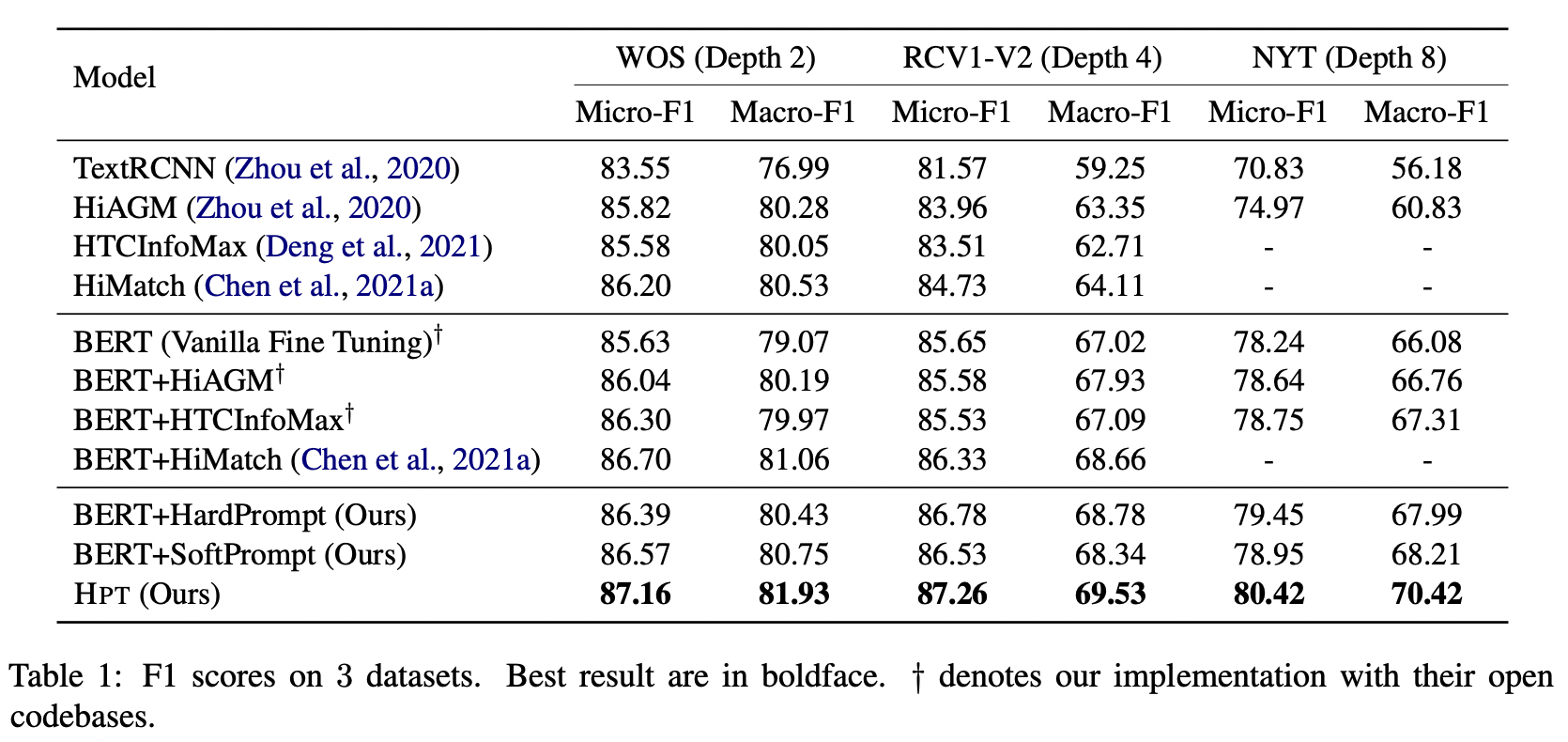从结果来看，不论是 HardPrompt 还是 SoftPrompt 都比直接微调要好，提出的方法取得了 SOTA。 消融实验验证了作者提出各个模块的有效性。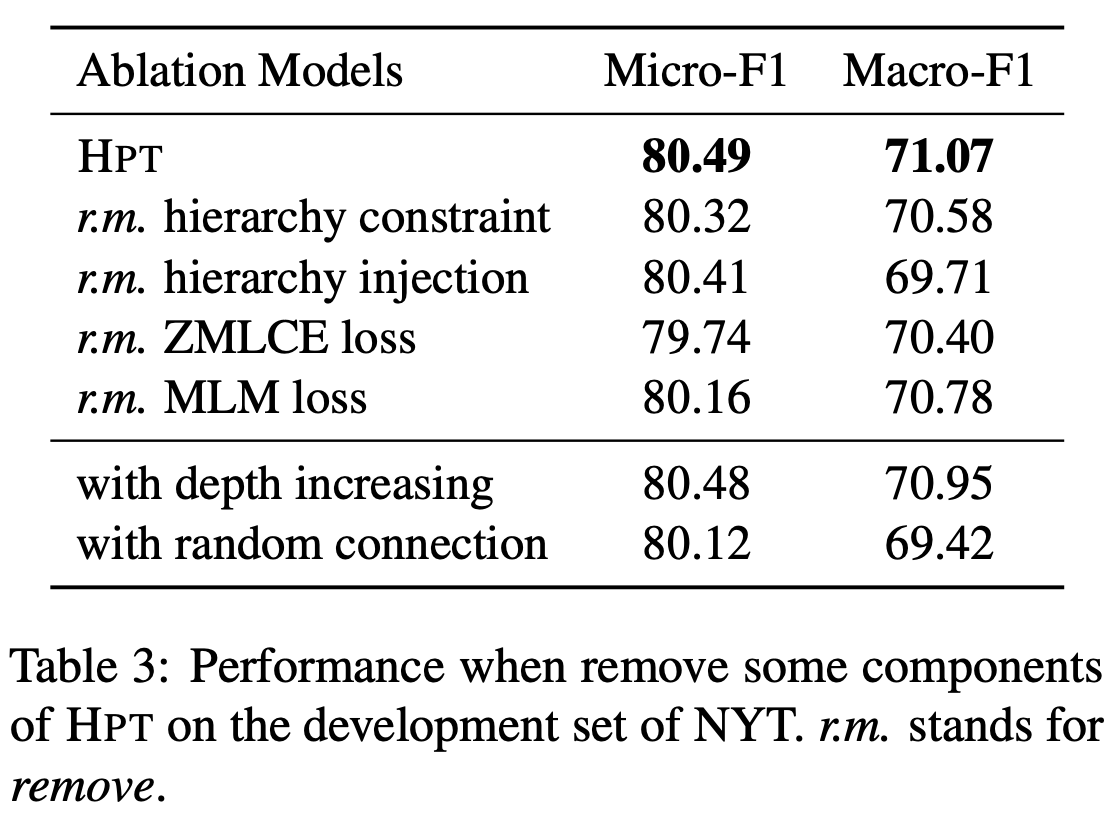作者比较了模型在数据不平衡的表现，包括每层的标签数不平衡和每个样本包含的标签数不平衡。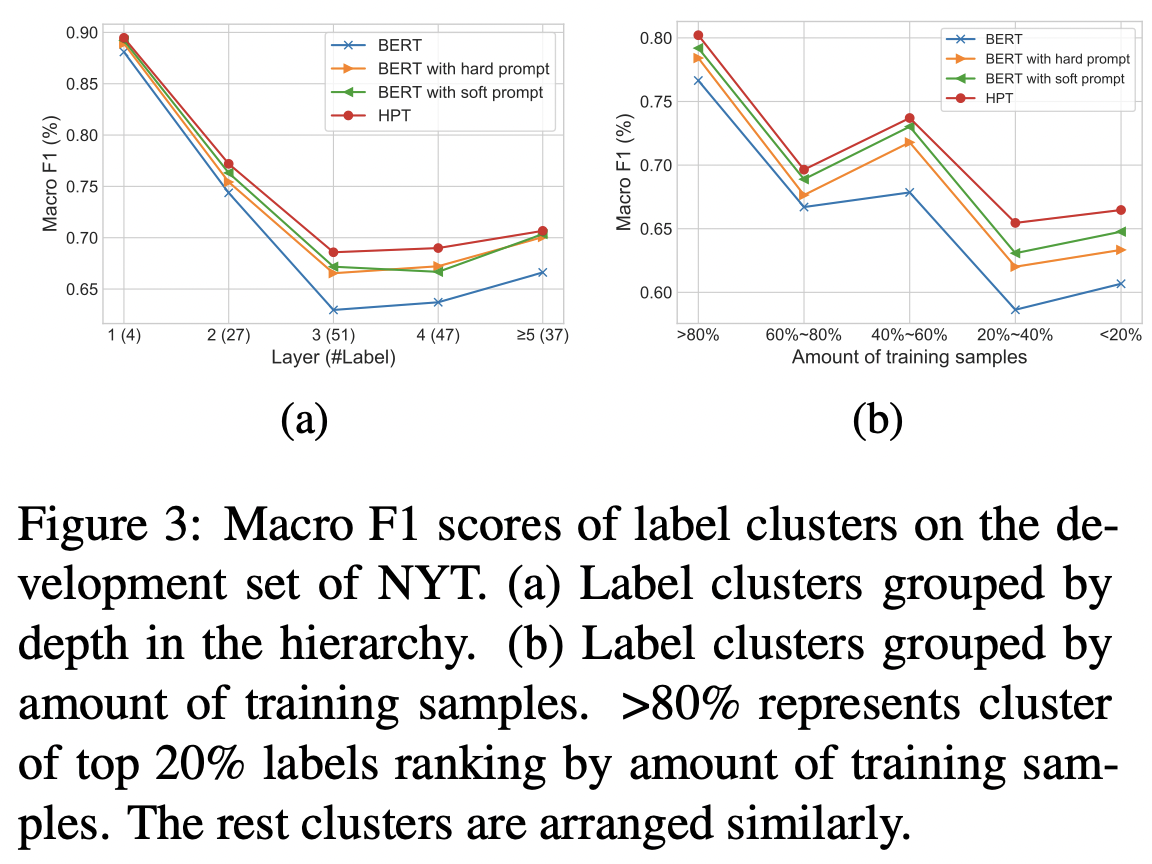此外还探讨了低资源设置下的表现，采用10%的训练数据，这也符合 prompt 本身的应用场景。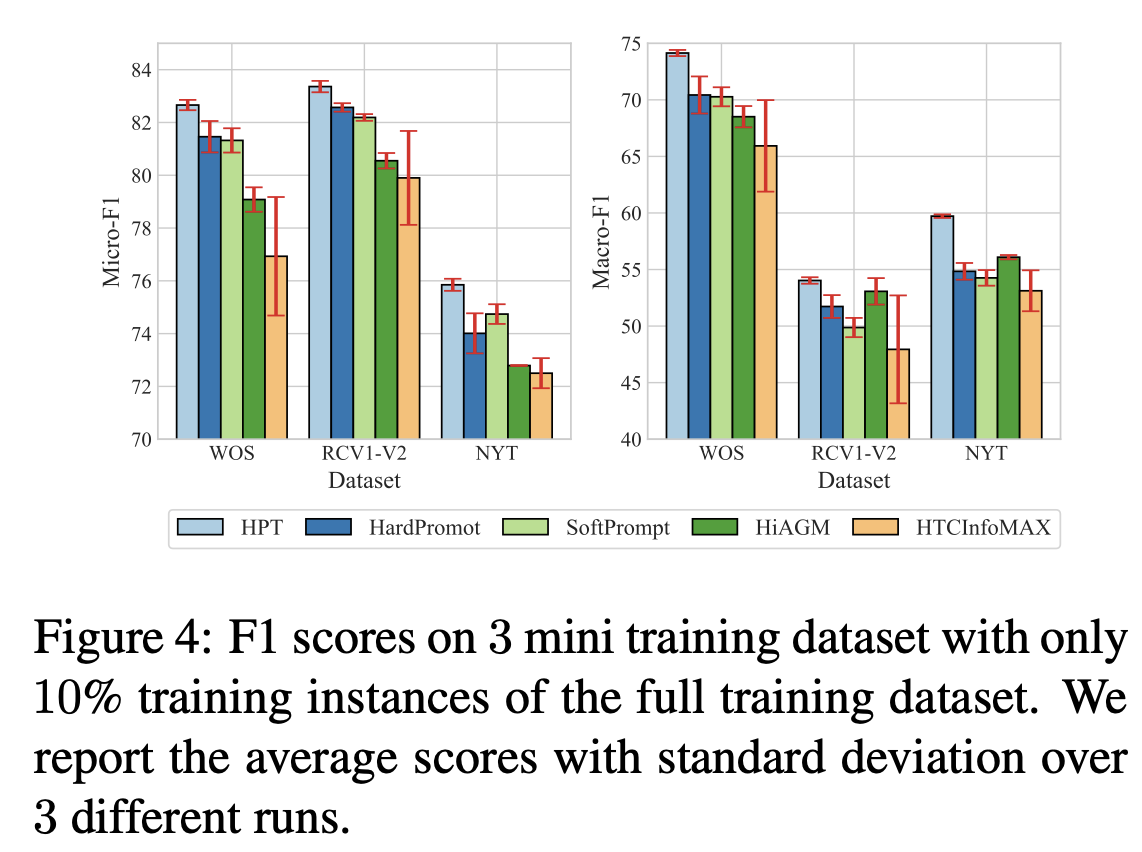]]> <p>用 Prompt 方法做层次文本分类，并融合了层次信息。</p> <p><img src="/2022/05/08/HPT-Hierarchy-aware-Prompt-Tuning-for-Hierarchical-Text-Classification/image-20220508100925643.png" alt="image-20220508100925643" style="zoom: 33%;"></p> Incorporating Hierarchy into Text Encoder: a Contrastive Learning Approach for Hierarchical Text Classification https://entropy2333.github.io/2022/03/31/Incorporating-Hierarchy-into-Text-Encoder-a-Contrastive-Learning-Approach-for-Hierarchical-Text-Classification/ 2022-03-31T08:00:57.000Z 2022-03-31T12:01:16.150Z ACL 2022，使用Graphormer与对比学习处理层次文本分类（HTC）。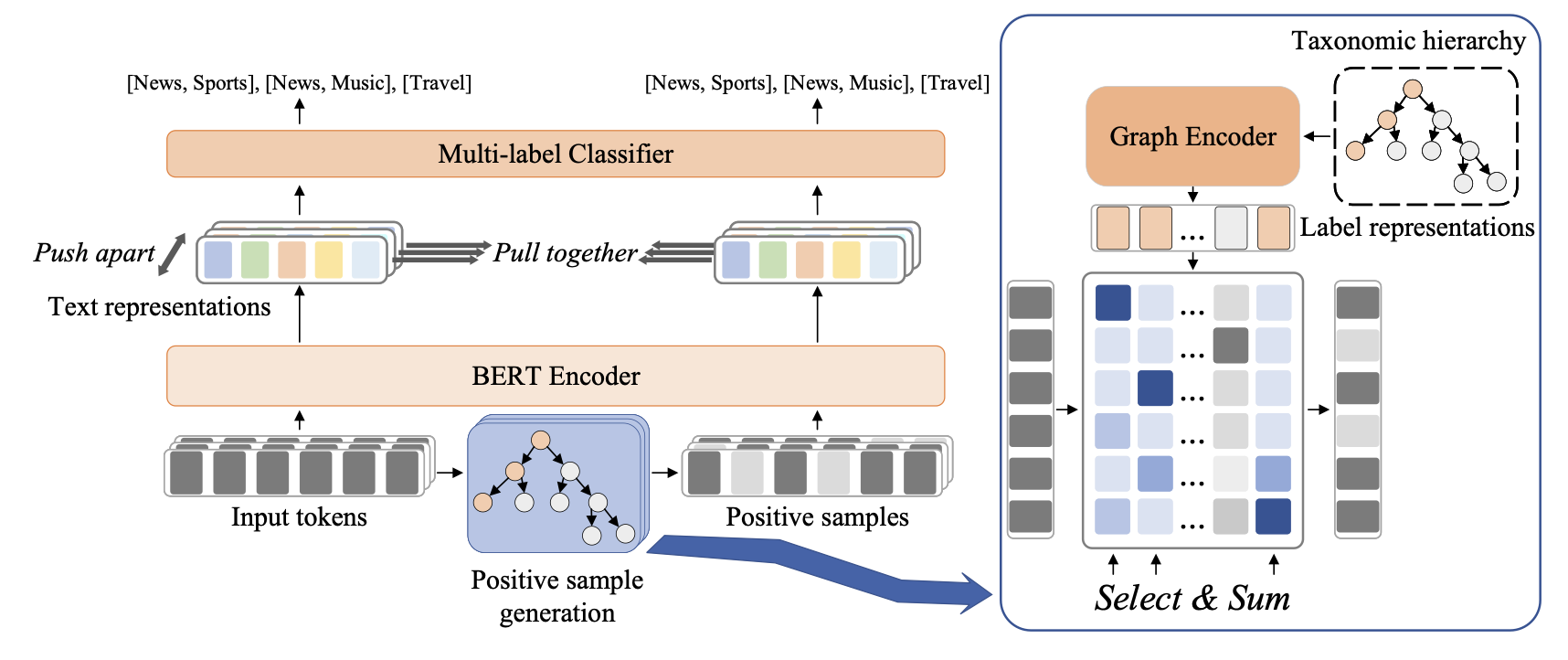## Overview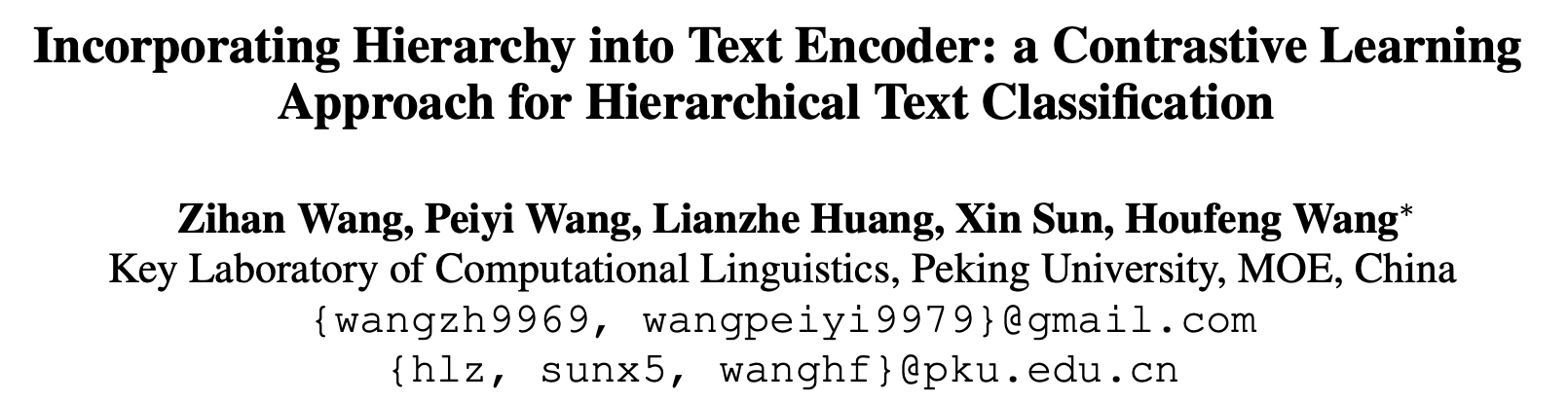## Background 现有的HTC方法已经引入了各种层次信息，主要有两种方式。 • 将文本和标签分别编码，得到混合表示。 • 将层次信息融入编码器，得到层次感知的文本表示。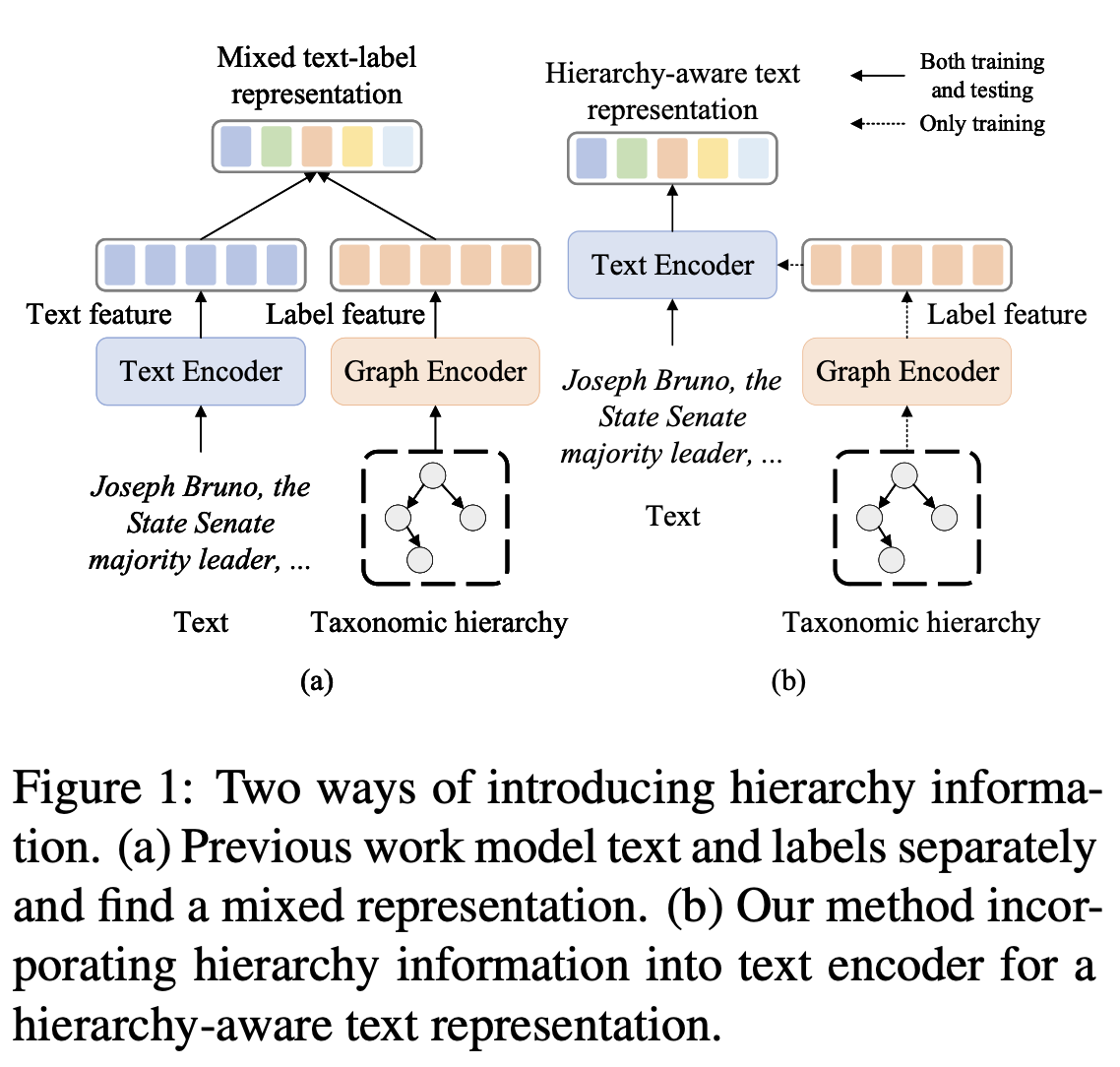本文引入了对比学习以获得层次感知的表示，尝试构建高质量的正样本。现有的方法包括数据增强和对抗攻击等，这些要么是无监督要么是任务无关的。 作者的motivation主要是：文本分类时大多数的词或token其实并不重要，而少量的关键词对结果有很大的影响。给定文本序列及其标签，只保留少量关键词的短序列也应该保持同样的标签。因此，本文的idea和对抗攻击有点像，希望找出对分类影响最大的tokens，但对抗攻击是试图修改它们，而本文修改的是不重要的tokens。 ## Method ### Text Encoder 文本嵌入采用BERT，老生常谈。 $x = \{[CLS], x_1, x_2, \cdots, x_{n-2}, [SEP]\}$ 文本表示$$H\in\mathbb{R}^{n\times d_h}$$，采用$$h_x=h_{[CLS]}$$作为句子表示 $H = \mathrm{BERT}(x)$ ### Graph Encoder 本文采用Graphormer建模标签的层次关系，在Transformer的基础上使用spatial encoding和edge encoding建模图结构。节点特征是标签嵌入和名称嵌入的和： $f_i = \mathrm{label\_emb}(y_i) + \mathrm{name\_emb}(y_i)$ 标签嵌入是可学习的，名称嵌入利用了标签的名称（其中可能蕴含了丰富信息），采用BERT编码得到，最终得到所有节点特征$$F\in\mathbb{R}^{k\times d_h}$$ 为了利用结构信息，采用Graphormer中魔改的self-attention $A_{ij}^{G} = \frac{(f_iW_Q^G)(f_jW_K^G)^T}{\sqrt{d_h}} + c_{ij} + b_{\phi(y_i, y_j)}$ 其中$$c_{ij} = \frac1D\sum_{n=1}^Dw_{e_n}$$$$D=\phi(y_i,y_j)$$$$A_{ij}^G$$中的第一项是标准的点积注意力，$$c_{ij}$$表示edge encoding，$$\phi(y_i,y_j)$$表示两个节点间的距离。 因为在HTC问题中，图实际上是一个树，因此两个节点间只有一条路径$$(e_1, e_2, \cdots, e_D)$$，所以$$c_{ij}$$表示了两个节点间的边信息，$$w_{e_i}\in\mathbb{R}^1$$是可学习的权重。$$b_{\phi(y_i, y_j)}$$为spatial encoding，衡量了两个节点间的连通性。 和Transformer里一样，对注意力做softmax后LayerNorm即可。 $L = \mathrm{LayerNorm}(\mathrm{softmax}(A^G)V+F)$ ### Positive Sample Generation 对于对比学习来说，最重要的就是构建正样本。这一步的目标是保留部分token，同时维持标签不变。对于BERT的token embedding来说： $\{e_1,e_2,\cdots,e_n\} = \mathrm{BERT\_emb}(x)$ 可以用cross attention衡量token与label之间的相关性 $q_i = e_iW_Q,k_j=l_jW_K,A_{ij}=\frac{q_ik_j^T}{\sqrt{d_h}}$ 其中$$W_Q, W_K\in\mathbb{R}^{d_h\times d_h}$$为权重矩阵。对注意力矩阵计算Softmax即可得到token $$x_i$$属于标签$$y_i$$的概率，给定$$y_j$$可以从分布中采样关键token，构建正样本$$\hat{x}$$。为了使得采样操作可微，采用Gumbel-Softmax代替Softmax。 $P_{ij} = \mathrm{gumbel\_softmax}(A_{i1},A_{i2},\cdots,A_{ik})_j$ Gumbel-Softmax是一种重参数化（Reparameterization）的技巧，可以参考苏神的讲解 将所有真实标签的概率相加，作为token $$x_i$$属于真实标签集合 $$y$$的概率 $P_i = \sum_{j\in y}P_{ij}$ 最终的正样本通过门限控制得到 $\hat{x} = \{x_i\ \text{if}\ P_i > \gamma\ \text{else}\ \mathbf{0}\}$ 其中$$\mathbf{0}$$表示全零向量，保持关键词的位置不变。因为选择操作也不可微，所以作者还给出了一种等价实现： $\hat{e_i} = e_i((P_i+Detach(1-P_i))\ \text{if}\ P_i > \gamma \ \text{else}\ 0）$ 其中$$\hat{e_i}$$要么为$$e_i$$要么为0，从而对应的梯度为 $\frac{\partial \hat{e}_i}{\partial P_i} = e_i\ \text{if}\ P_i > \gamma \ \text{else} \ 0$ 将构建的正样本也送入BERT，得到表示 $\hat{H} = \mathrm{BERT}(\hat{x})$ ### Contrastive Learning Module 至此，已经有了文本序列和缩减字符的正样本，它们的句子表示应当相似。给定$$N$$个正样本对$$(h_i,\hat{h_i})$$，首先过一层全连接 \begin{align} c_i &= W_2\mathrm{ReLU}(W_1h_i) \\ \hat{c_i} &= W_2\mathrm{ReLU}(W_1\hat{h_i})\end{align} 其中$$W_1, W_2\in\mathbb{R}^{d_h\times d_h}$$。对于每个样本来说，同一个batch内的其他样本都是负例，所以共有$$2(N-1)$$个负样本对。之后和SimCSE一样，采用交叉熵计算 $\begin{equation} L_m^{con}=-\log\frac{\exp ({\rm sim}(z_m, \mu(z_m))/\tau)}{\sum^{2N}_{i=1,i\not= m}\exp ({\rm sim}(z_m, z_i)/\tau)}\end{equation}$ 其中$$\rm{sim}$$为cosine相似度，$${\rm sim}(u, v)=u\cdot v/ \|u\|\|v\|$$$$\mu$$为匹配函数： $\begin{equation} \mu(z_m)=\left\{ \begin{array}{ll} c_i, \text{ if } z_m=\hat{c_i}&\\ \hat{c_i}, \text{ if } z_m=c_i& \end{array} \right.\end{equation}$ 对比损失是所有样本的和 $\begin{equation} L^{con}=\frac{1}{2N}\sum_{m=1}^{2N}L_m^{con}\end{equation}$ 至于HTC任务，全连接+BCE即可。 $\begin{equation}L_{ij}^C=-y_{ij}\log(p_{ij})-(1-y_{ij})\log(1-p_{ij})\end{equation}$ $\begin{equation} L^C=\sum_{i=1}^N\sum_{j=1}^kL_{ij}^C\end{equation}$ 最终的损失函数是三者的加权和，在推理时模型退化为BERT+分类头。 $\begin{equation} L=L^C+\hat{L^C}+\lambda L^{con}\end{equation}$ ## Experiment 选择了WOS、NYT和RCV1-V2数据集，使用bert-base-uncased作为基准模型。对于Graphormer，设置8个头，768的特征维度。batch size设置为12，学习率3e-5。 对于WOS，$$\gamma$$为0.02，对于NYT和RCV1-V2，$$\gamma$$为0.005。WOS和RCV1-V2的$$\lambda$$为0.1，NYT的$$\lambda$$为0.3。 之前的部分模型采用TextRCNN作为文本编码器，作者也给出了BERT的结果。值得一提的是，作者没有复现出HiMatch的BERT结果，因此采用的是自己的结果。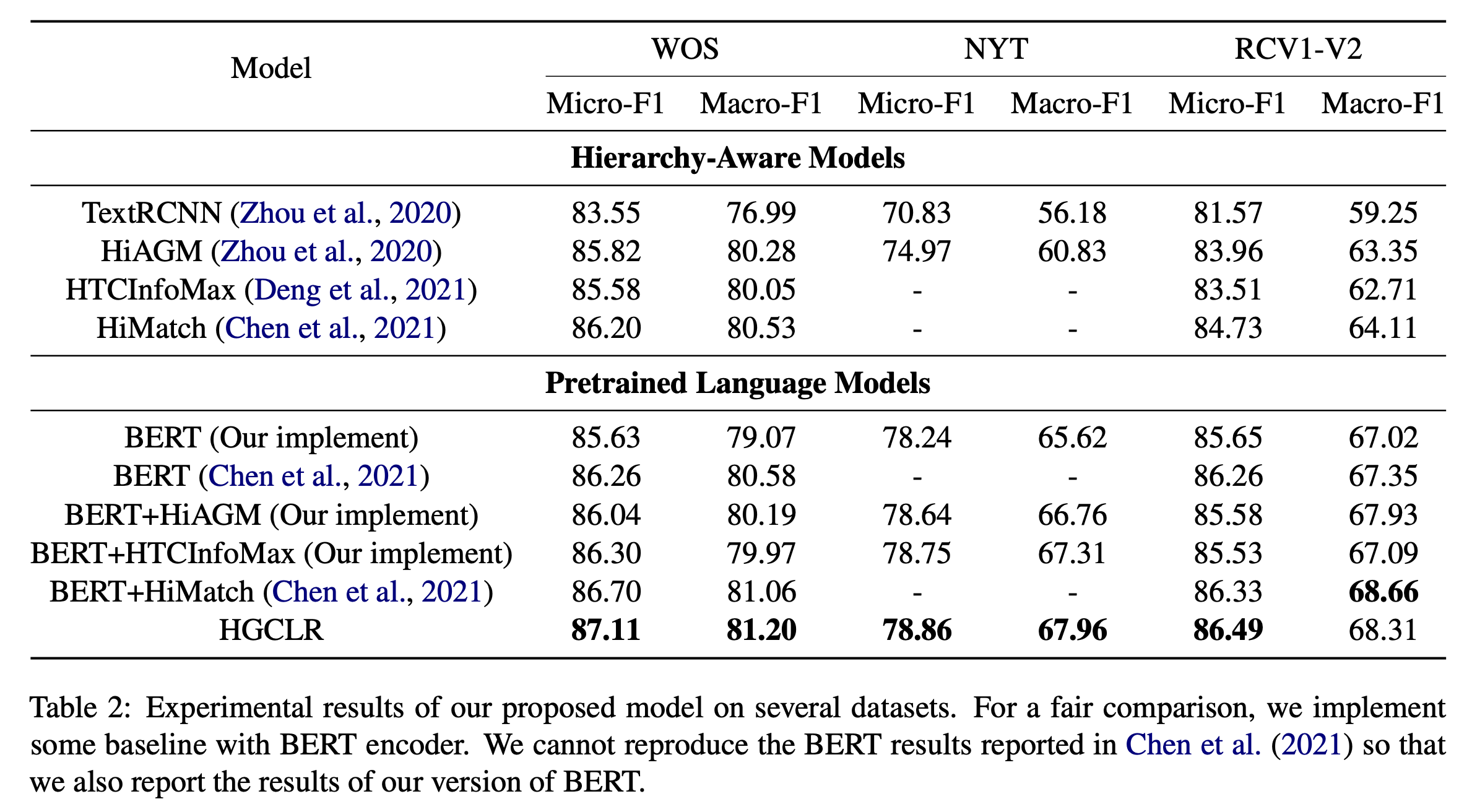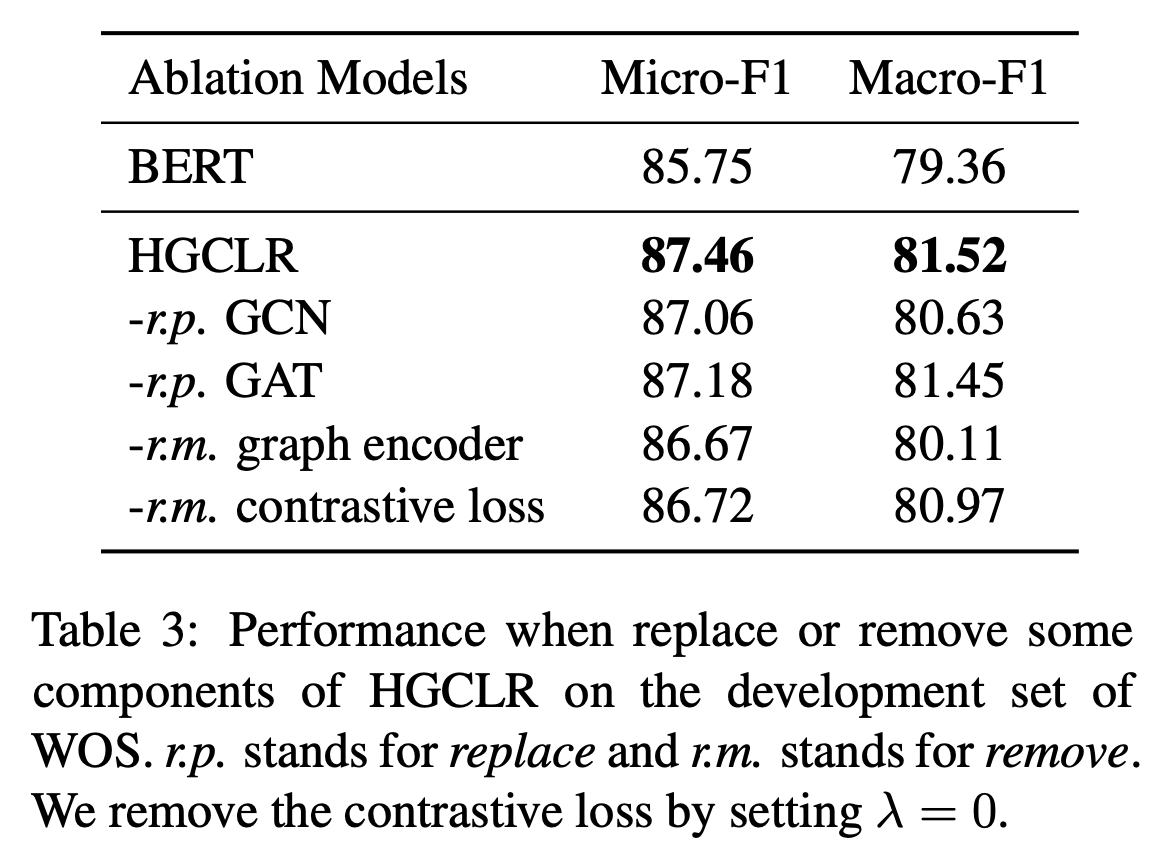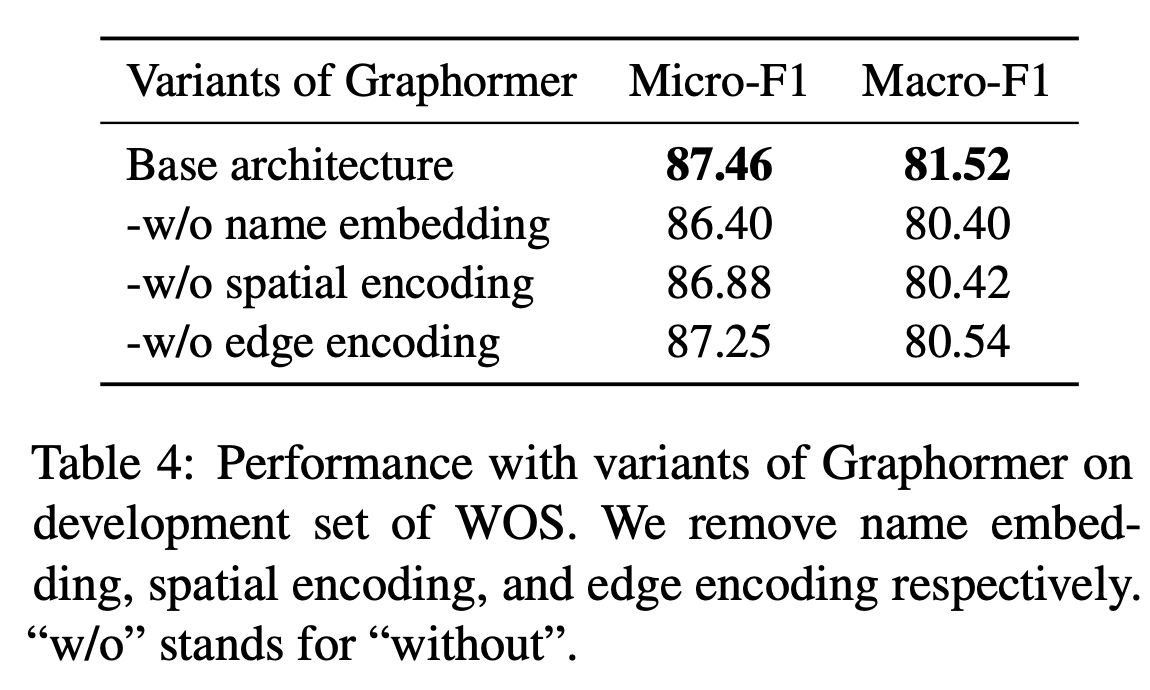]]> <p>ACL 2022，使用Graphormer与对比学习处理层次文本分类（HTC）。</p> <p><img src="/2022/03/31/Incorporating-Hierarchy-into-Text-Encoder-a-Contrastive-Learning-Approach-for-Hierarchical-Text-Classification/image-20220331164335199.png" alt="image-20220331164335199" style="zoom:50%;"></p> Hierarchy-aware Label Semantics Matching Network for Hierarchical Text Classification https://entropy2333.github.io/2022/03/21/Hierarchy-aware-Label-Semantics-Matching-Network-for-Hierarchical-Text-Classification/ 2022-03-21T08:29:51.000Z 2022-04-04T11:36:15.985Z ACL 2021，提出层次感知的标签语义匹配网络处理层次多标签分类（HTC）问题。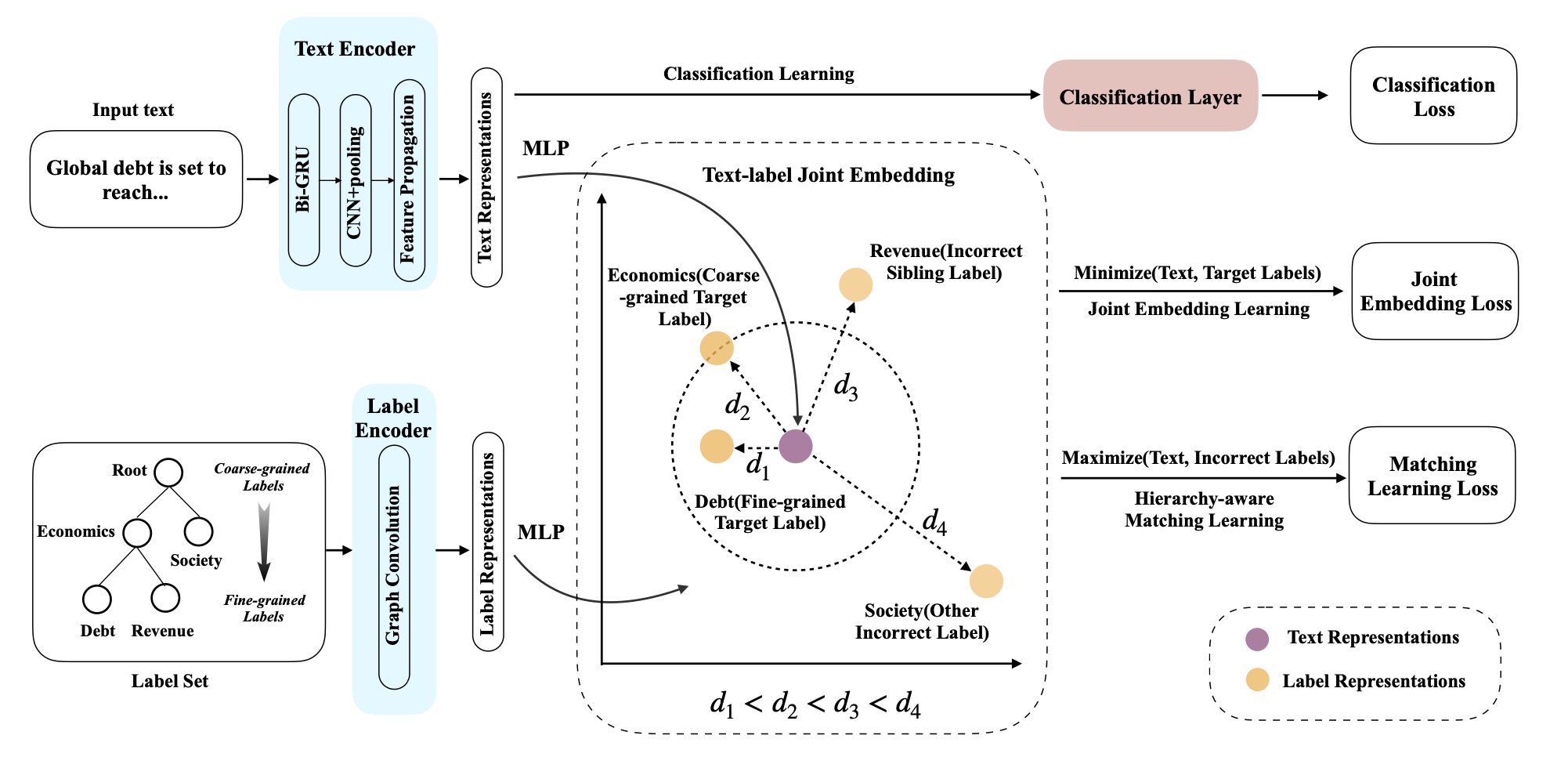## Overview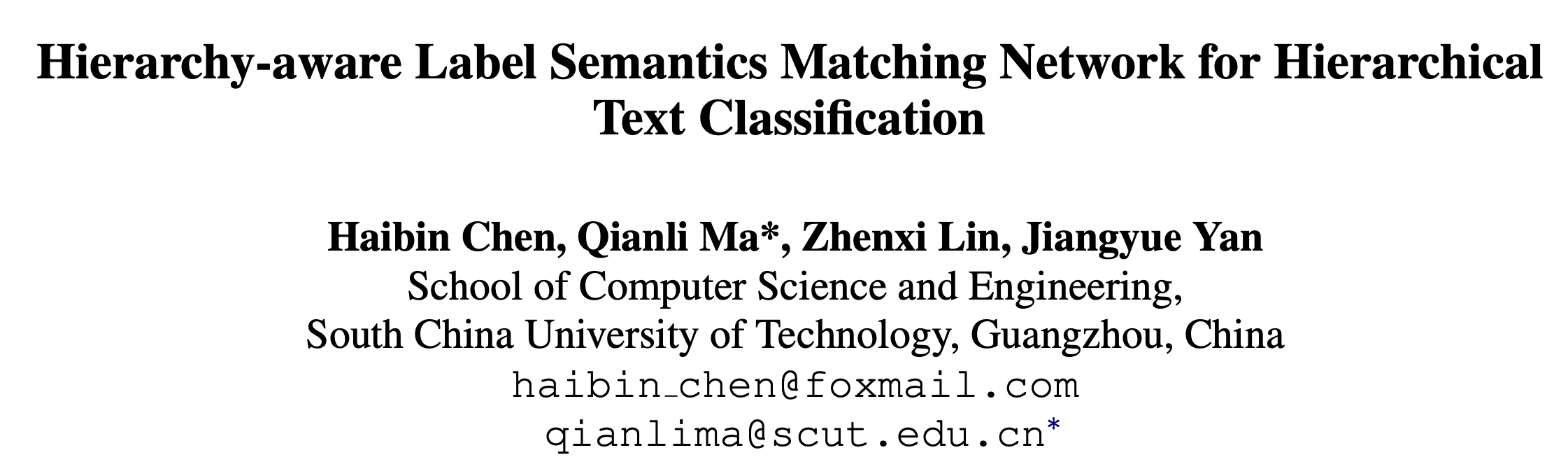## Background 有点类似对比学习的思想，将HTC问题形式化为一个语义匹配问题，希望文本表示与目标标签的表示更接近，与不相关的标签表示距离更远。 ## Method 参考HiAGM，首先分别用LSTM和GCN对文本和标签提取特征，作者这里对文本也用了GCN进一步提取特征，称为hierarchy-aware text feature propagation module。 \begin{align}S_t = \textrm{ReLU}\left(\overleftarrow{E}\cdot V_t\cdot W_{g_1}+ \overrightarrow{E}\cdot V_t\cdot W_{g_2}\right) \\S_l = \textrm{ReLU}\left(\overleftarrow{E}\cdot V_l\cdot W_{g_3}+ \overrightarrow{E}\cdot V_l\cdot W_{g_4}\right)\end{align} 其中$$E\in\mathbb{R}^{k\times k}$$表示父子节点的先验转移概率，$$V$$表示节点特征，$$W$$为GCN的权重。 在得到文本和标签的表示后，作者使用联合嵌入将二者映射到同一潜在空间。 \begin{align}\Phi_t = \textrm{FFN}_t(S_t) \\\Phi_l = \textrm{FFN}_l(S_l) \\\end{align} 为了对齐表示，采用MSE作为损失函数。 $L_{joint} = \sum_{p\in P(y)}\lVert\Phi_t - \Phi_l^p\rVert_2^2$ 除了文本和标签之间的相关性，还需要考虑不同粒度标签的相关性，因此作者提出了一种margin-based triplet loss来衡量文本表示与标签之间的相关性。 $L_{match} = \max(0,D(\Phi_t, \Phi_l^p)-D(\Phi_t, \Phi_l^n)+\gamma)$ 其中$$\Phi_l^p$$表示目标标签语义，$$\Phi_l^n$$表示其他标签的语义，采用的是L2归一化的欧式距离，$$\gamma$$表示门限。 对Margin Loss的理解可参考https://zhuanlan.zhihu.com/p/158853633 此外，为了提高计算效率，作者还进行了采样。为每个细粒度标签采样了所有父节点（粗粒度标签）和一个兄弟标签，并随机选择一个不正确标签得到负样本集$$n\in N(y)$$ 在采样了标签对后，为每个标签对设置相同的margin也不合理。作者的想法是，如果两个标签在层次结构中更接近，那么其语义也应该更近。为此作者提出了四个距离 1. $$d_1$$应当为最小距离，表示正例，其他表示负例。 2. $$d_2$$表示文本与粗粒度标签的语义距离。 3. $$d_3$$表示文本与不正确的细粒度标签的距离。 4. $$d_4$$应当是最大的距离。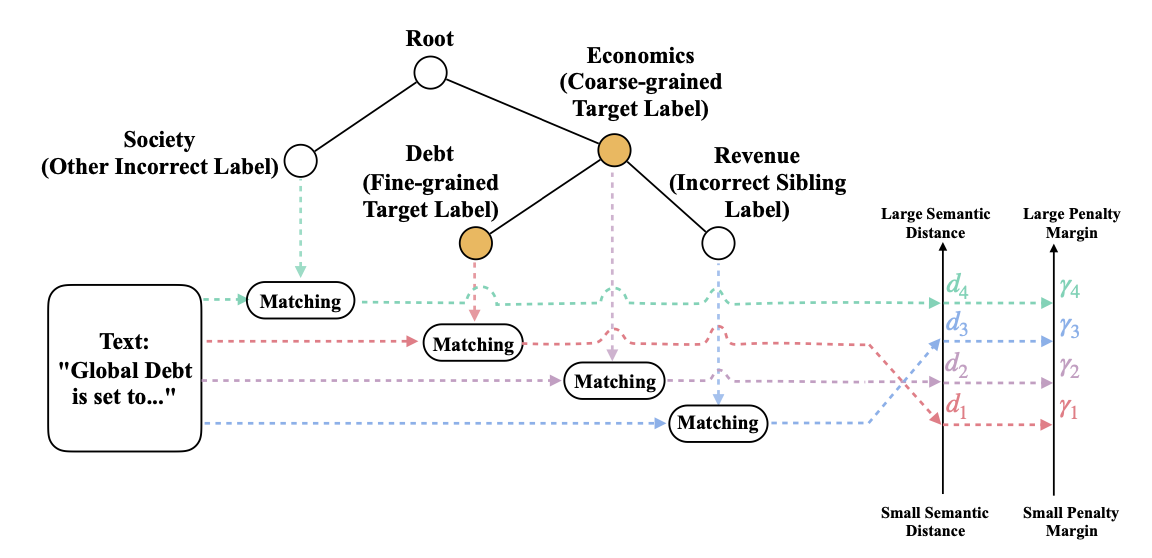针对上述，引入了四个margin $$\gamma_1,\gamma_2,\gamma_3,\gamma_4$$建模这种比较关系，随着距离的增大惩罚的margin也更大。作者在此忽略了$$\gamma_1$$，因为文本与细粒度标签的匹配关系已经在联合嵌入中得到充分的利用。 $\gamma_2 = \alpha\gamma;\quad\gamma_3=\beta\gamma;\quad\gamma_4=\gamma$ 最终的损失函数是三者的加权 $\mathcal{L} = \mathcal{L}_{cls}(y,\hat{y})+\lambda_1\mathcal{L}_{joint}+\lambda_2\mathcal{L}_{match}$ ## Experiment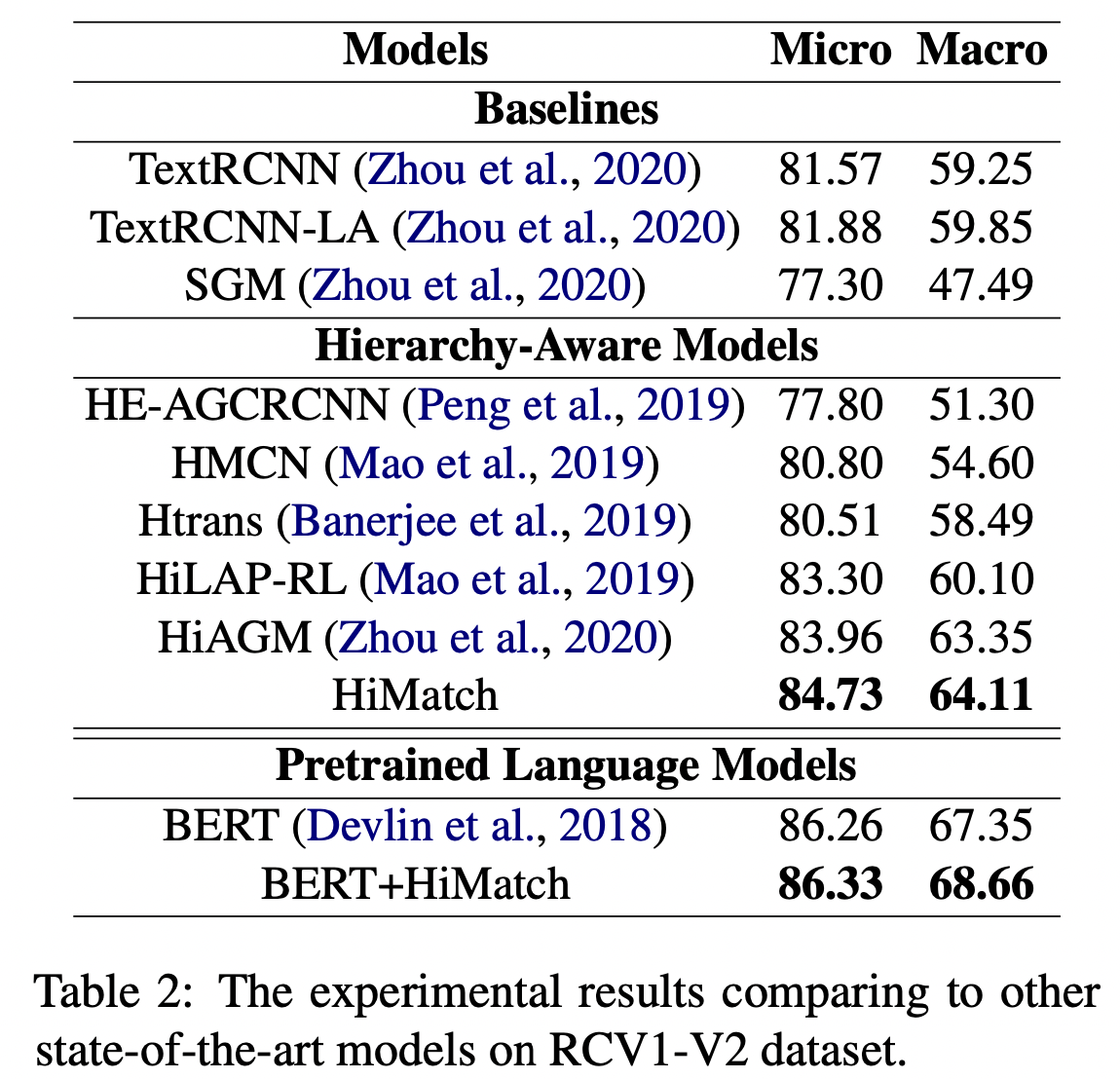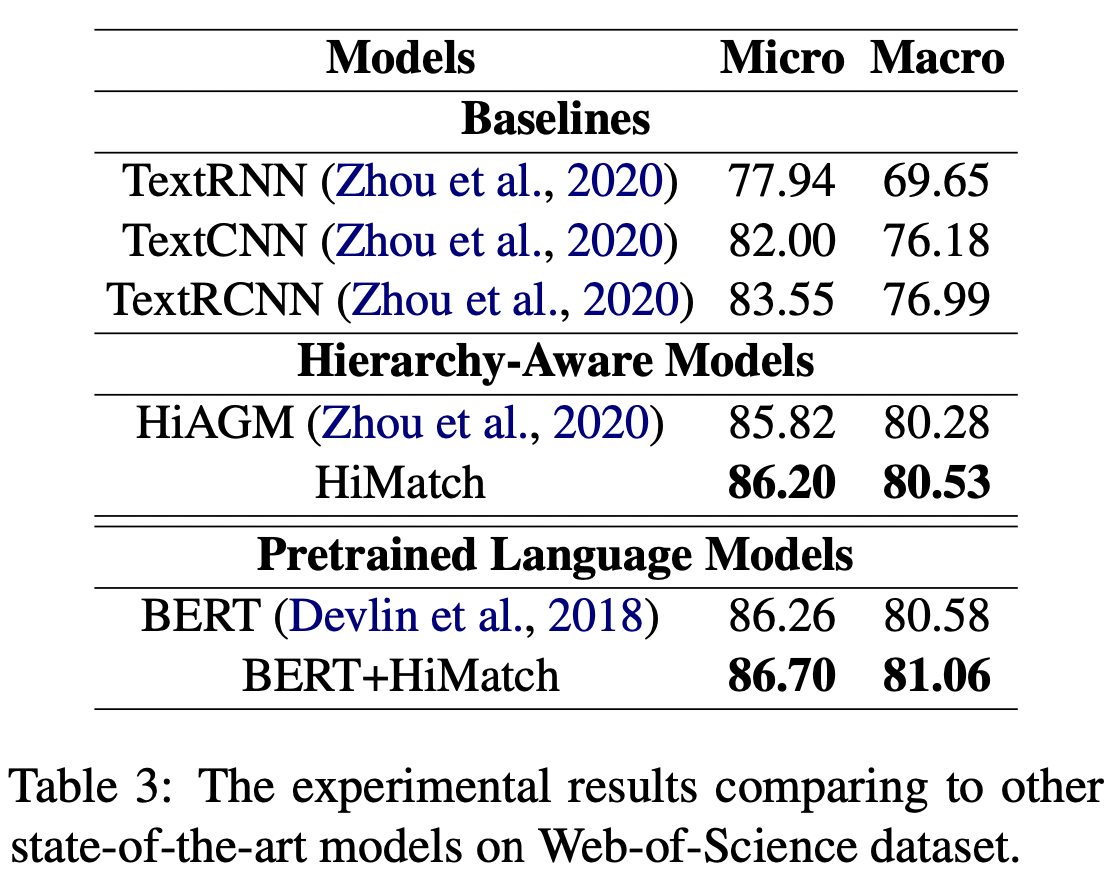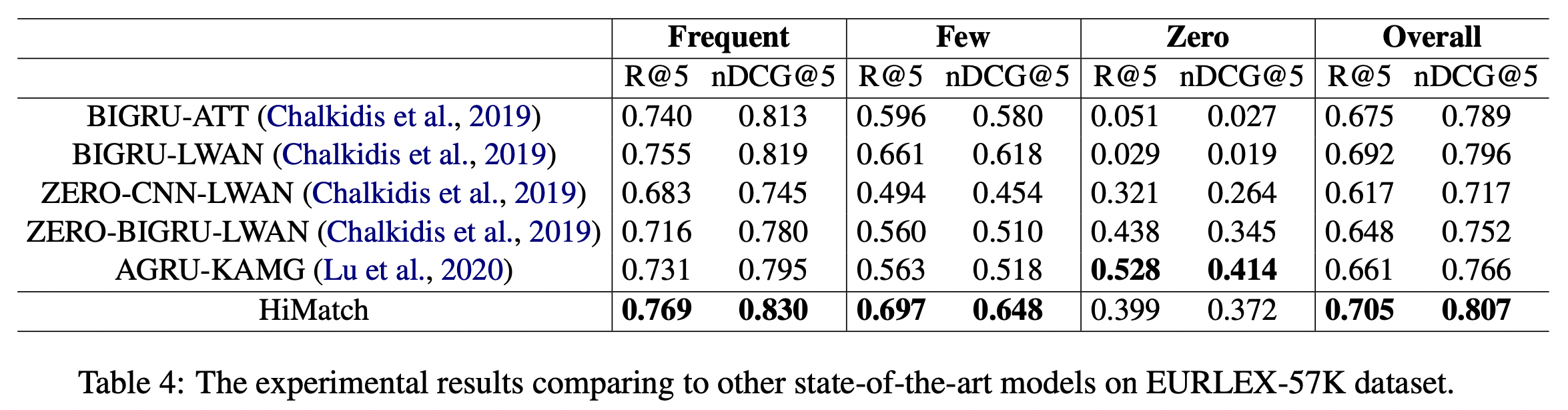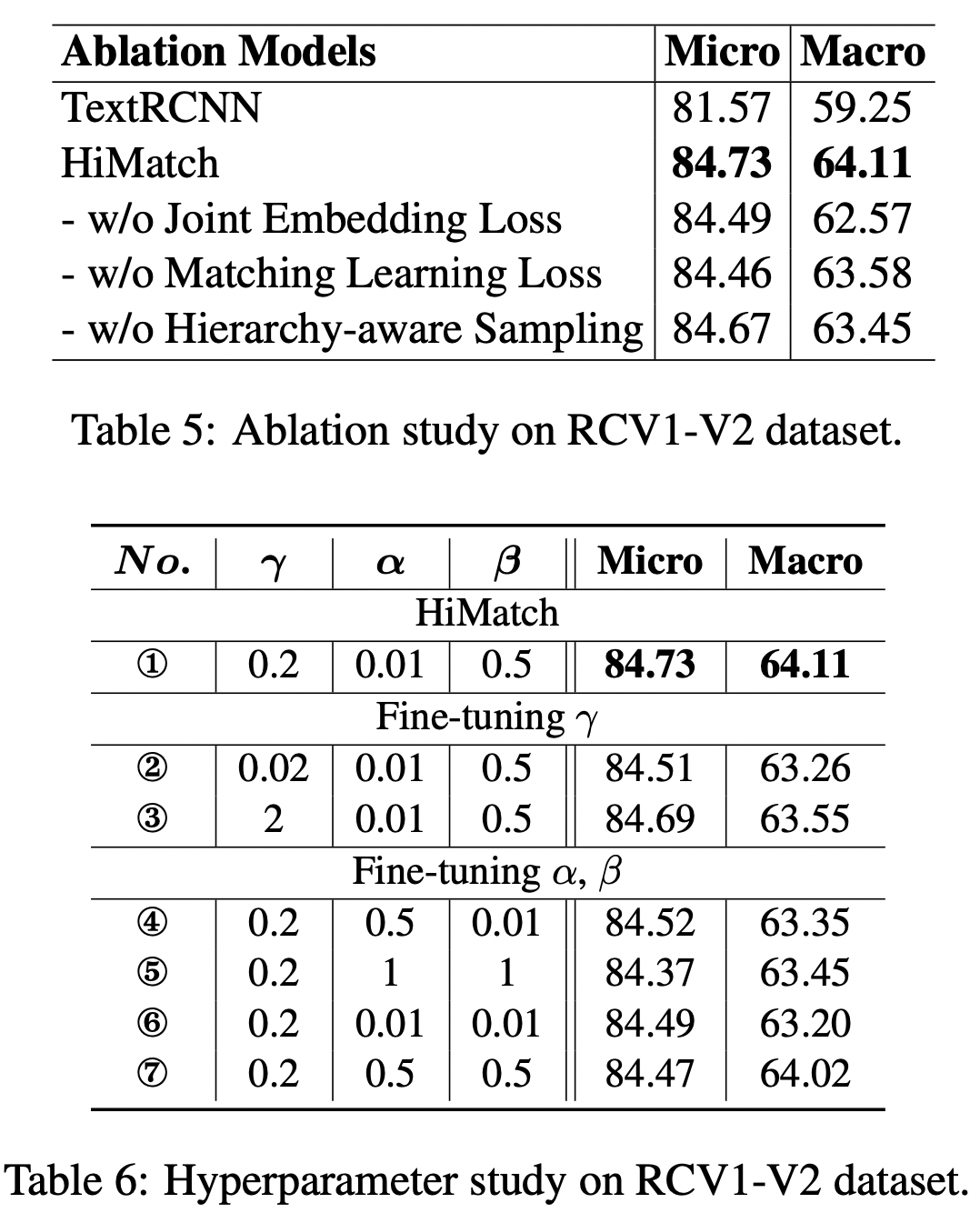## Implementation Margin Loss的实现 class MarginRankingLoss(torch.nn.Module): def __init__(self, config): """ Criterion loss default torch.nn.MarginRankingLoss(0.01) """ super(MarginRankingLoss, self).__init__() self.dataset = config.data.dataset base = 0.2 self.ranking = [torch.nn.MarginRankingLoss(margin=base*0.1), torch.nn.MarginRankingLoss(margin=base * 0.5), torch.nn.MarginRankingLoss(margin=base)] self.negative_ratio = config.data.negative_ratio def forward(self, text_repre, label_repre_positive, label_repre_negative, mask=None): """ :param text_repre: torch.FloatTensor, (batch, hidden) :param label_repre_positive: torch.FloatTensor, (batch, hidden) :param label_repre_negative: torch.FloatTensor, (batch, sample_num, hidden) :param mask: torch.BoolTensor, (batch, negative_ratio, negative_number), the index of different label """ loss_inter_total, loss_intra_total = 0, 0 text_score = text_repre.unsqueeze(1).repeat(1, label_repre_positive.size(1), 1) loss_inter = (torch.pow(text_score - label_repre_positive, 2)).sum(-1) loss_inter = F.relu(loss_inter / text_repre.size(-1)) loss_inter_total += loss_inter.mean() for i in range(self.negative_ratio): m = mask[:, i] m = m.unsqueeze(-1).repeat(1, 1, label_repre_negative.size(-1)) label_n_score = torch.masked_select(label_repre_negative, m) label_n_score = label_n_score.view(text_repre.size(0), -1, label_repre_negative.size(-1)) text_score = text_repre.unsqueeze(1).repeat(1, label_n_score.size(1), 1) # index 0: parent node if i == 0: loss_inter_parent = (torch.pow(text_score - label_n_score, 2)).sum(-1) loss_inter_parent = F.relu((loss_inter_parent-0.01) / text_repre.size(-1)) loss_inter_total += loss_inter_parent.mean() else: # index 1: wrong sibling, index 2: other wrong label loss_intra = (torch.pow(text_score - label_n_score, 2)).sum(-1) loss_intra = F.relu(loss_intra / text_repre.size(-1)) loss_gold = loss_inter.view(1, -1) loss_cand = loss_intra.view(1, -1) ones = torch.ones(loss_gold.size()).to(loss_gold.device) loss_intra_total += self.ranking[i](loss_gold, loss_cand, ones) return loss_inter_total, loss_intra_total mask的生成 for sample_i, sample in enumerate(batch): batch_token.append(sample['token']) batch_label.append(sample['label']) batch_doc_len.append(sample['token_len']) if self.mode == "TRAIN": positive = np.zeros((self.positive_sample_num)) negative = np.zeros((self.negative_sample_num)) margin = np.zeros((self.negative_ratio, self.negative_sample_num)) for i in range(self.positive_sample_num): positive[i] = sample['positive_sample'][i] for i in range(self.negative_sample_num): negative[i] = sample['negative_sample'][i] # use mask matrix 'margin' to differentiate different sampling label margin[sample['margin'][i], i] = 1 batch_ranking_positive_mask.append(positive) batch_ranking_negative_mask.append(negative) batch_margin_mask.append(margin) ]]> <p>ACL 2021，提出层次感知的标签语义匹配网络处理层次多标签分类（HTC）问题。</p> <p><img src="/2022/03/21/Hierarchy-aware-Label-Semantics-Matching-Network-for-Hierarchical-Text-Classification/image-20220321163555813.png" alt="image-20220321163555813" style="zoom:33%;"></p> TensorRT部署步骤 https://entropy2333.github.io/2022/03/19/TensorRT%E9%83%A8%E7%BD%B2%E6%AD%A5%E9%AA%A4/ 2022-03-19T05:24:24.000Z 2022-03-19T16:30:22.979Z 记录一下用TensorRT部署一个基于Swin-Transformer的Pytorch模型的步骤，以作备忘。 ## 概览 TensorRT是英伟达推出的模型加速工具，只负责模型的推理（inference）过程。具体的优化部分可以查阅官网资料，比如用FP16或INT8推理、层与张量的融合、内核调优等。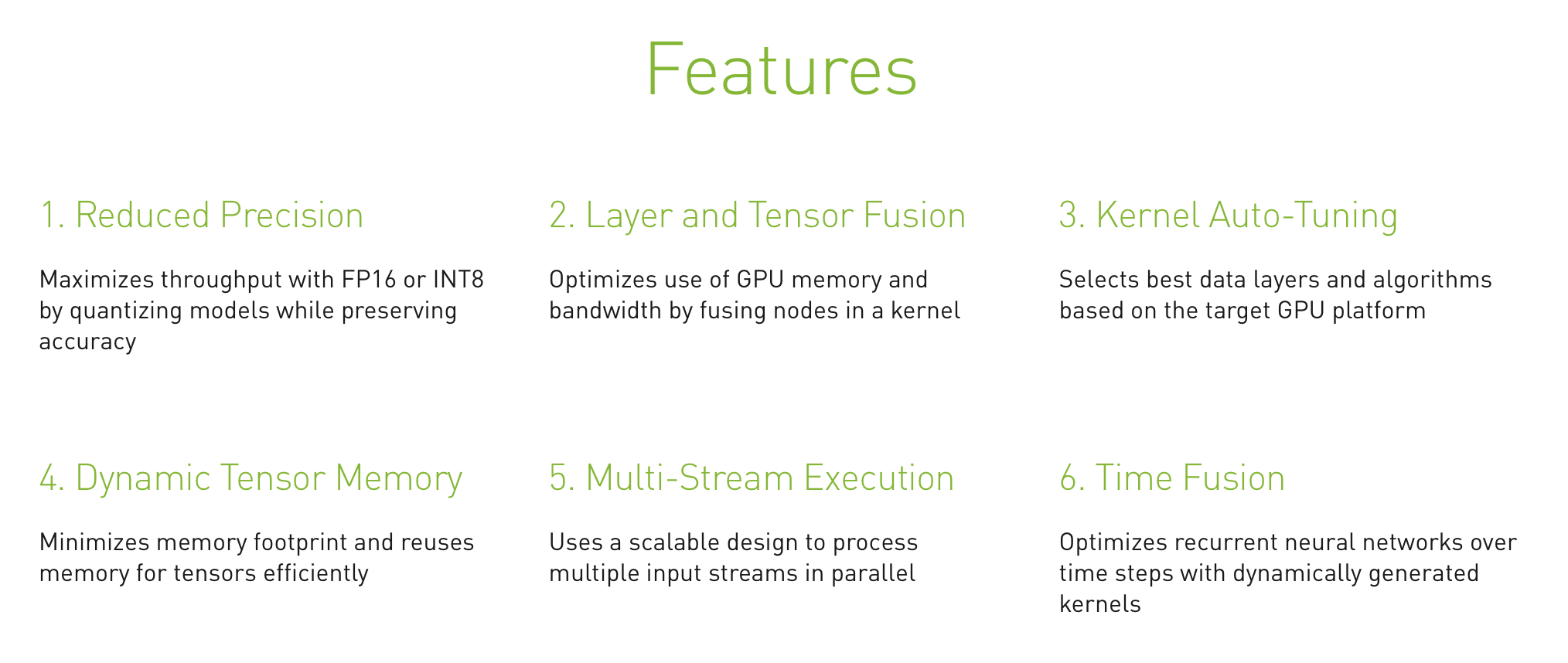TensorRT的特点 TensorRT可以将模型实现数倍的加速，官网声称一行代码可以实现对PyTorch加速6倍以上。 使用TensorRT部署PyTorch模型目前有两种方案，一是先转为ONNX再转为TensorRT，而是采用Torch-TensorRT直接优化。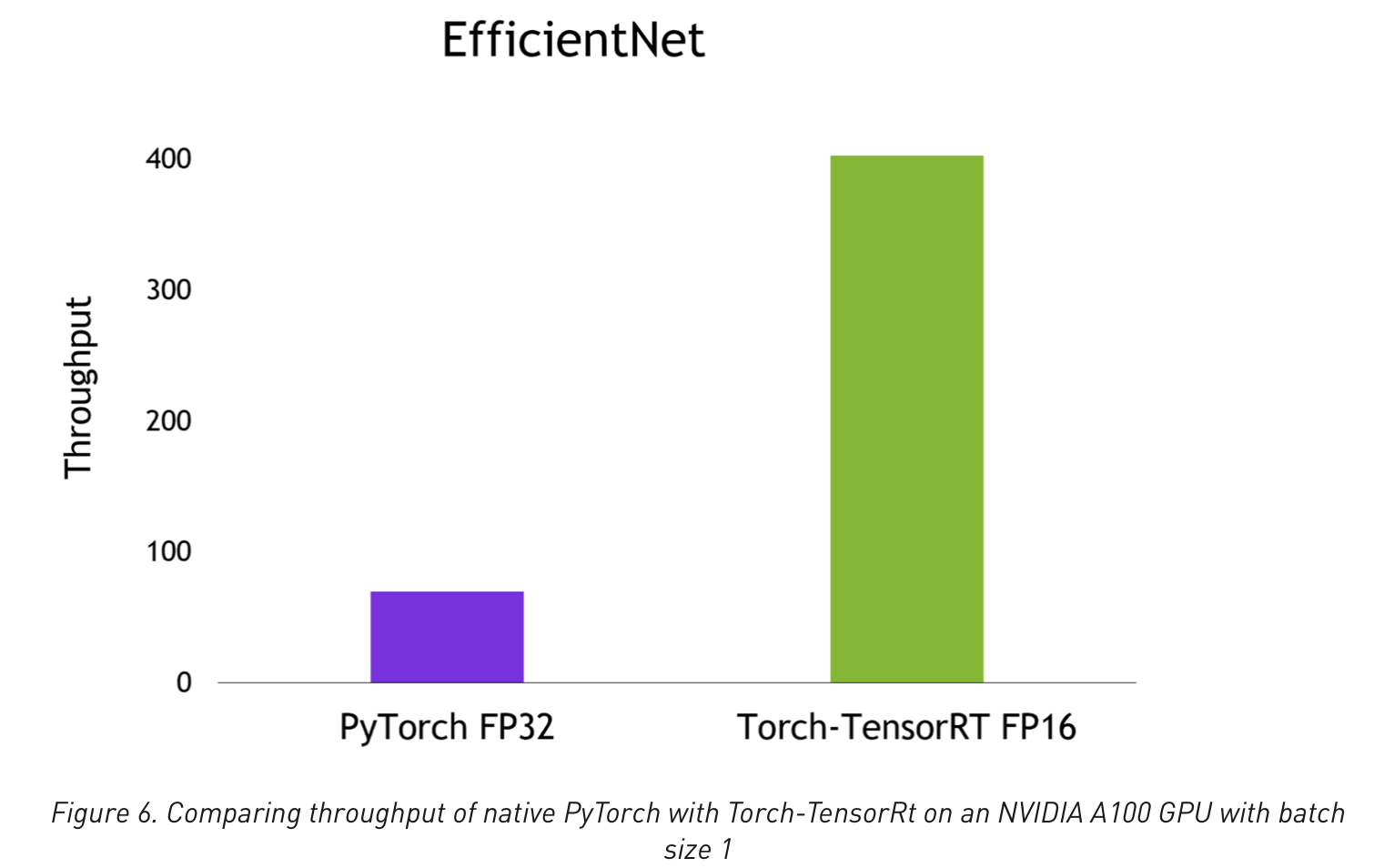前者相比之下生态更成熟（但还是有很多坑），而且ONNX也可以直接推理，因此采取先转ONNX再转TensorRT的方式。 ## 环境准备 平台为Linux，环境要求大致如下，尽量版本越新越好，新版本的兼容性往往更好，报错更少。 • CUDA 10.2 & CUDNN 8 • torch 1.11.0 • onnx 1.9.0 • onnxruntime 1.10.0 • TensorRT 8.2.3 友情提醒：安装教程一定要参考官网教程！！！网络上的回答很容易不正确或过时！！！ ### 安装CUDA和CUDNN 参考官方CUDA手册，去下载页面选择合适的CUDA版本下载即可，可以选择run包，下载后直接运行就可以安装。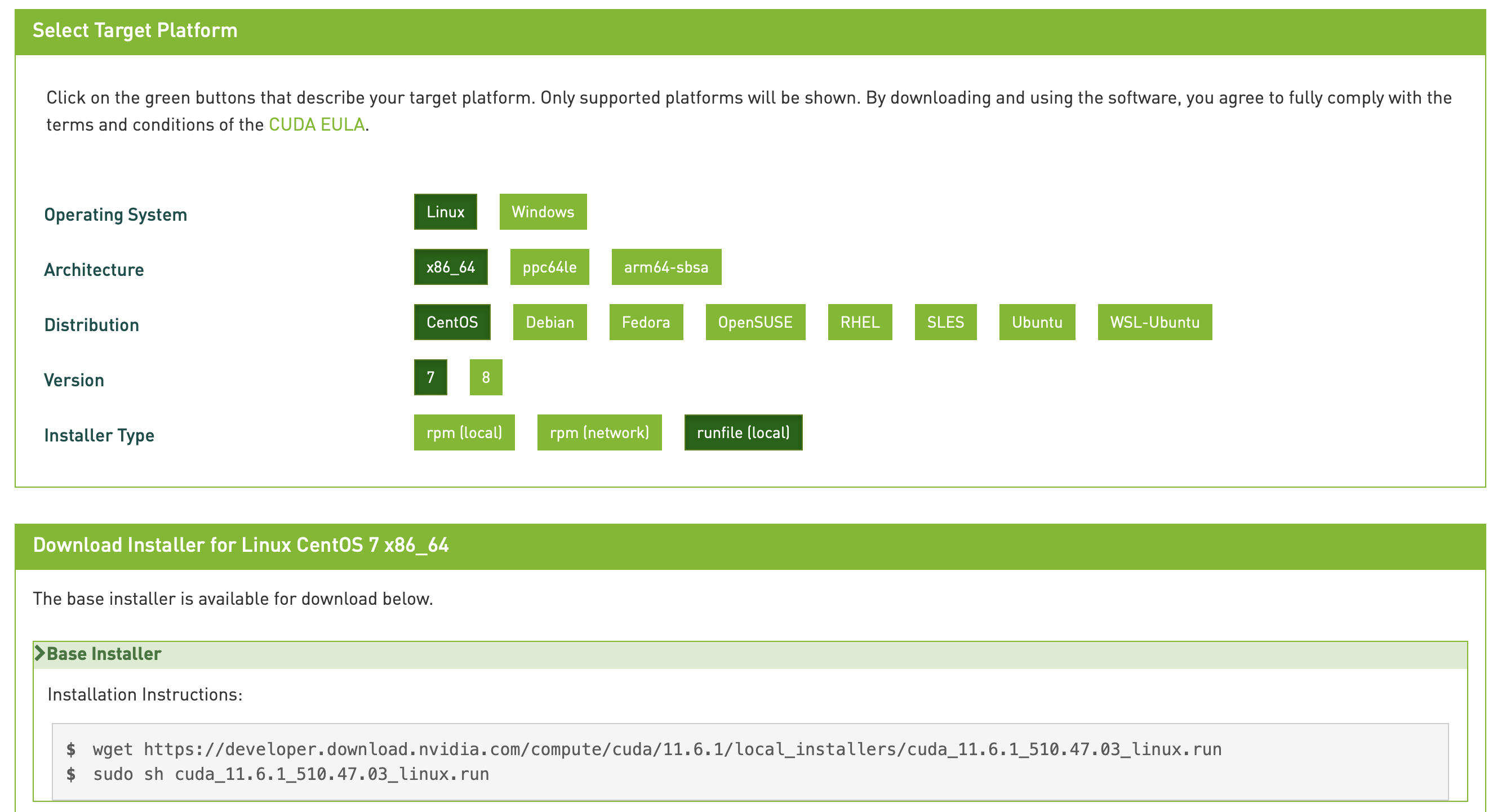如果之前安装过显卡驱动的（用nvidia-smi可以查看），在安装时可以取消驱动那一部分，只安装CUDA即可。 一般安装路径为/usr/local/cuda，这是一个软链接，指向某一个实际版本的CUDA（10.2或10.1等等），如果没有需要自己创建。 机器上存在多个CUDA版本时，也可以通过修改这个软链接来更换CUDA版本。 安装后，还需要修改环境变量，往往会写在~/.bashrc/etc/profile中，因人而异。 export PATH=/usr/local/cuda/bin:{PATH}export LD_LIBRARY_PATH=/usr/local/cuda/lib64:${LD_LIBRARY_PATH} 修改之后source ~/.bashrc生效，使用nvcc -V查看CUDA版本号，正常显示即安装成功。 至于CUDNN，依旧参考官方安装手册。去下载页面（需要登陆）下载对应版本的CUDNN即可，推荐越新越好（March 18th, 2022刚更新了v8.3.3），注意与CUDA版本匹配。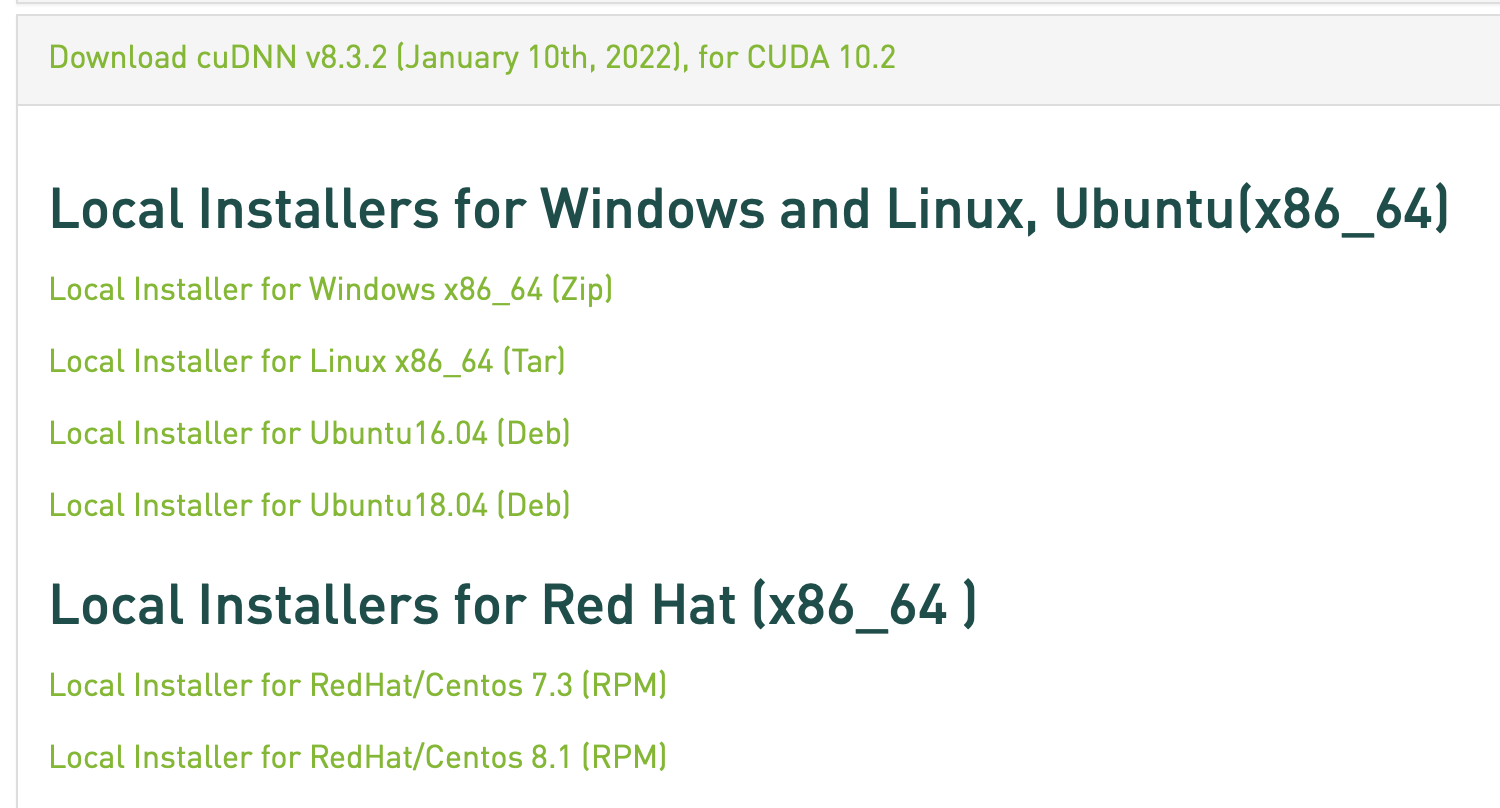下载tar压缩包后，解压复制即可安装成功。 tar -xvf cudnn-linux-x86_64-8.x.x.x_cudaX.Y-archive.tar.xzsudo cp cudnn-*-archive/include/cudnn*.h /usr/local/cuda/include sudo cp -P cudnn-*-archive/lib/libcudnn* /usr/local/cuda/lib64 sudo chmod a+r /usr/local/cuda/include/cudnn*.h /usr/local/cuda/lib64/libcudnn* ### 安装PyTorch和ONNX 直接pip install即可，TensorRT目前好像只支持到onnx==1.9.0 pip3 install onnx==1.9.0pip3 install onnxruntimepip3 install torch torchvision torchaudio 为了简化模型，还可以安装onnx-simplifier pip3 install onnx-simplifier ### 安装TensorRT 参考官方安装手册，去下载页面（需要登陆）下载tar包。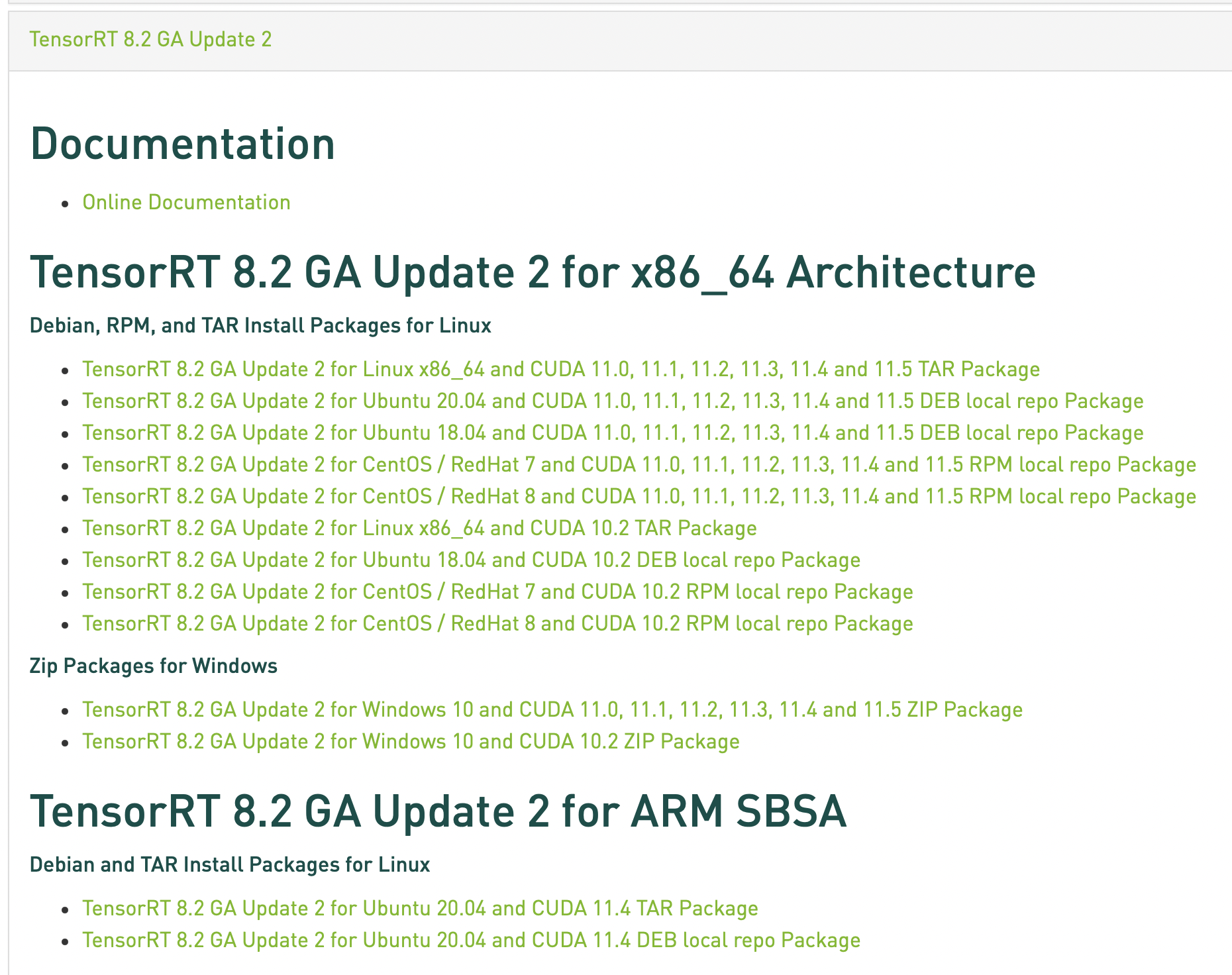解压后有若干子目录 ls TensorRT-${version}bin  data  doc  graphsurgeon  include  lib  onnx_graphsurgeon  python  samples  targets  TensorRT-Release-Notes.pdf  uff

cd TensorRT-${version}/pythonpython3 -m pip install tensorrt-*-cp3x-none-linux_x86_64.whlcd TensorRT-${version}/uffpython3 -m pip install uff-0.6.9-py2.py3-none-any.whlcd TensorRT-${version}/graphsurgeonpython3 -m pip install graphsurgeon-0.4.5-py2.py3-none-any.whlcd TensorRT-${version}/onnx_graphsurgeon    python3 -m pip install onnx_graphsurgeon-0.3.12-py2.py3-none-any.whl

export LD_LIBRARY_PATH=$LD_LIBRARY_PATH:<TensorRT-${version}/lib>

python3 -m pip install 'pycuda<2021.1'

## 导出ONNX模型

input_data = torch.randn(batch_size, *input_shape)model = self.model.to('cpu')model.eval()torch.onnx.export(model,                  input_data,                  onnx_file_path,                  opset_version=12,                  input_names=input_name,                  output_names=output_name,                  verbose=True)print(f'save onnx model at {onnx_file_path}')

def save_model(self, dst_path, need_simplify=True):    model = self.onnx_model    if need_simplify:        model, check = simplify(model)        assert check, "Simplified ONNX model could not be validated"    onnx.save_model(model, dst_path)

TODO

## Reference

]]>
<p>记录一下用TensorRT部署一个基于Swin-Transformer的Pytorch模型的步骤，以作备忘。</p>
Git操作手册 https://entropy2333.github.io/2022/03/05/Git%E6%93%8D%E4%BD%9C%E6%89%8B%E5%86%8C/ 2022-03-05T15:01:44.000Z 2022-05-13T14:16:42.811Z Git常见操作备忘

## 配置

# 查看git config user.namegit config user.email# 修改（局部）git config user.name ${your_name}git config user.email${email@example.com}# 修改（全局）git config --global user.name ${your_name}git config --global user.email${email@example.com}

git remote add origin https://<access-token-name>:<access-token>@gitlab.com/myuser/myrepo.git

### 创建分支并提交

# 添加远程分支git remote add origin git@git.sjtu.edu.cn/user/repo.git# 创建本地分支git checkout -b local_branch# 创建空分支git checkout --orphan local_branch# push到远程分支git push origin local_branch

### git-lfs

# 安装brew install git-lfs# 开启lfs功能git lfs install# 追踪大文件，提交时需要添加.gitattributesgit lfs track# 追踪指定文件git lfs track *.pdf# 查看追踪的文件列表git lfs ls-files

### 删除远程仓库不存在的分支

# 查看所有分支git branch -a# 只查看远程分支git branch -r# -p/--prune: 删除不存在的远程分支git fetch -p

# 合并最近的n次提交git rebase -i HEAD~n# 合并当前HEAD到指定commit idgit rebase $commit-id 默认编辑器为nano，可以用git config --global core.editor "vim"修改为vim。 ## 修改历史提交信息 git filter-branch -f --env-filter 'OLD_EMAIL="old_email"CORRECT_NAME="your_name"CORRECT_EMAIL="email@exmaple.com"if [ "$GIT_COMMITTER_EMAIL" = "$OLD_EMAIL" ]then export GIT_COMMITTER_NAME="$CORRECT_NAME"    export GIT_COMMITTER_EMAIL="$CORRECT_EMAIL"fiif [ "$GIT_AUTHOR_EMAIL" = "$OLD_EMAIL" ]then export GIT_AUTHOR_NAME="$CORRECT_NAME"    export GIT_AUTHOR_EMAIL="\$CORRECT_EMAIL"fi' --tag-name-filter cat -- --branches --tags
]]>
<p>Git常见操作备忘</p>
Combining Label Propagation and Simple Models Out-performs Graph Neural Networks https://entropy2333.github.io/2022/02/24/Combining-Label-Propagation-and-Simple-Models-Out-performs-Graph-Neural-Networks/ 2022-02-24T08:11:08.000Z 2022-03-03T08:03:38.900Z ICLR 2021，采用后处理的方法而非GNN在OGB上取得SOTA。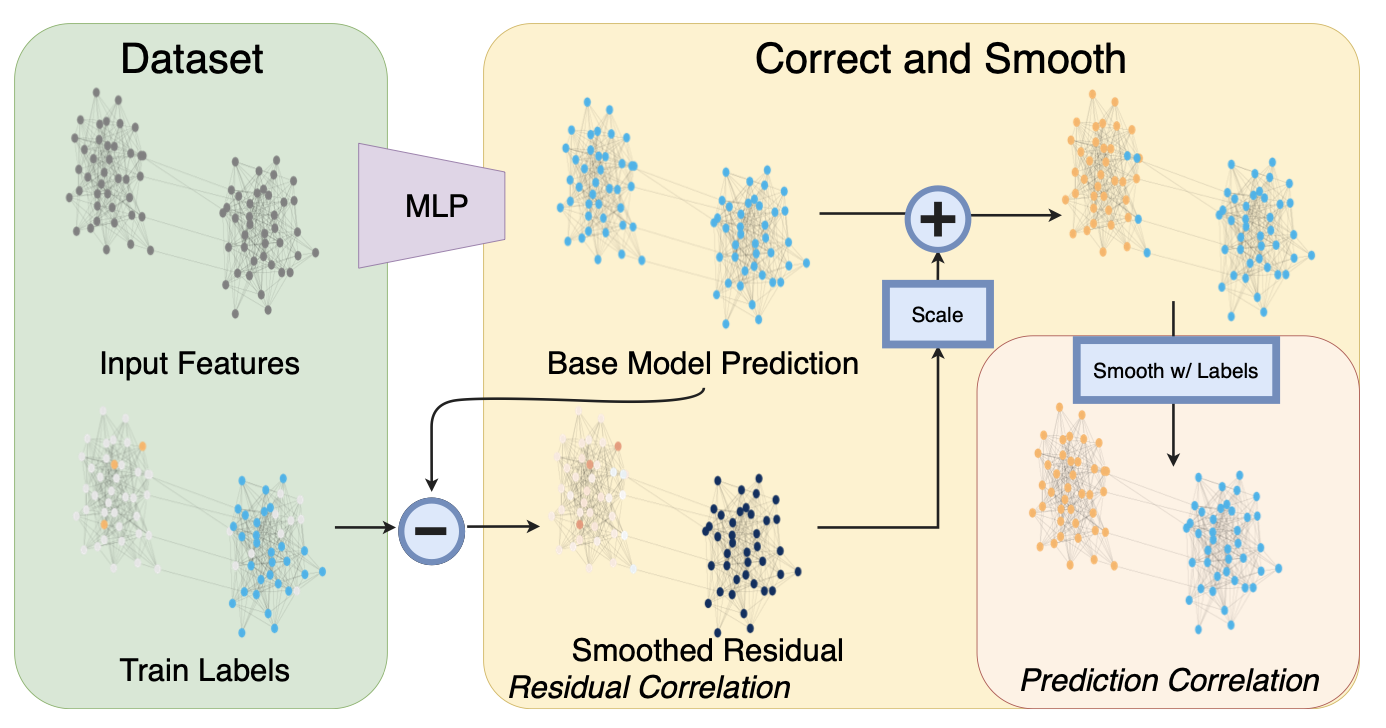## Overview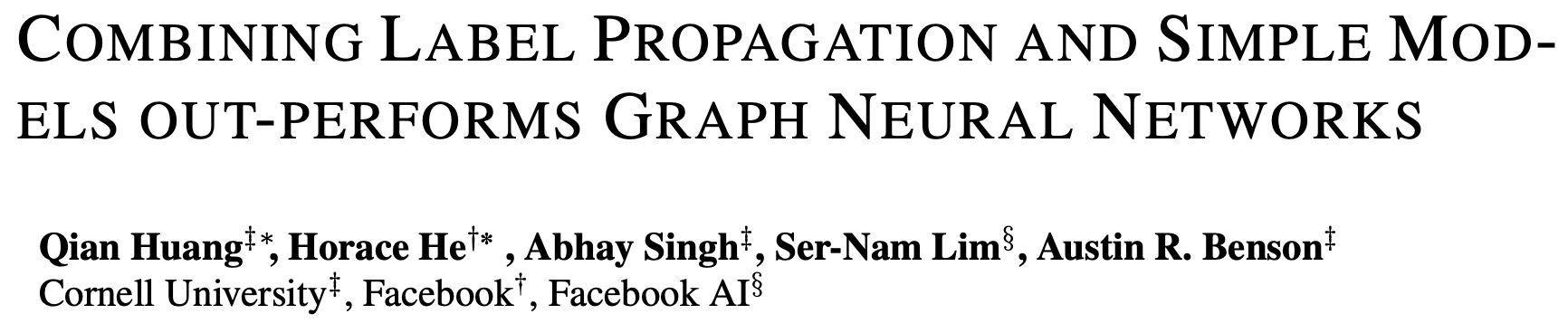## Background

1. 忽略图结构，用节点特征进行基本预测。
2. 纠正步骤，将训练数据的不确定性传播到整个图中，以纠正基本预测。
3. 对预测结果进行平滑处理。

## Method

$$G=(V,E)$$$$n=|V|$$个节点，节点特征$$X\in\mathbb{R}^{n\times p}$$$$A$$为邻接矩阵，$$D$$为对角度矩阵，$$S=D^{-1/2}AD^{-1/2}$$。对于预测问题，节点集合分为有标注的$$L$$和无标注的$$U$$，标签$$Y\in\mathbb{R}^{n\times c}$$，需要给未标注的节点$$j\in U$$打标签。

## Experiment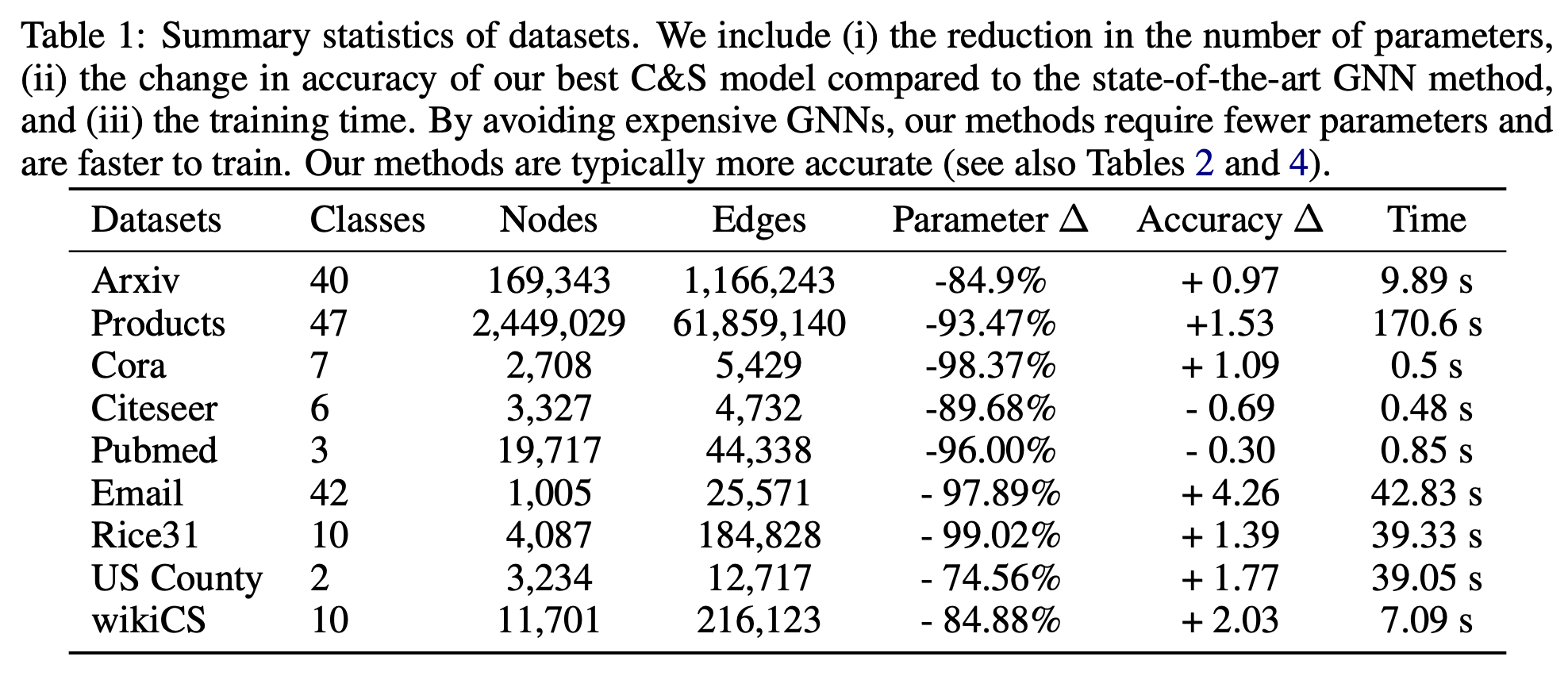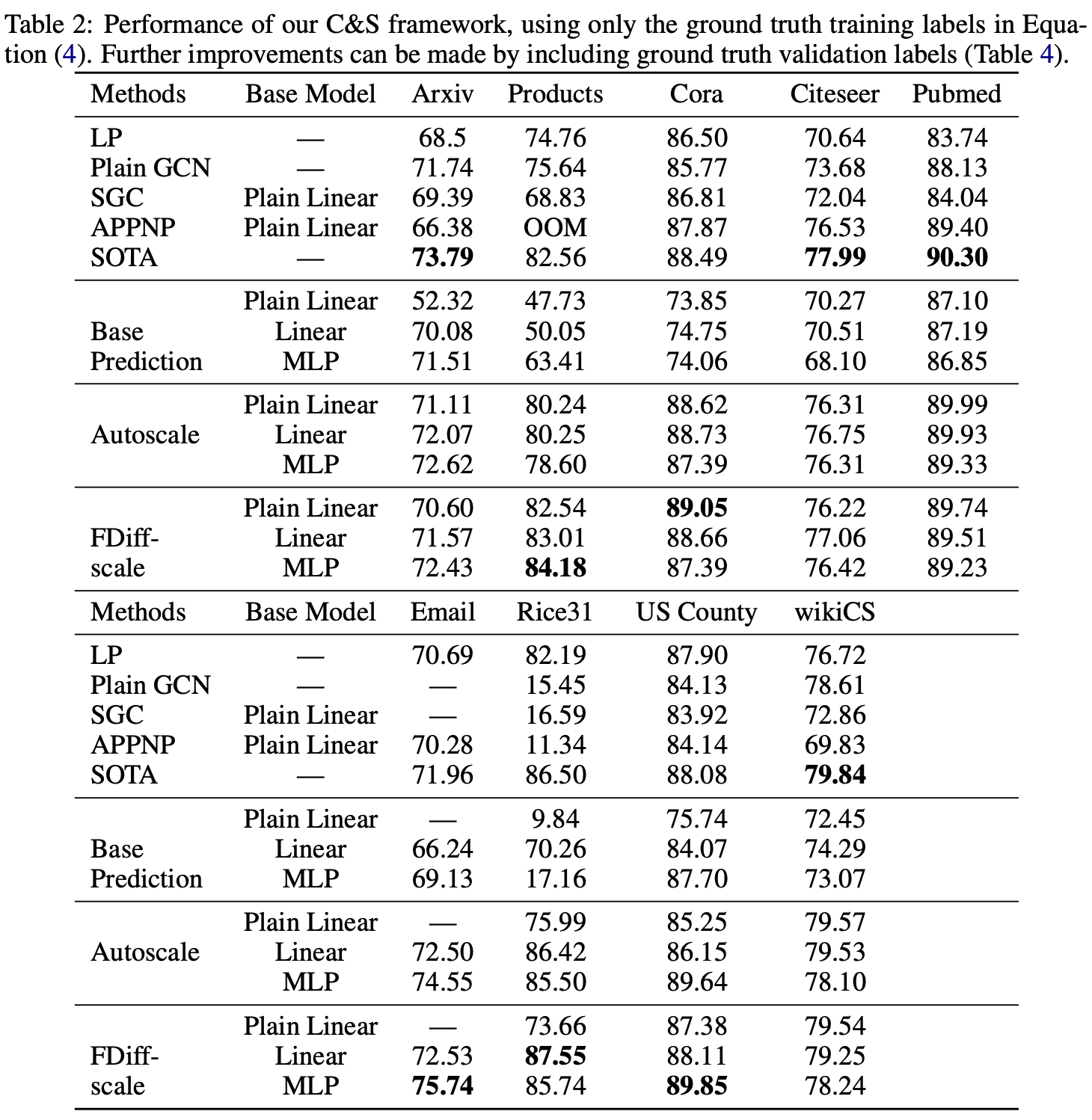]]>
<p>ICLR 2021，采用后处理的方法而非GNN在OGB上取得SOTA。</p> <p><img src="/2022/02/24/Combining-Label-Propagation-and-Simple-Models-Out-performs-Graph-Neural-Networks/image-20220224170455627.png" alt="image-20220224170455627" style="zoom: 50%;"></p>
Laplace Redux -- Effortless Bayesian Deep Learning https://entropy2333.github.io/2022/02/21/Laplace-Redux-Effortless-Bayesian-Deep-Learning/ 2022-02-21T07:04:11.000Z 2022-02-22T03:14:42.934Z NIPS 2021，拉普拉斯近似（Laplacian Approximation）。

## Overview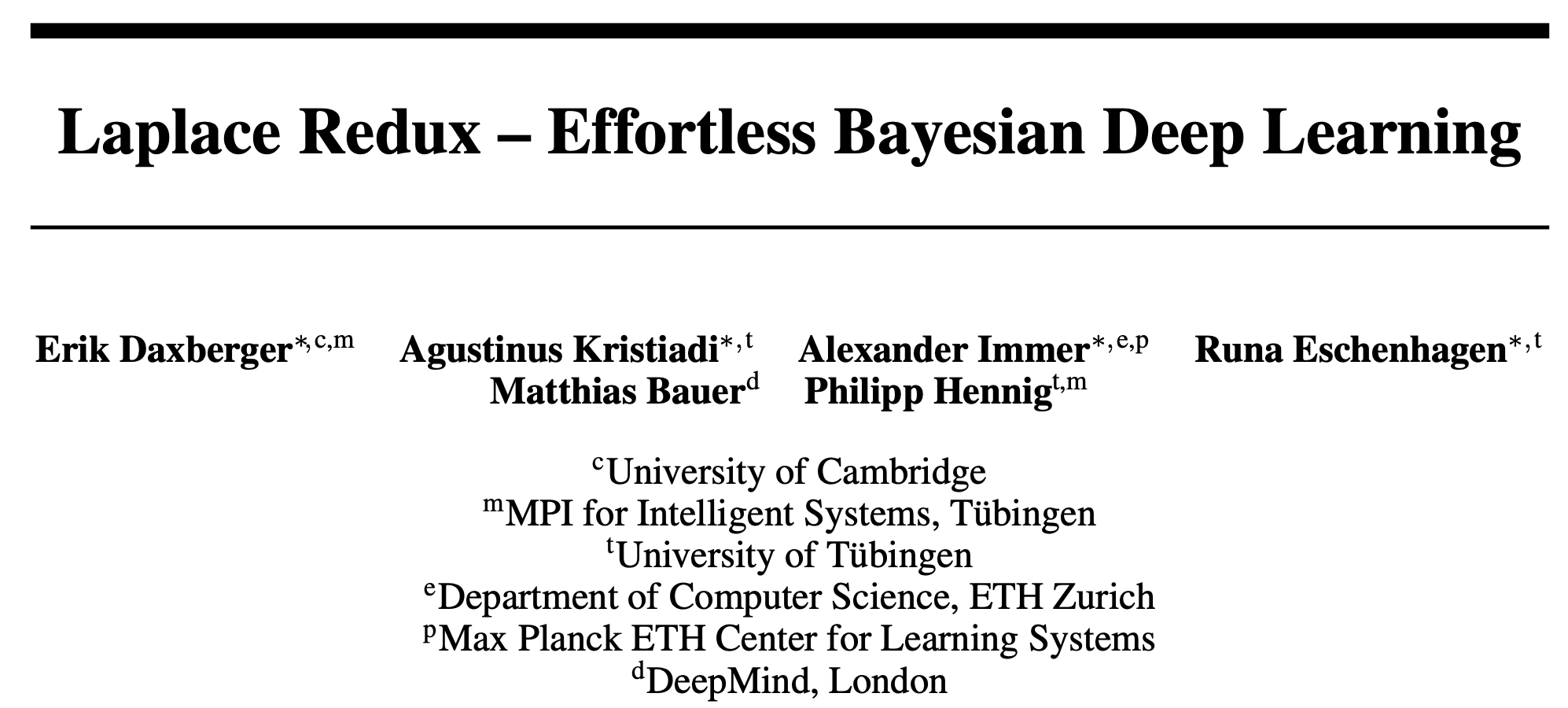## Background

• 校准能力差，过度自信（overconfidence）。
• 持续训练时对先前任务的灾难性遗忘（catastrophic forgetting）。
• 选择合适的网络架构和超参数很困难。

• 给模型配备鲁棒的不确定估计。
• 捕获过去的信息，使得模型能够持续学习。

• 本文率先调研了深度学习中LA的发展，并展现了可扩展且实用的LA的关键要素。
• 提供了基于Pytorch的LA库laplace，实现了大量LA变种。
• 使用laplace库进行了大量实验，展现了LA的竞争力。

## Method

### Laplace Approximation in Deep Learning

1. 采用LA近似模型的后验分布，使得模型能够概率预测。
2. 使用LA近似model evidence，从而可以模型选择（调超参数）。

### Four Components of Scalable Laplace Approximation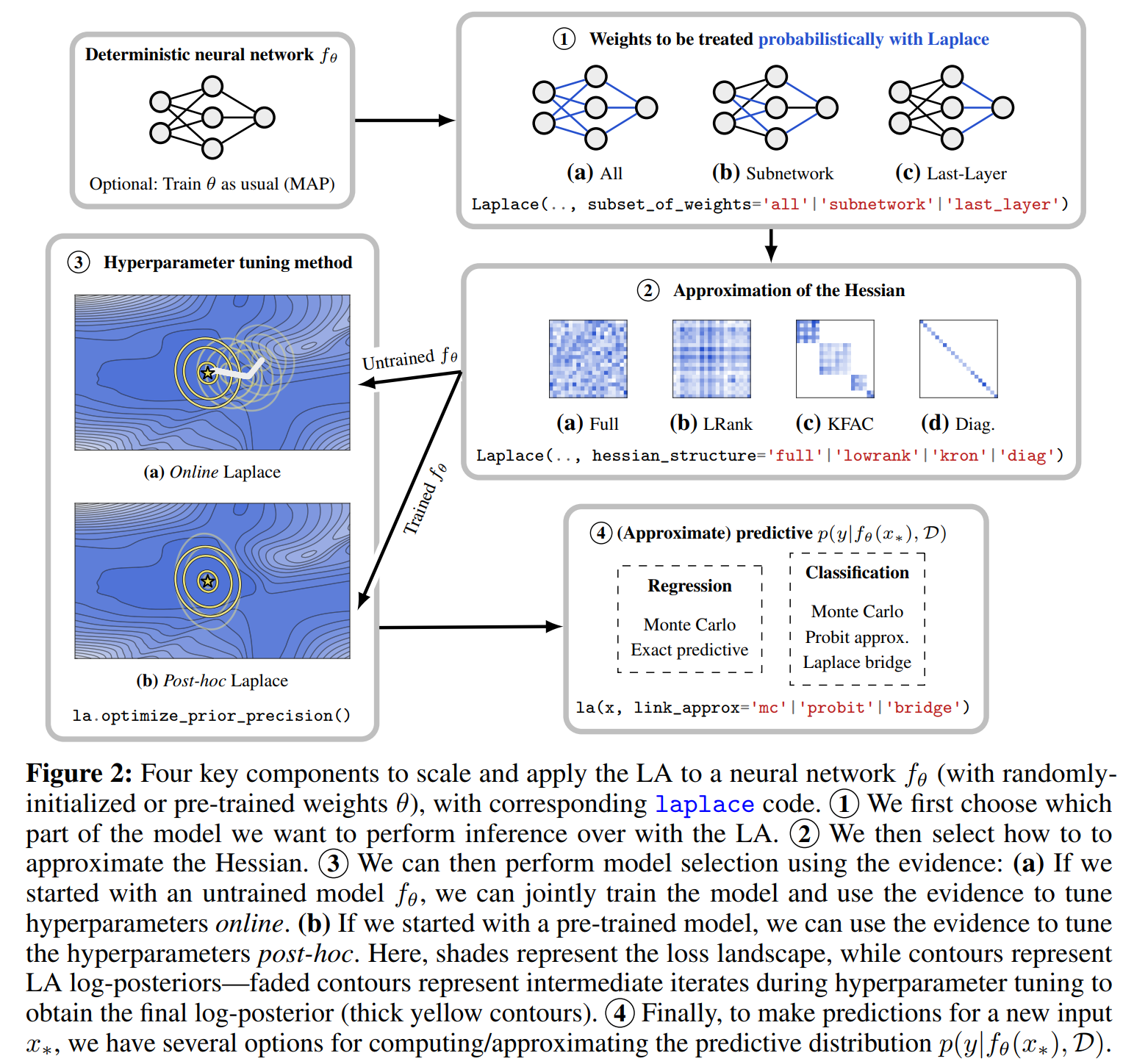### laplace: A Toolkit for Deep Laplace Approximation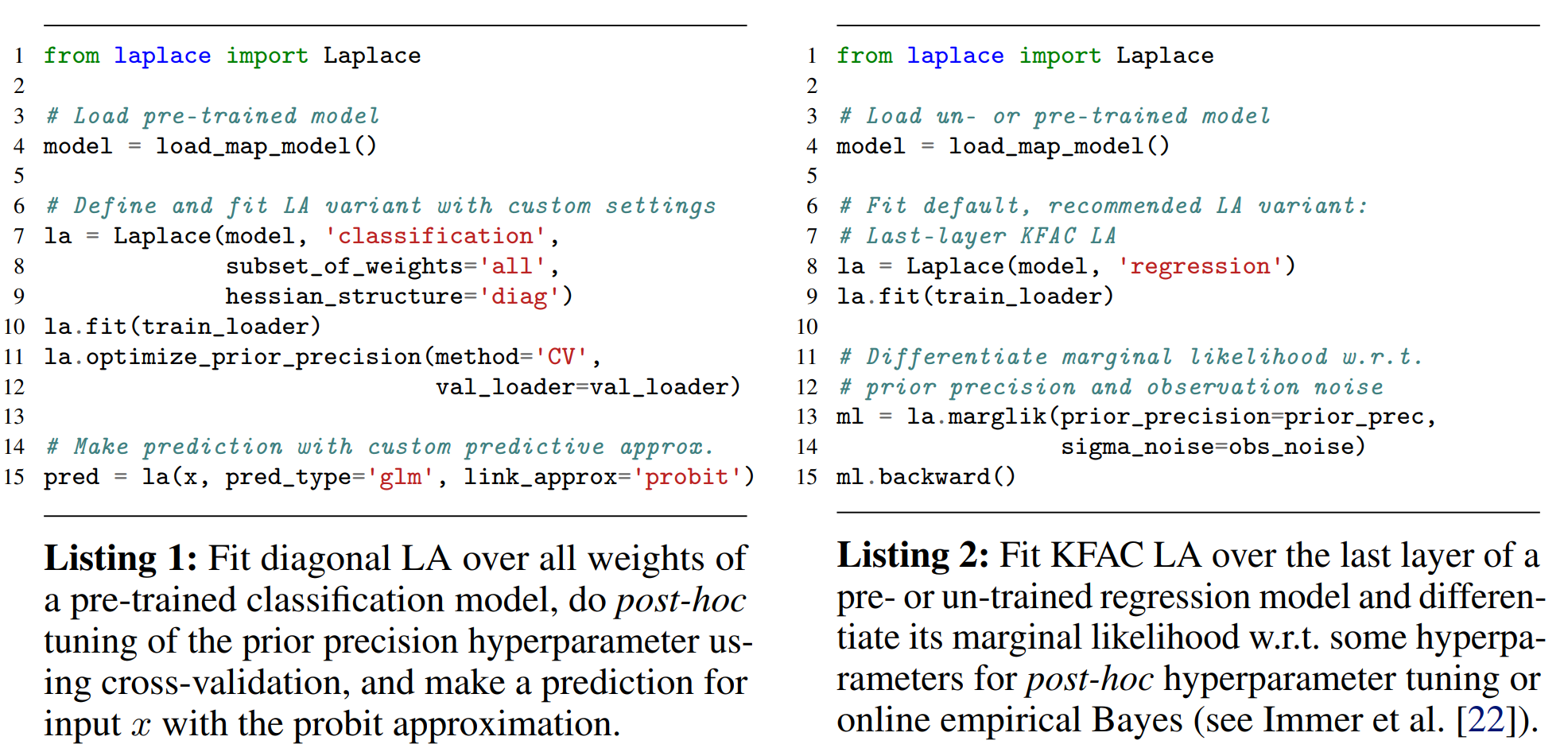## Experiments

1. 寻找LA的最佳设计选择。
2. 在in-distribution、dataset-shift和out-of-distribution三种设置下，验证LA相比于贝叶斯baseline的竞争力。
3. 展现LA适用于各种数据模态和网络架构（包括transformer），这种情况下贝叶斯方法难以使用。
4. 展示LA可以作为持续学习的一个易用baseline。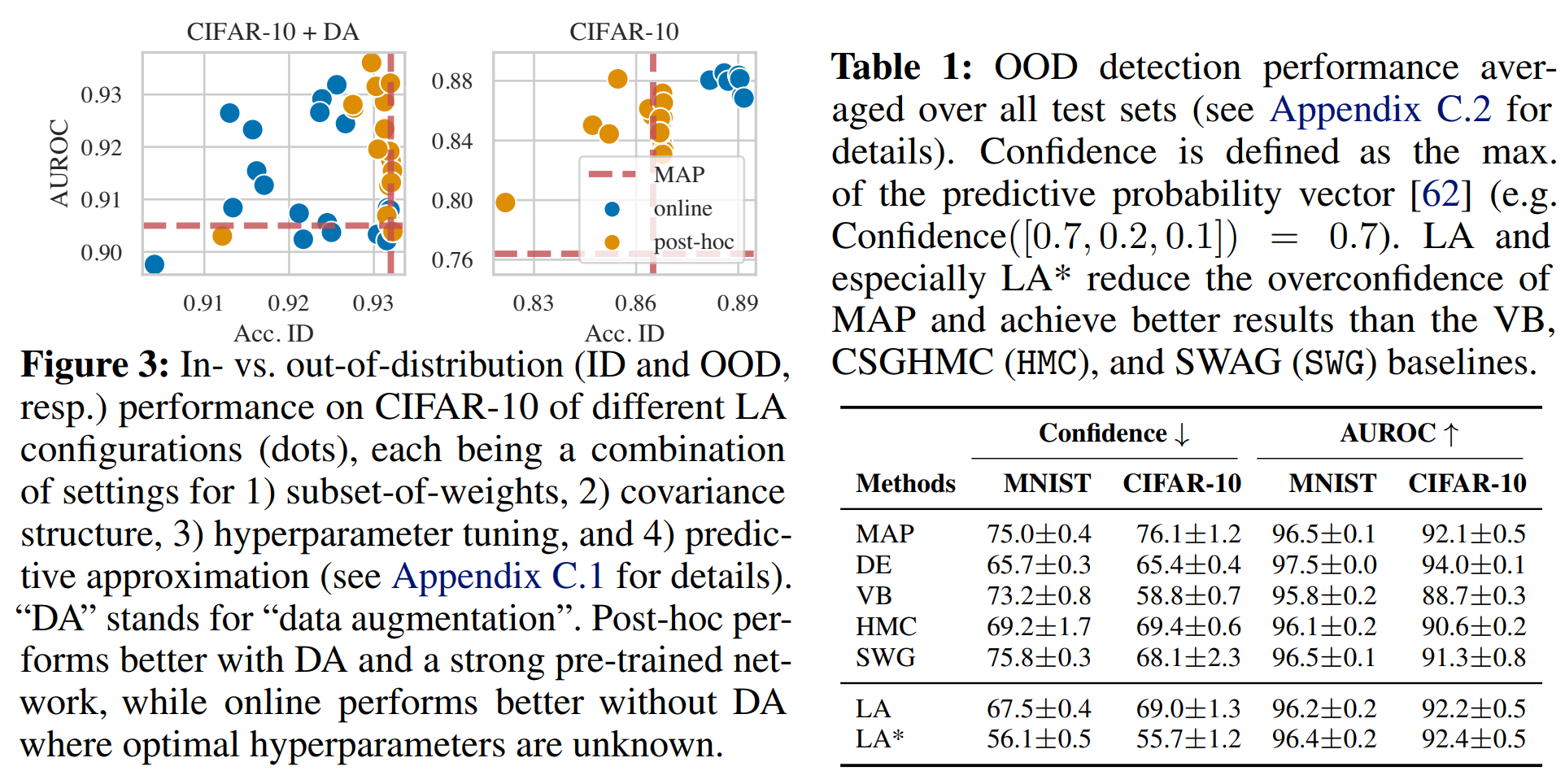laplace中默认设置是一个后处理KFAC最后一层的LA，采用GGN近似Hessian矩阵。这适用于所有最后一层为全连接层的网络架构，因此适用于预训练网络。对于需要自己训练的模型，采用GGN或经验Fisher的在线KFAC LA是一个不错的baseline。

]]>
<p>NIPS 2021，拉普拉斯近似（Laplacian Approximation）。</p>
RealFormer: Transformer Likes Residual Attention https://entropy2333.github.io/2022/02/20/RealFormer-Transformer-Likes-Residual-Attention/ 2022-02-20T05:46:06.000Z 2022-02-23T05:05:23.094Z ACL Findings 2021，在Transformer中引入残差Attention。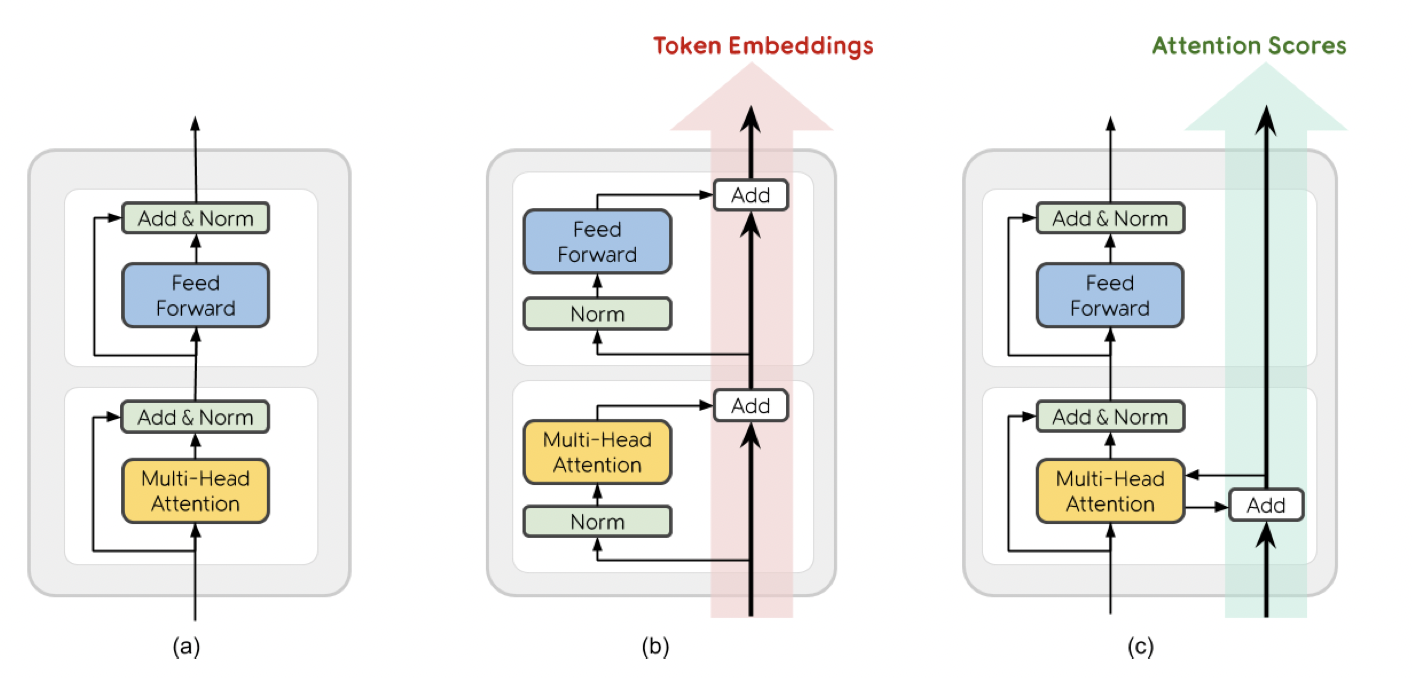## Overview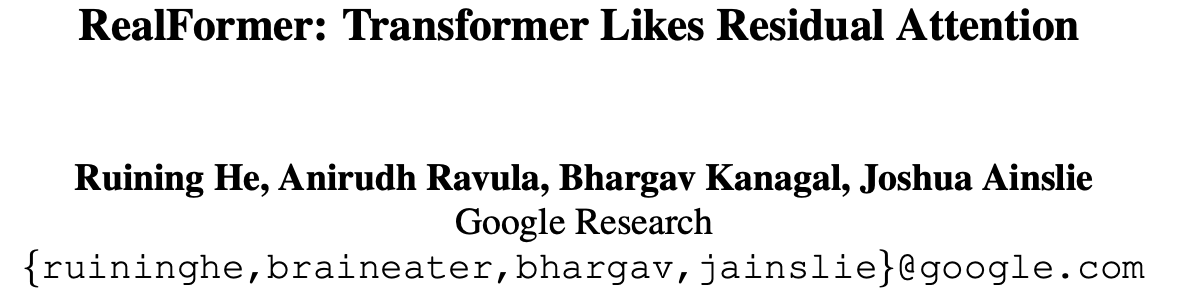## Background

Transformer按Layer Normalization的位置可以分为Post-LN和Pre-LN，BERT、XLNET、RoBERTa和ALBERT等都属于前者，GPT-2和Megatron属于后者。本文兼顾二者，引入残差注意力模块提升了Transformer的性能。

## Experiment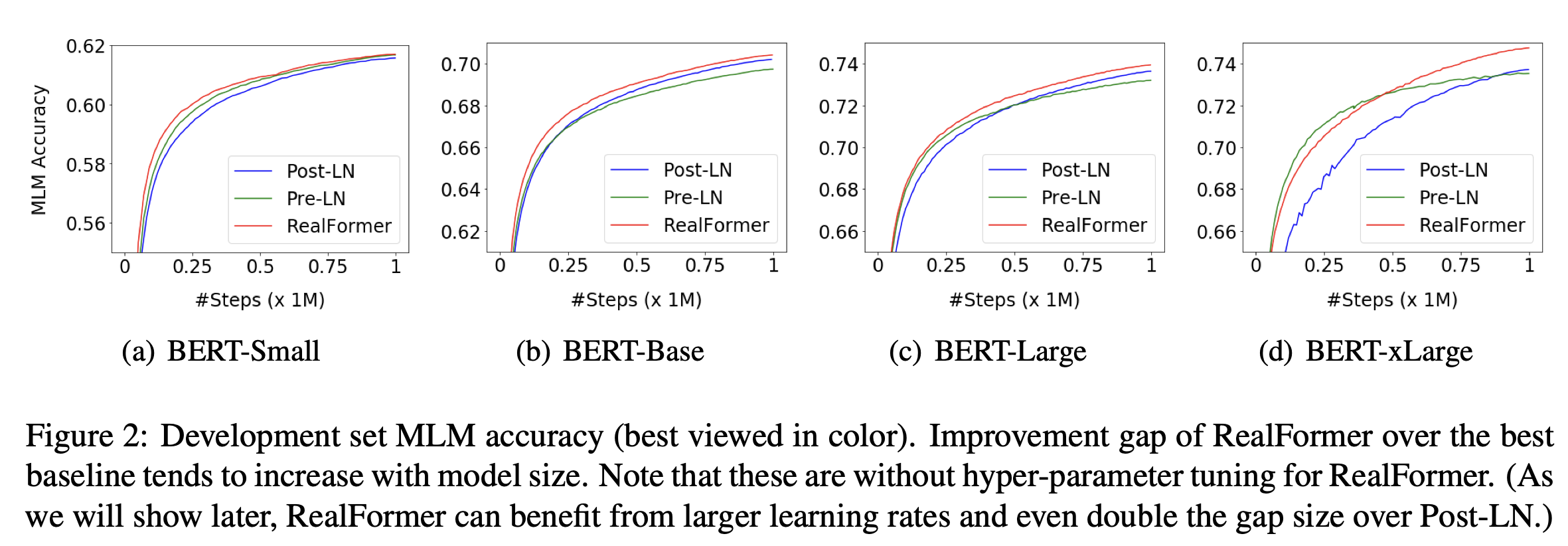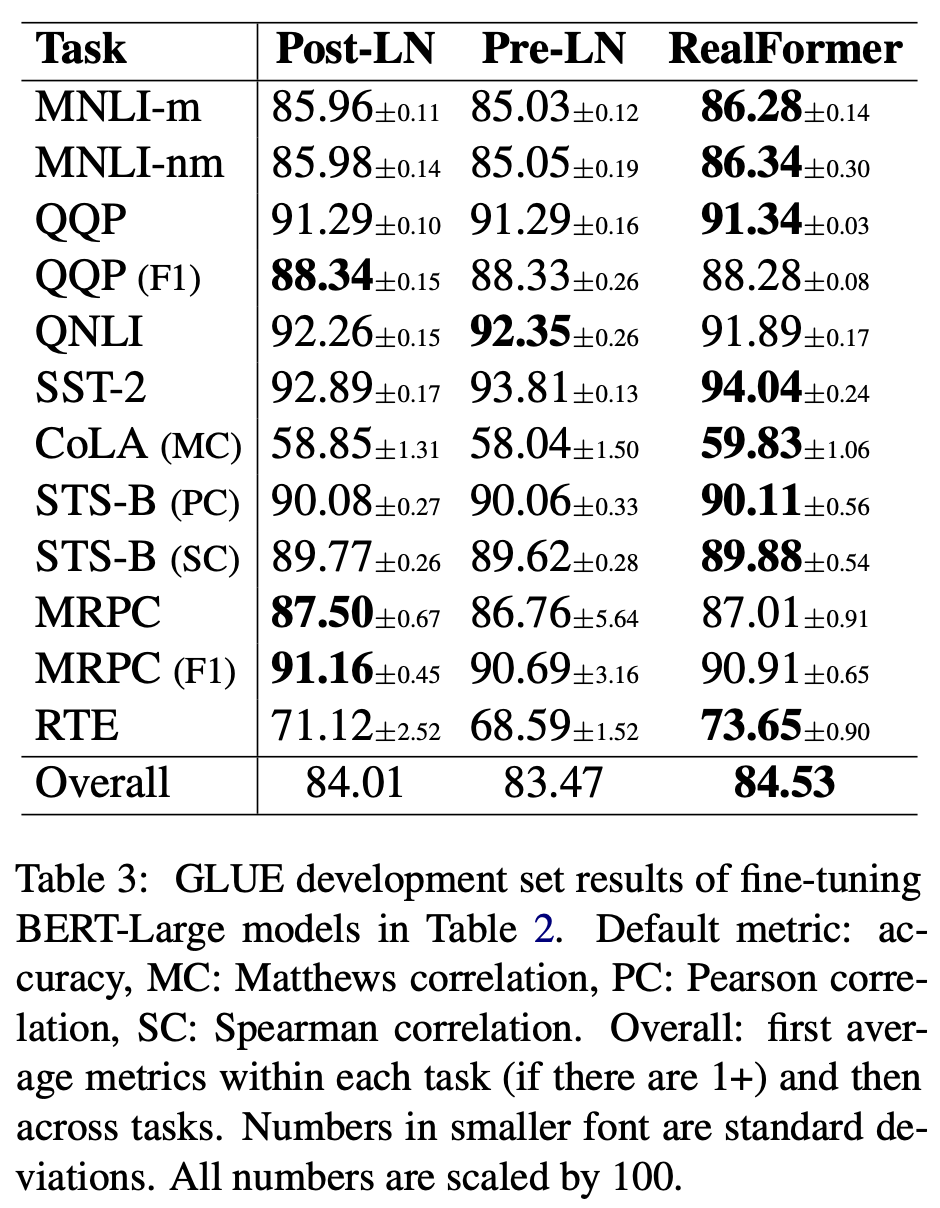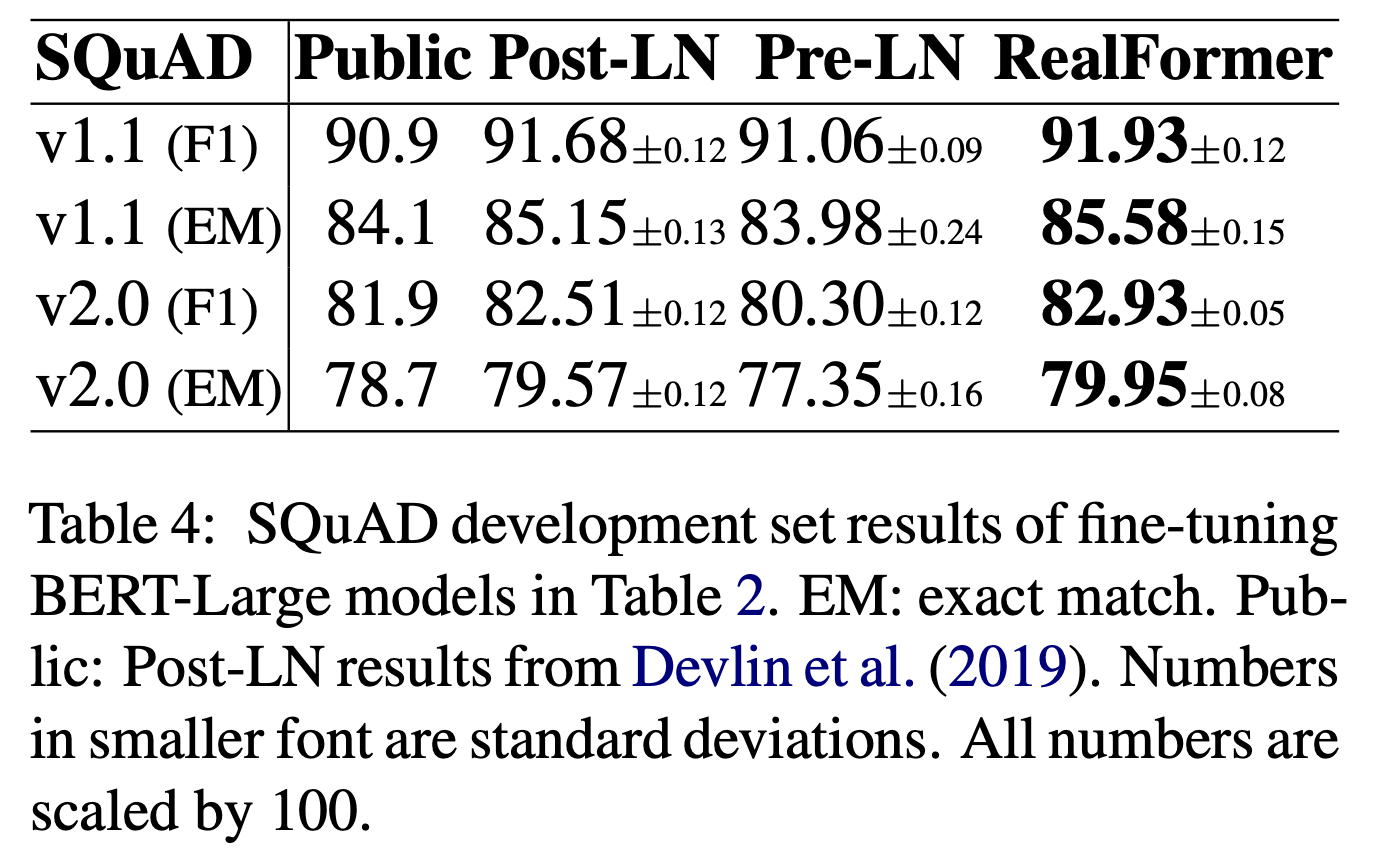]]>
<p>ACL Findings 2021，在Transformer中引入残差Attention。</p> <p><img src="/2022/02/20/RealFormer-Transformer-Likes-Residual-Attention/image-20220223125711993.png" alt="image-20220223125711993" style="zoom: 50%;"></p>
Multi-label Classification with Partial Annotations using Class-aware Selective Loss https://entropy2333.github.io/2022/02/18/Multi-label-Classification-with-Partial-Annotations-using-Class-aware-Selective-Loss/ 2022-02-18T05:57:22.000Z 2022-02-18T07:35:29.484Z 分析了多标签分类中的部分标注问题，设计了Loss函数处理这个问题。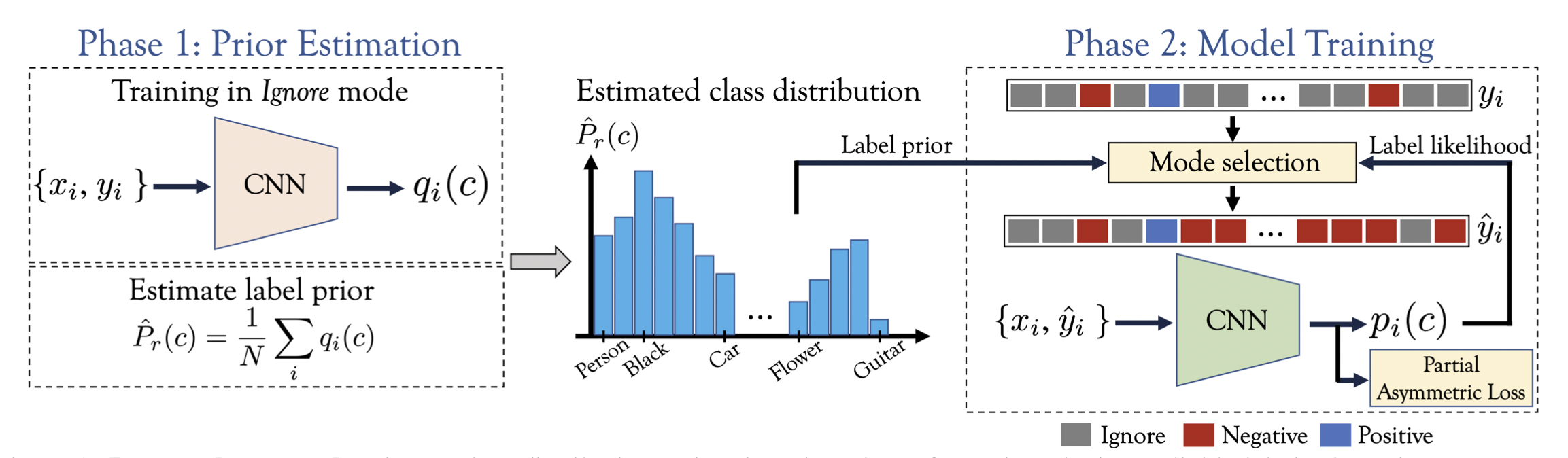## Overview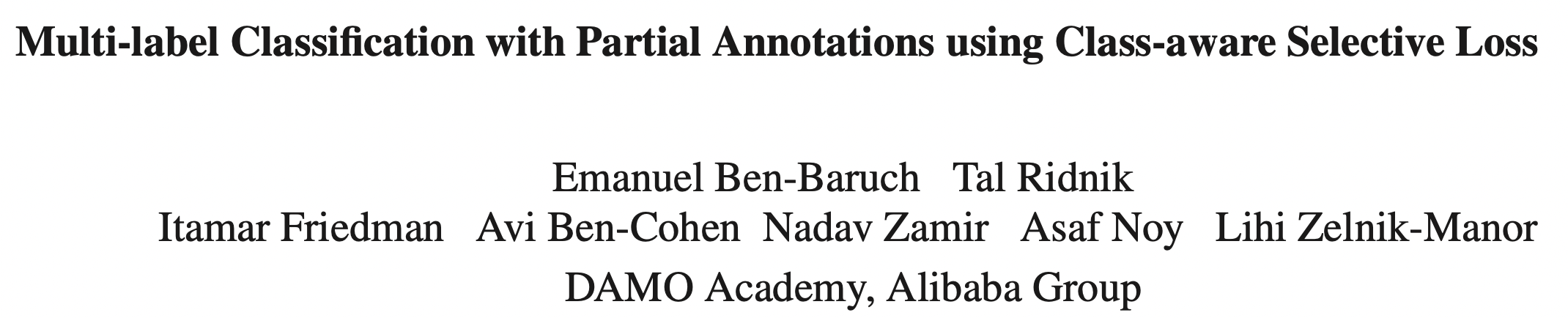## BackgroundOpenImages中“黑色”标签只标注了1688个样本，占全体样本的0.02%，然而这是很可能频繁出现的标签。因此统计数据集中类别的频数往往不能反映真实的标签比例，需要从数据中估计类别的分布。

## Method

### Estimating the Class Distribution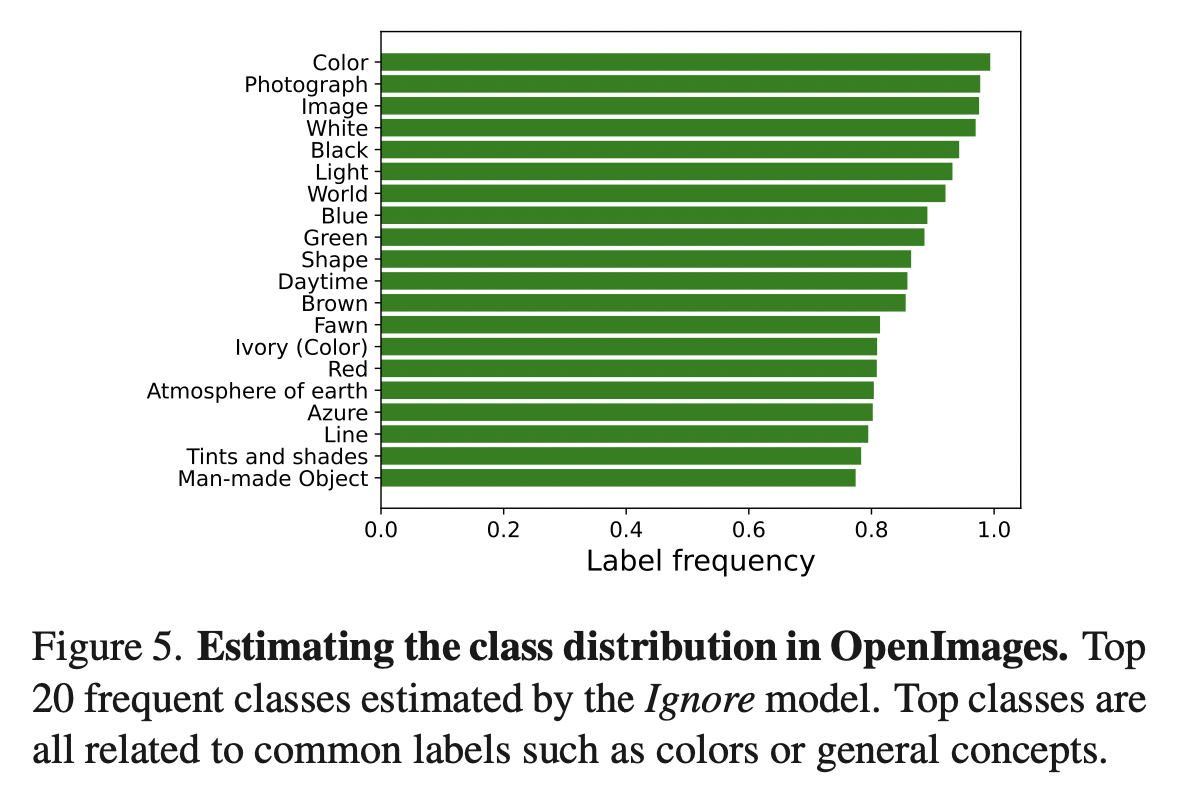## Experiment

1. Fixed per class (FPC) 对于每个类别随机采样固定数目$$N_s$$的正负标注，丢弃其余标注。
2. Random per annotation (RPA) 按概率$$p$$删除每个标注。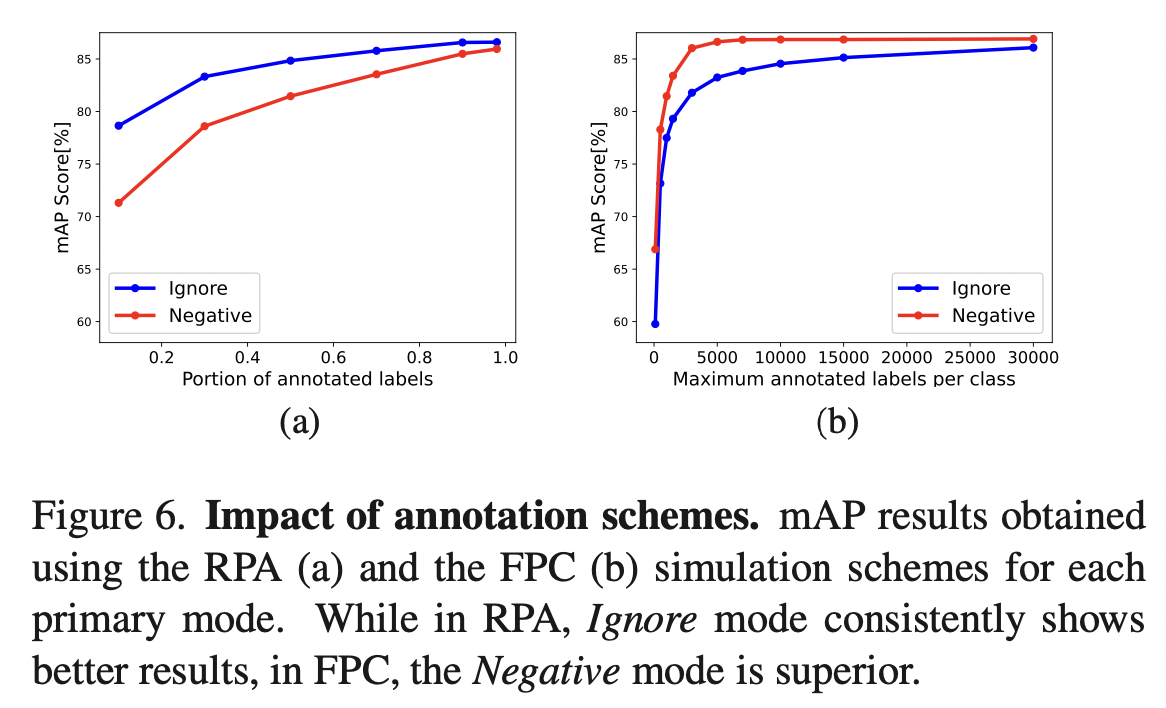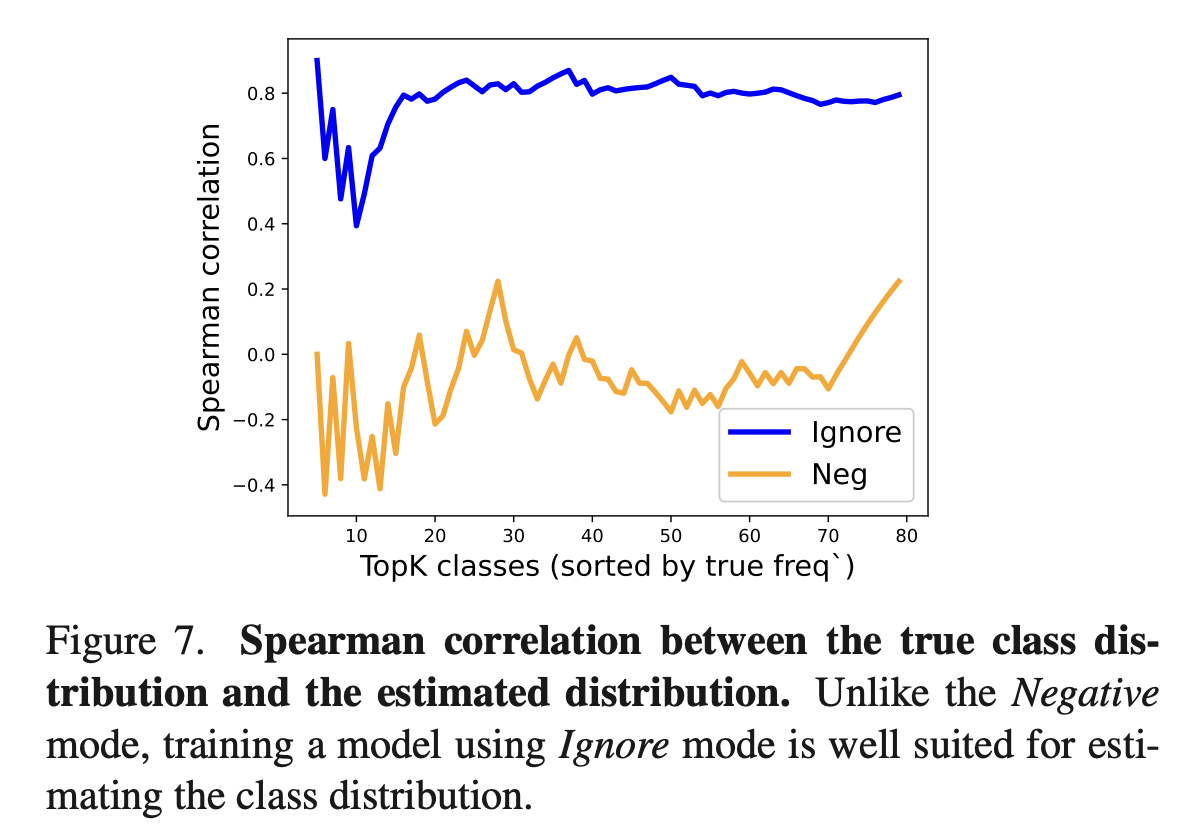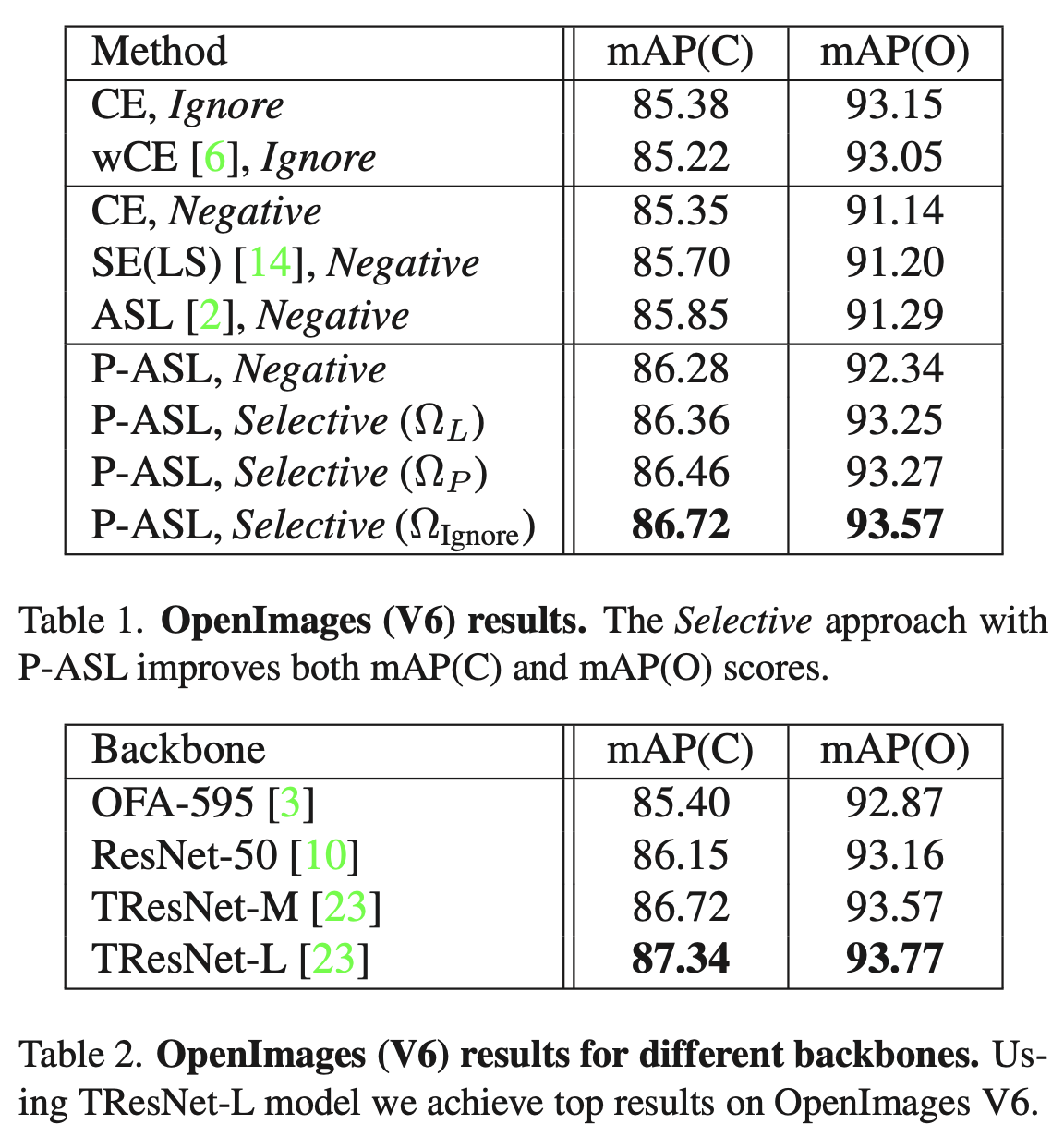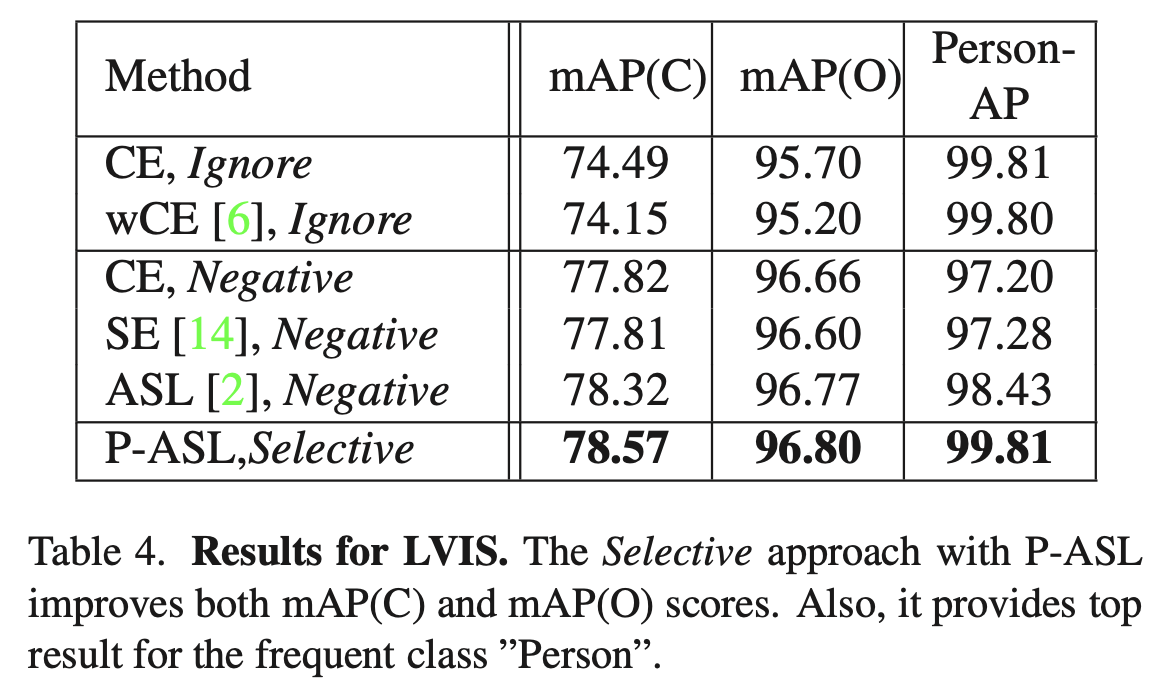]]>
<p>分析了多标签分类中的部分标注问题，设计了Loss函数处理这个问题。</p> <p><img src="/2022/02/18/Multi-label-Classification-with-Partial-Annotations-using-Class-aware-Selective-Loss/image-20220218142826882.png" alt="image-20220218142826882" style="zoom:33%;"></p>
HCL-MTC: Hierarchical Contrastive Learning for Multi-label Text Classification https://entropy2333.github.io/2022/02/13/HCL-MTC-Hierarchical-Contrastive-Learning-for-Multi-label-Text-Classification/ 2022-02-13T10:50:01.000Z 2022-02-18T06:21:35.845Z ACL ARR 2022，提出层次对比学习，学习标签间的区别信息。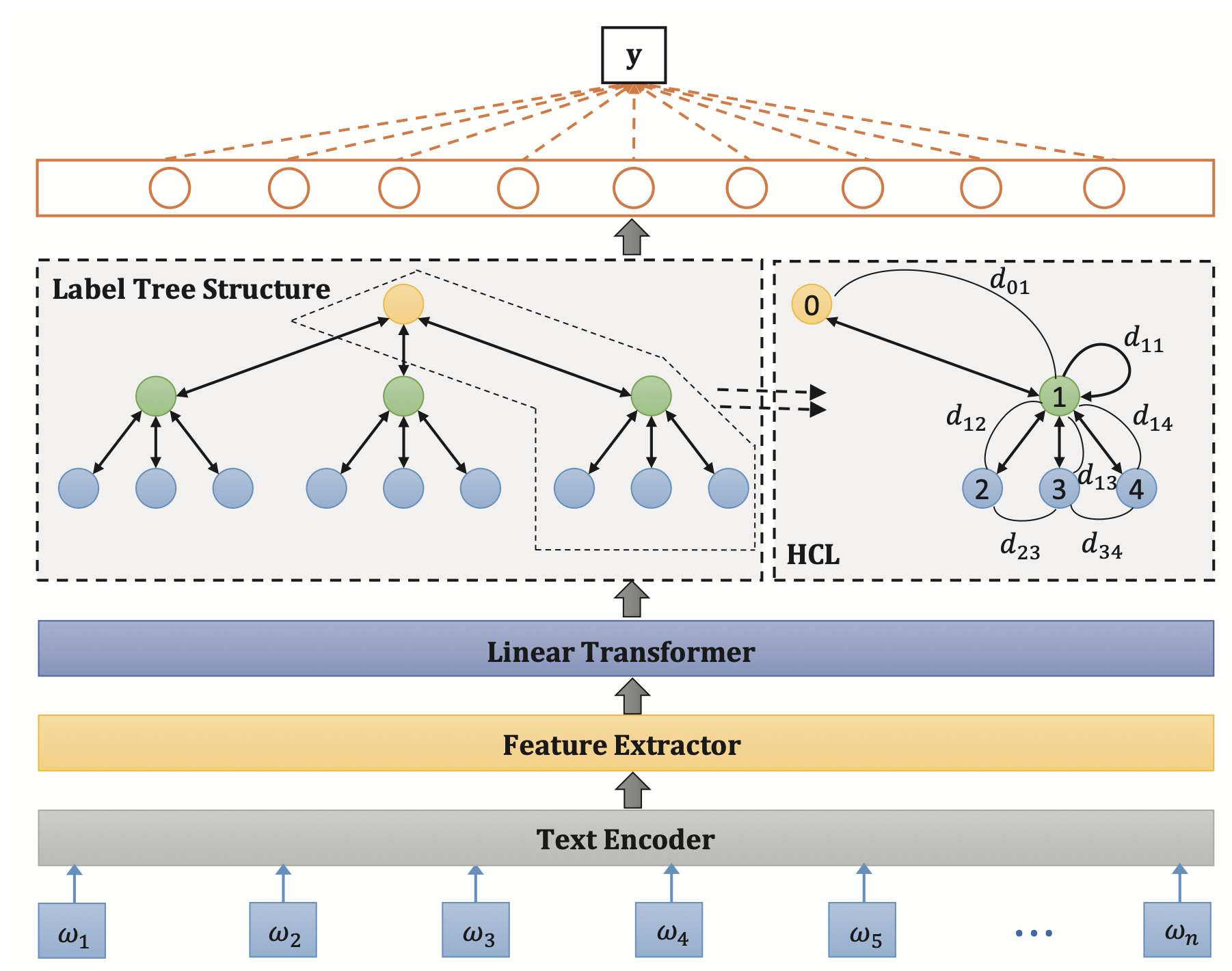## Background

MLTC任务可以分为两种方法：直接从文本信息预测以及从文本标签的混合信息中预测。前者忽略了标签间的信息，后者可以学习标签的层次信息。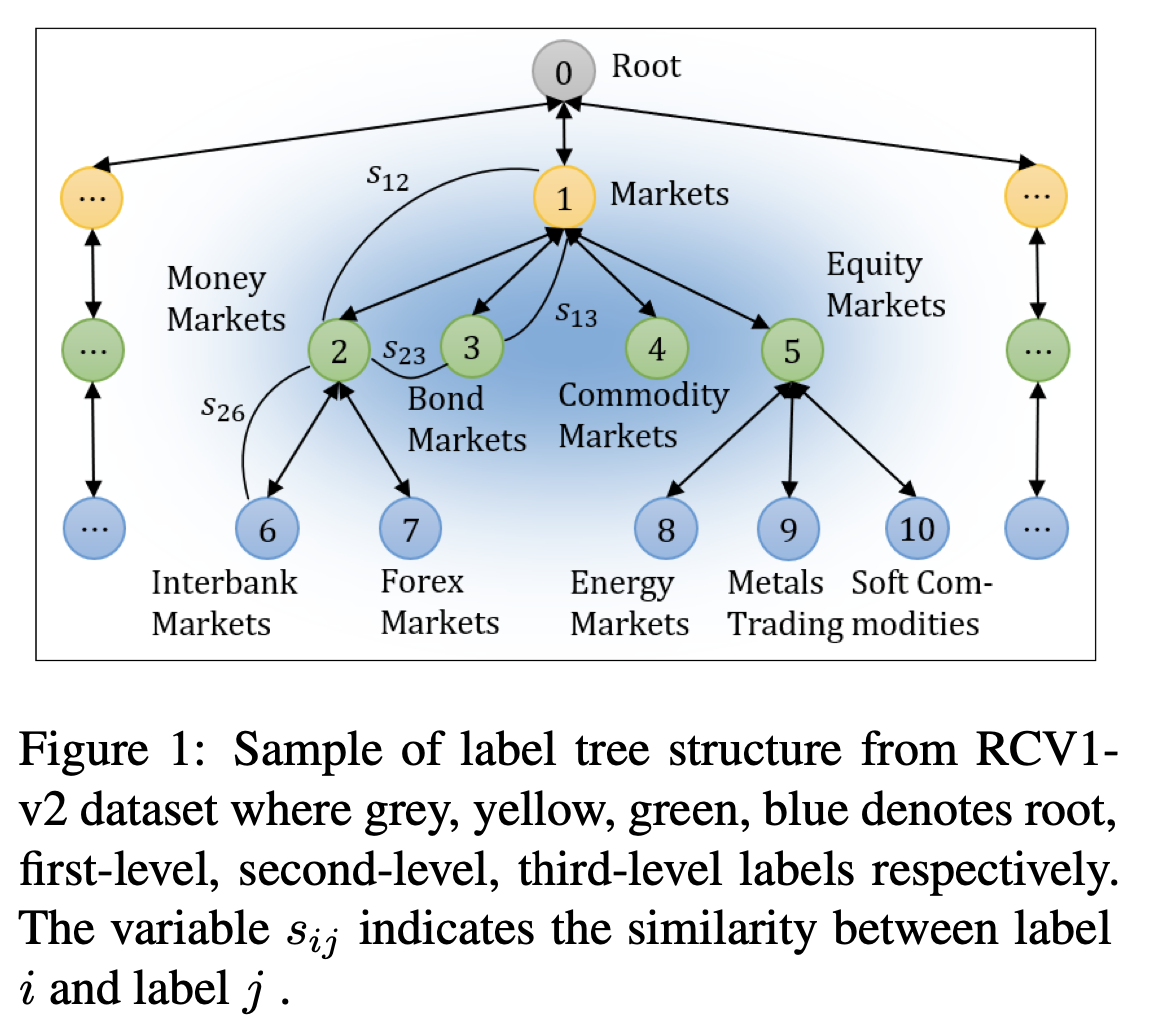## Method

Recursive Regularization for Large-scale Classification with Hierarchical and Graphical Dependencies, KDD 2013 [paper]

\begin{align}L_c &= -\sum_{i=1}^m[y_i\log(y_i')+(1-y_i)\log(1-y_i')] \\L_r &= \sum_{i\in\mathcal{V}}\sum_{j\in child(i)}\frac12\lVert w_i-w_j\rVert^2 \\L &= L_c + \lambda_1L_r + \lambda_2L_d\end{align}

## Experiment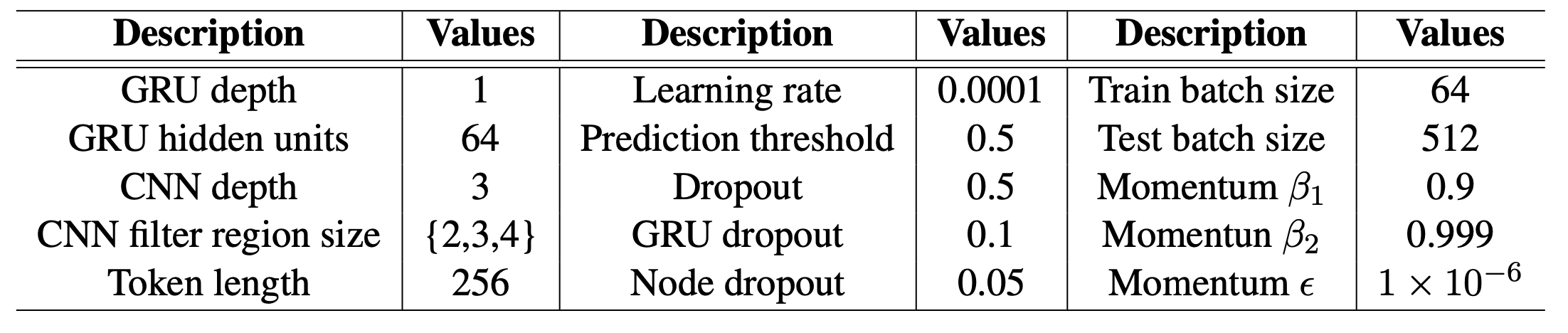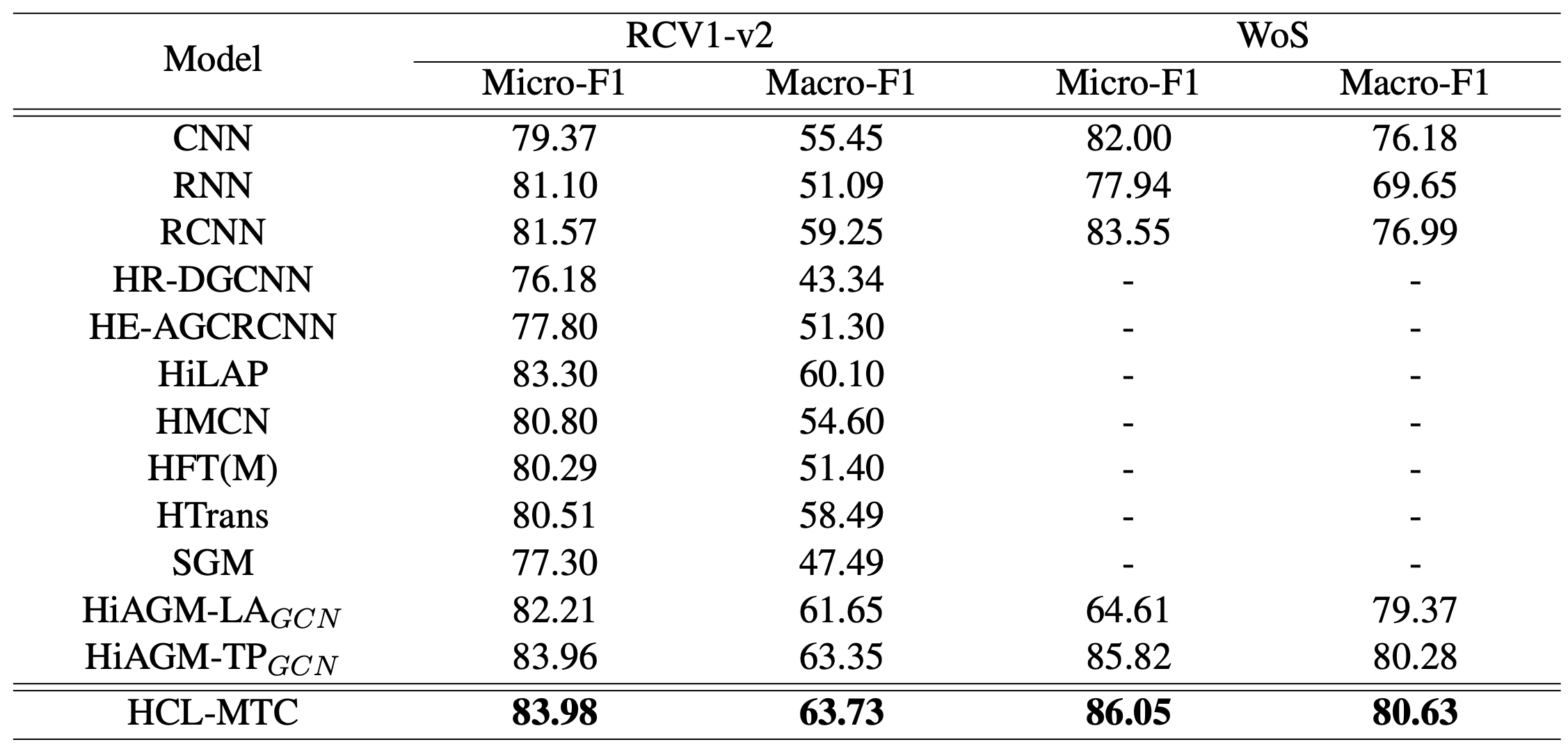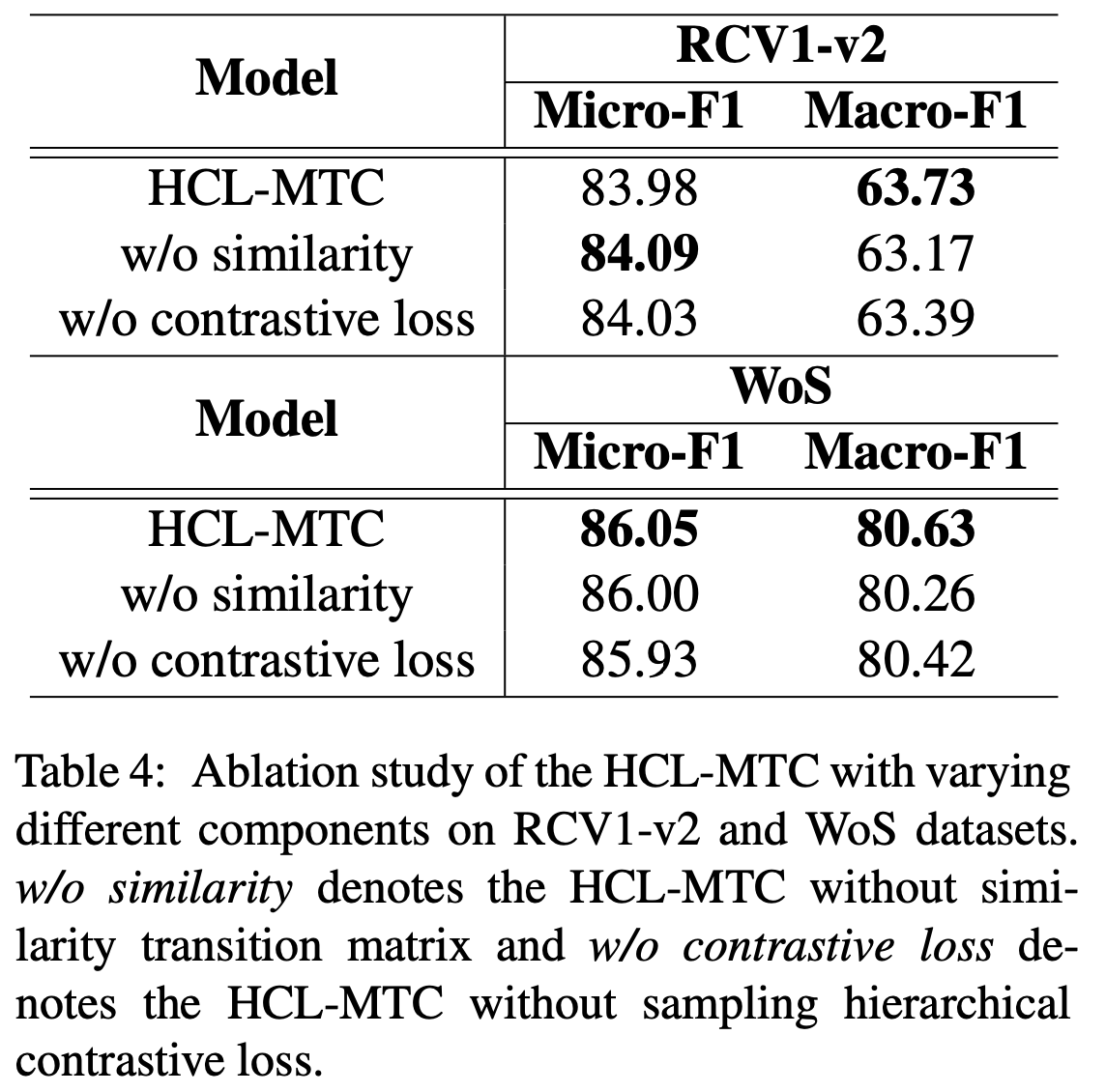]]>
<p>ACL ARR 2022，提出层次对比学习，学习标签间的区别信息。</p> <p><img src="/2022/02/13/HCL-MTC-Hierarchical-Contrastive-Learning-for-Multi-label-Text-Classification/image-20220216130823448.png" alt="image-20220216130823448" style="zoom: 33%;"></p>
Modeling Diagnostic Label Correlation for Automatic ICD Coding https://entropy2333.github.io/2022/02/05/Modeling-Diagnostic-Label-Correlation-for-Automatic-ICD-Coding/ 2022-02-05T08:15:11.000Z 2022-02-06T06:30:53.801Z NAACL 2021，提出了一个two-stage框架以捕获标签相关性，提升自动ICD编码的性能。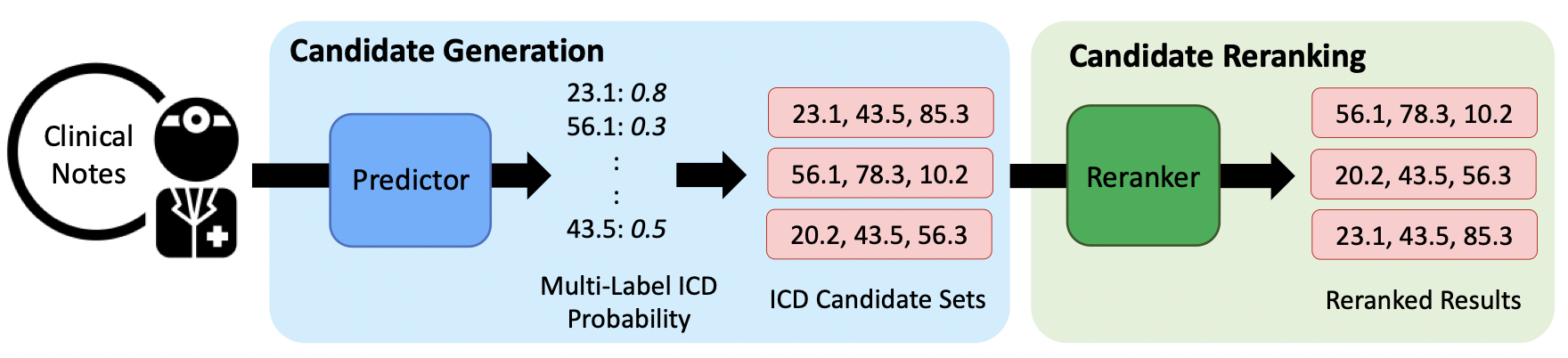## Overview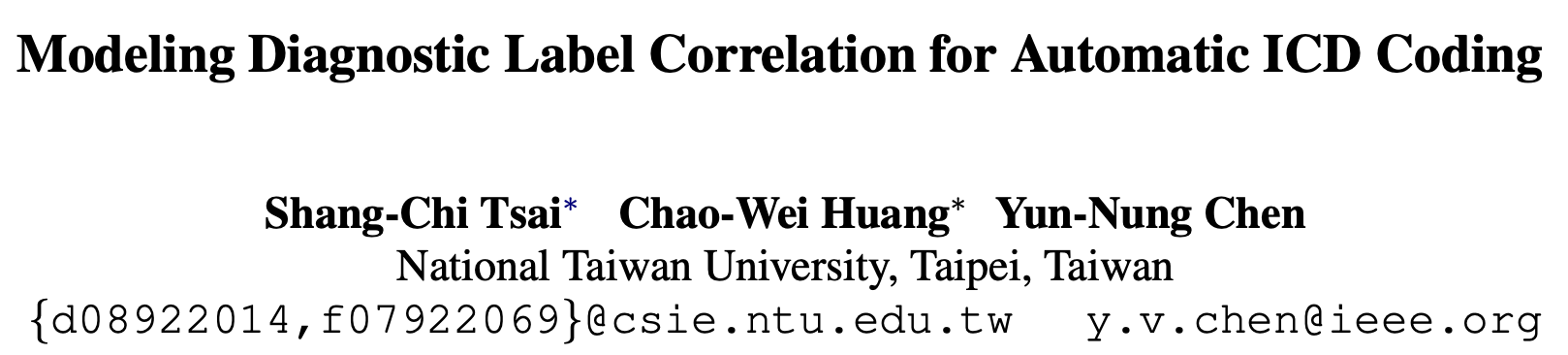## Method

1. 标签候选集生成，采用基本的分类器得到标签概率。
2. 标签候选集重排，利用标签相关性重排候选标签。

### Candidate Generation

[ICML 2016] Conditional bernoulli mixtures for multi-label classification [paper]

def get_n_best(probs, n=10):    flip_idx = np.argsort(np.abs(probs-0.5))[:n]    labels = np.zeros(len(probs))    cum_prob = 0.0    for i, prob in enumerate(probs):        if i in flip_idx:            continue        if prob >= 0.5:            labels[i] = 1            cum_prob += np.log(prob)        else:            labels[i] = 0            cum_prob += np.log(1-prob)    last_queue = PriorityQueue()    last_queue.put((cum_prob, labels))    for i, prob in enumerate(probs):        if i not in flip_idx:            continue        queue = PriorityQueue()        for cum_prob, labels in last_queue.queue:            labels2 = np.copy(labels)            labels[i] = 1            queue.put((cum_prob + np.log(prob+1e-6), labels))            labels2[i] = 0            queue.put((cum_prob + np.log(1-prob+1e-6), labels2))        while len(queue.queue) > n:            _ = queue.get()        last_queue = queue    n_best = []    while not queue.empty():        n_best.append(queue.get())    n_best = n_best[::-1]    return n_best

### Candidate Reranking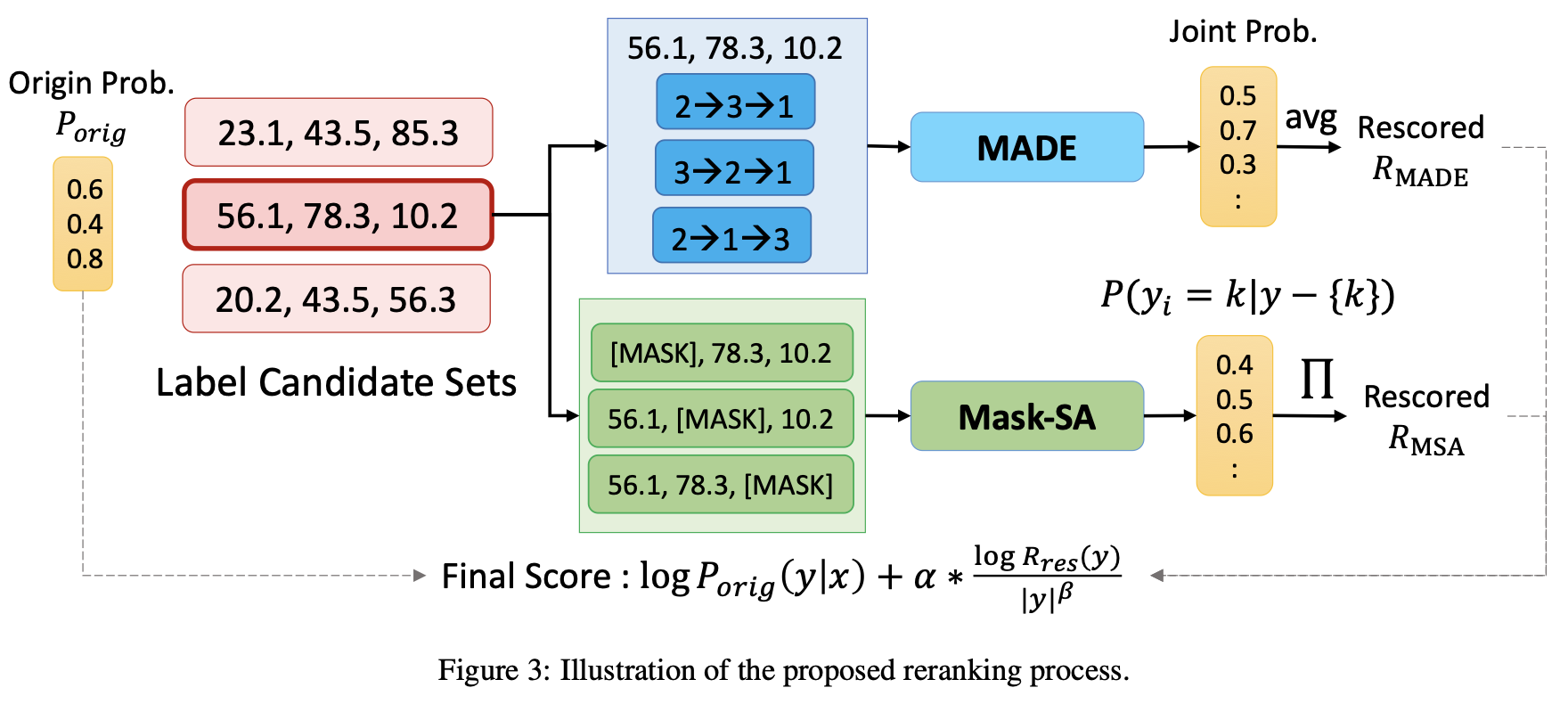MADE采用了一个掩码自编码器估计密度，通过自回归的方式估计联合概率$$P(\hat{\mathrm{y}})$$$P_{MADE}(\hat{\mathrm{y}})=\prod_{i=1}^{|\mathcal{Y}|}P_{MADE}(y_i=\hat{\mathrm{y}}_i|\hat{\mathrm{y}}_{o<i},\theta_{MADE})$ 其中$$o$$表示$$\{1,2,\cdots,|\mathcal{Y}|\}$$中的随机排列，$$o(i)$$表示新的排序，$$\hat{\mathrm{y}}_{o<i} = \{\hat{\mathrm{y}}_j|o(j)<o(i)\}$$表示新的排序中先于$$\hat{\mathrm{y}}_i$$的所有元素集合。

input_ids, attention_mask = build_masked_input(    token_ids, tokenizer, mask_positions=[i])all_input_ids.append(input_ids)all_attention_mask.append(attention_mask)all_input_ids = torch.tensor(all_input_ids).cuda()all_attention_mask = torch.tensor(all_attention_mask).cuda()with torch.no_grad():    output = model(all_input_ids, all_attention_mask)prediction_scores = outputlog_prob = 0.0for i, token_id in enumerate(token_ids):    log_prob += prediction_scores[i, i].detach().log_softmax(dim=-1)[token_id].cpu().item()nbests_with_bert.append((0.0, log_prob, len(token_ids), prob, labels))

## Experiment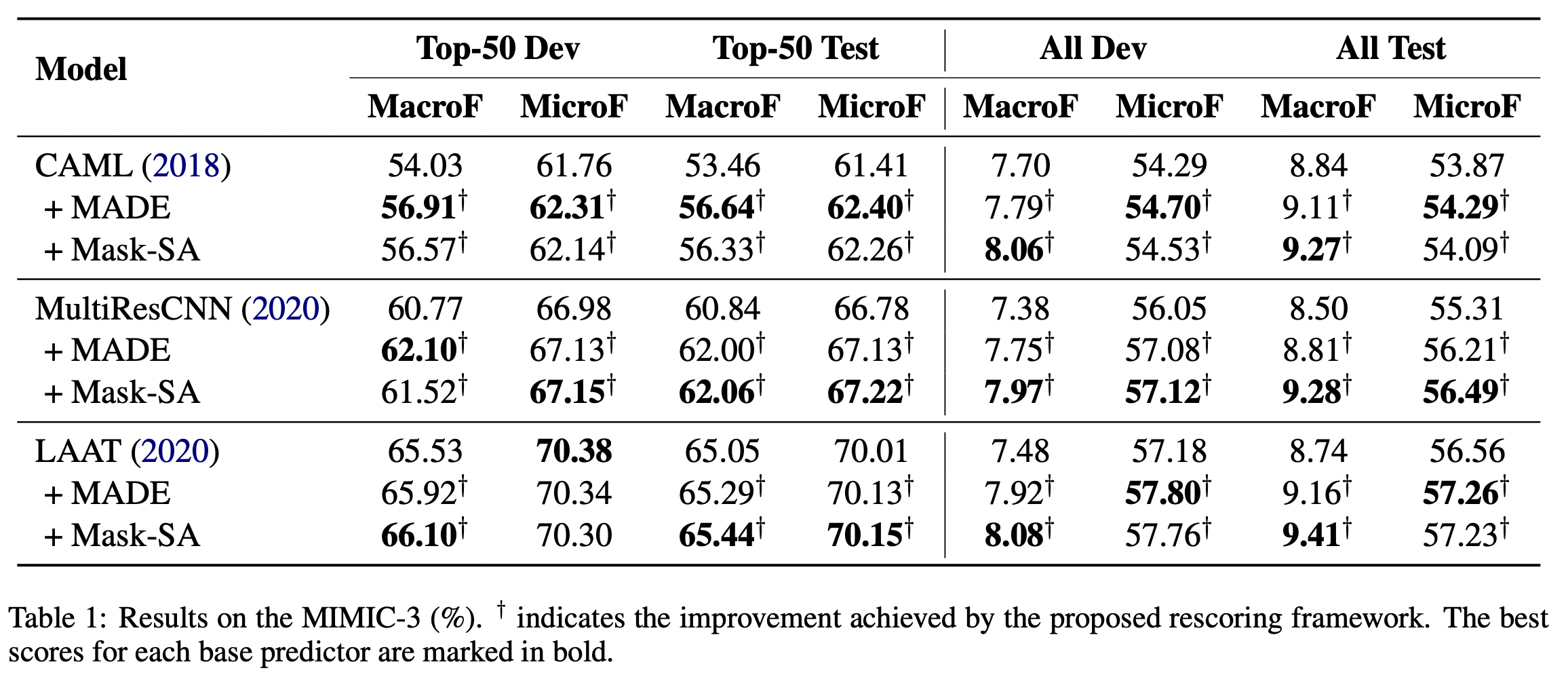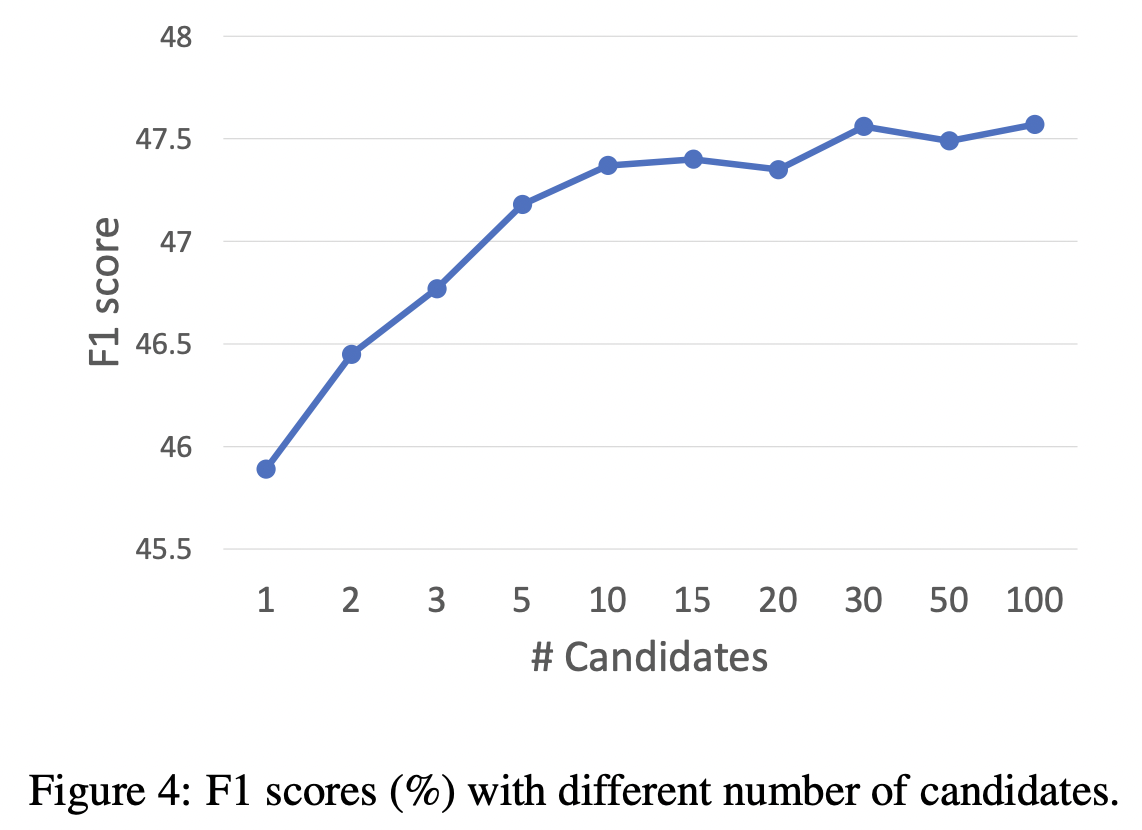]]>
<p>NAACL 2021，提出了一个two-stage框架以捕获标签相关性，提升自动ICD编码的性能。</p> <p><img src="/2022/02/05/Modeling-Diagnostic-Label-Correlation-for-Automatic-ICD-Coding/image-20220205161927097.png" alt="image-20220205161927097" style="zoom:50%;"></p>
Perceiver: General Perception with Iterative Attention https://entropy2333.github.io/2022/02/02/Perceiver-General-Perception-with-Iterative-Attention/ 2022-02-02T07:29:03.000Z 2022-02-02T08:29:05.819Z ICML 2021，来自DeepMind，目前大多数模型只能处理单模态，本文提出基于Transformer的Perceiver模型，适用于各种各样的输入，不需要过多的特定假设。模型利用非对称的注意力机制，将输入迭代地提取到一个很小的隐藏bottleneck，从而可以处理非常大的输入。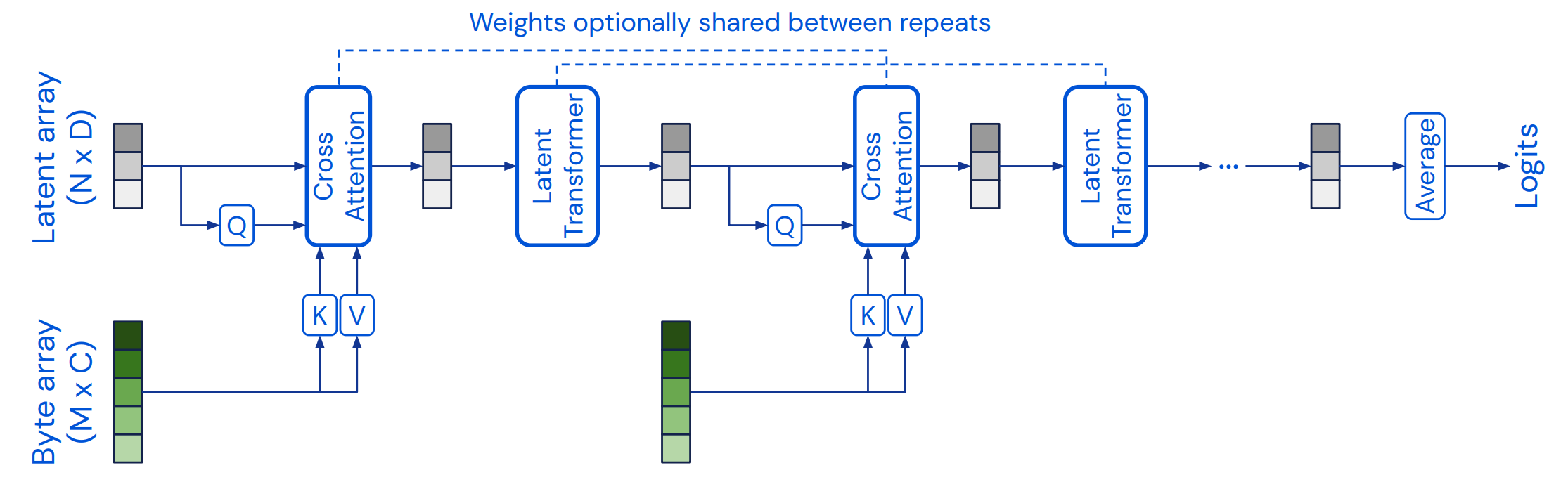## Overview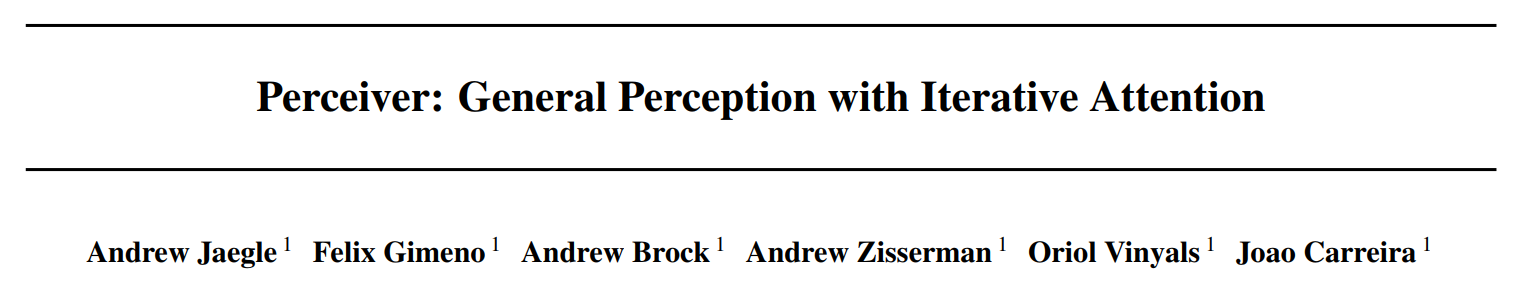## Method

### The Perceiver architecture

• 一个cross-attention模块，将一个字节序列和潜在序列映射到一个潜在序列。
• 一个Transformer tower，将一个潜在序列映射到一个潜在序列。

cross-attention将注意力的复杂度由$$\mathcal{O}(M^2)$$降低到了$$\mathcal{O}(MN)$$，其中$$M$$$$N$$分别表示$$Q$$$$K$$的长度，一般情况下$$N\ll M$$。从而在后续的Transformer中，计算代价由$$\mathcal{O}(LM^2)$$降低到了$$\mathcal{O}(LN^2)$$

Transformer使用了GPT-2架构，潜在序列使用可学习的位置编码进行初始化。

## Experiment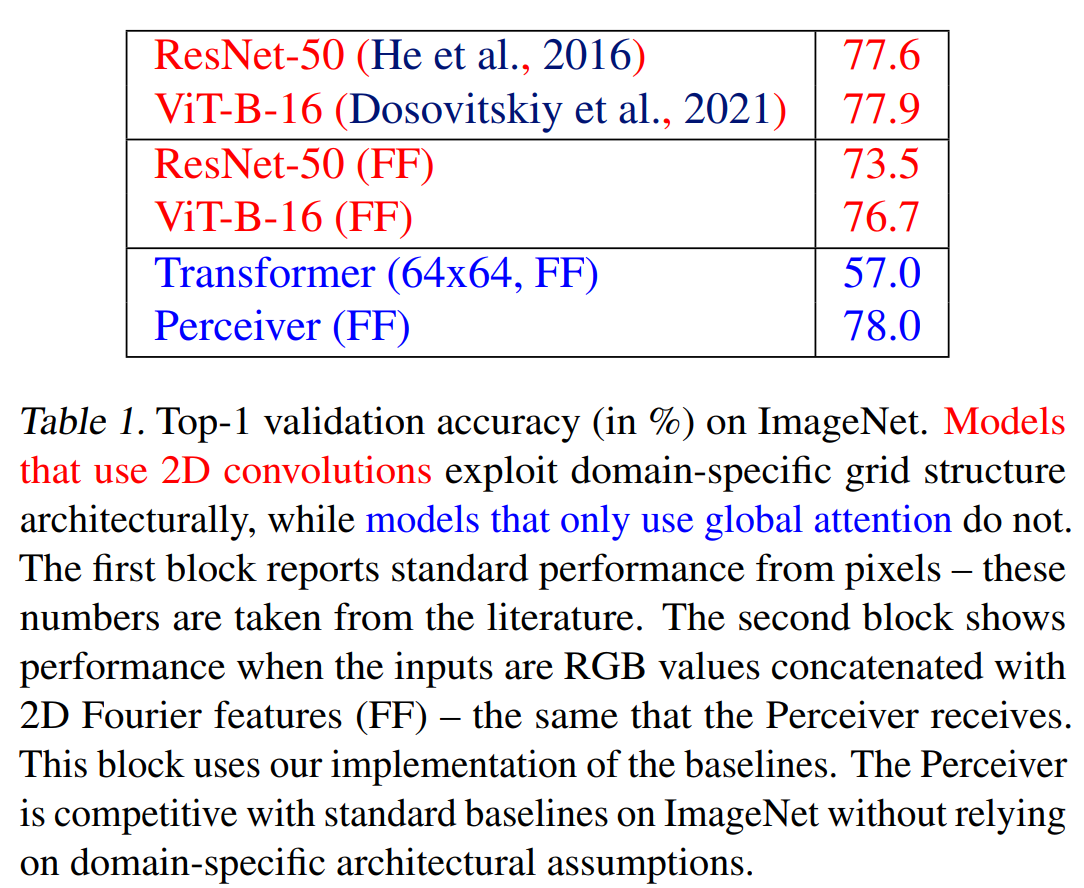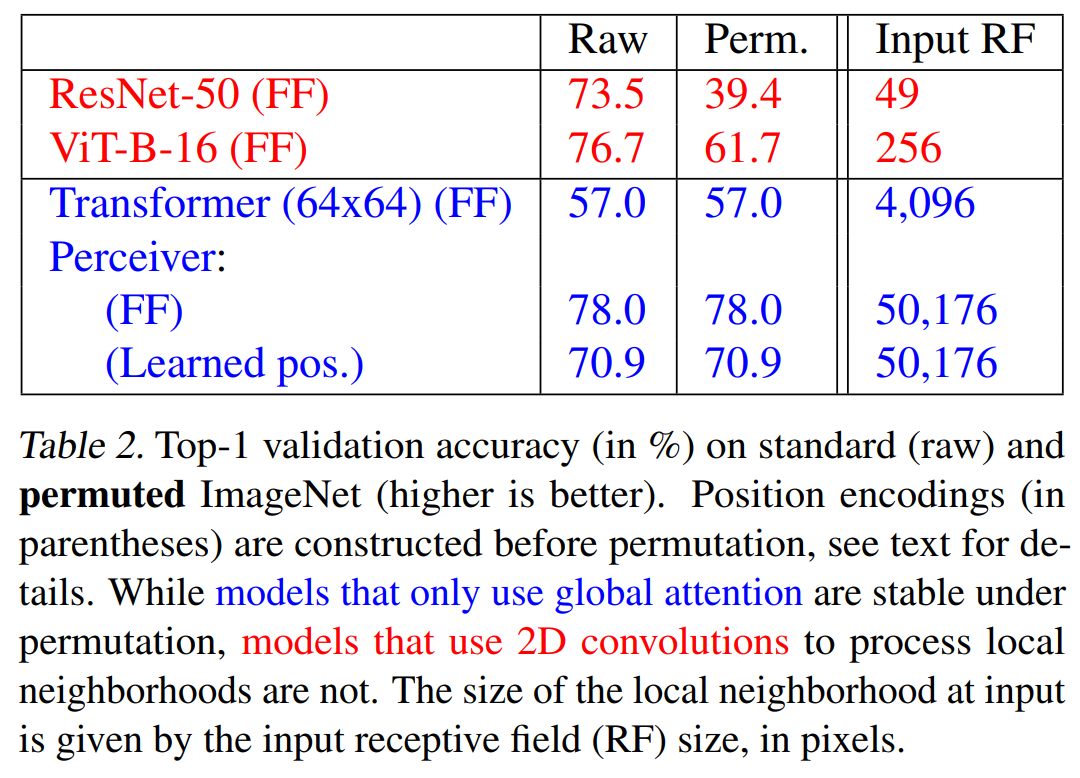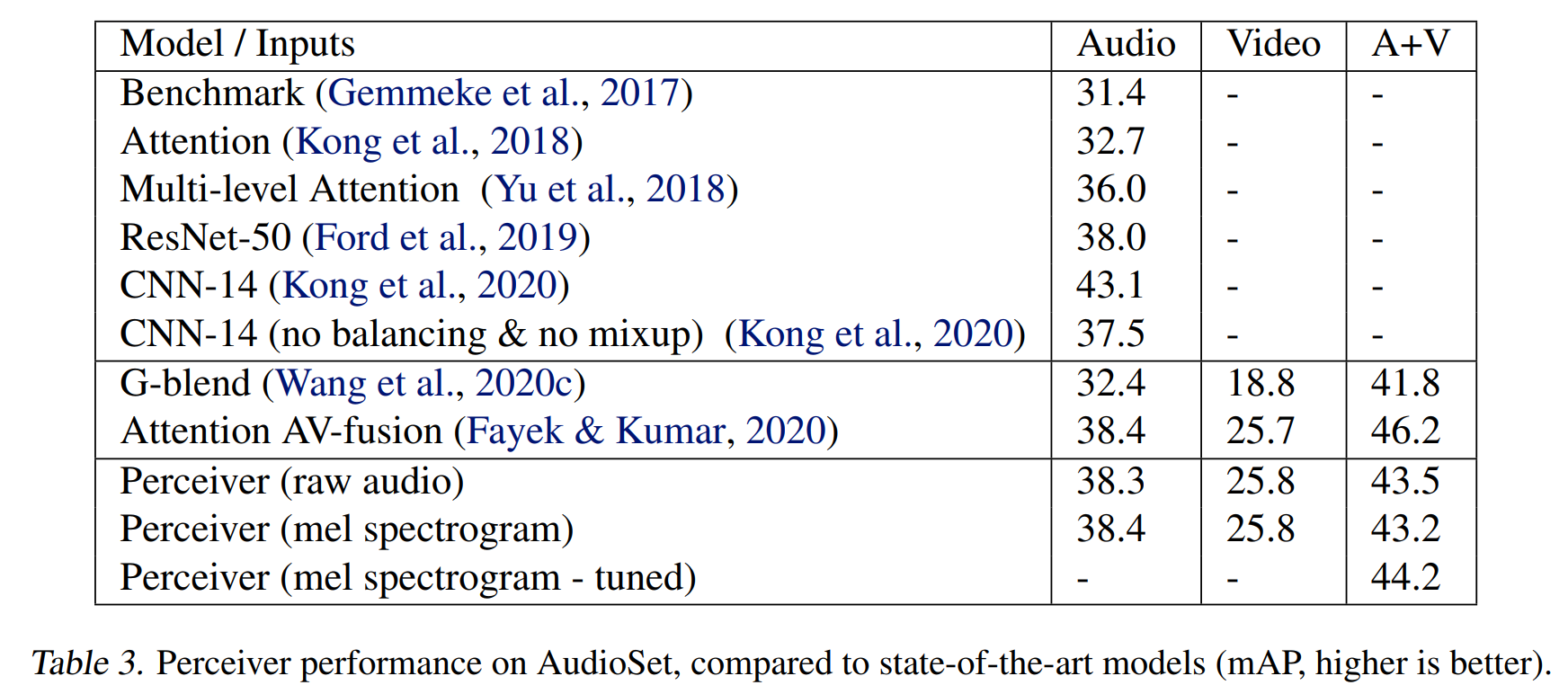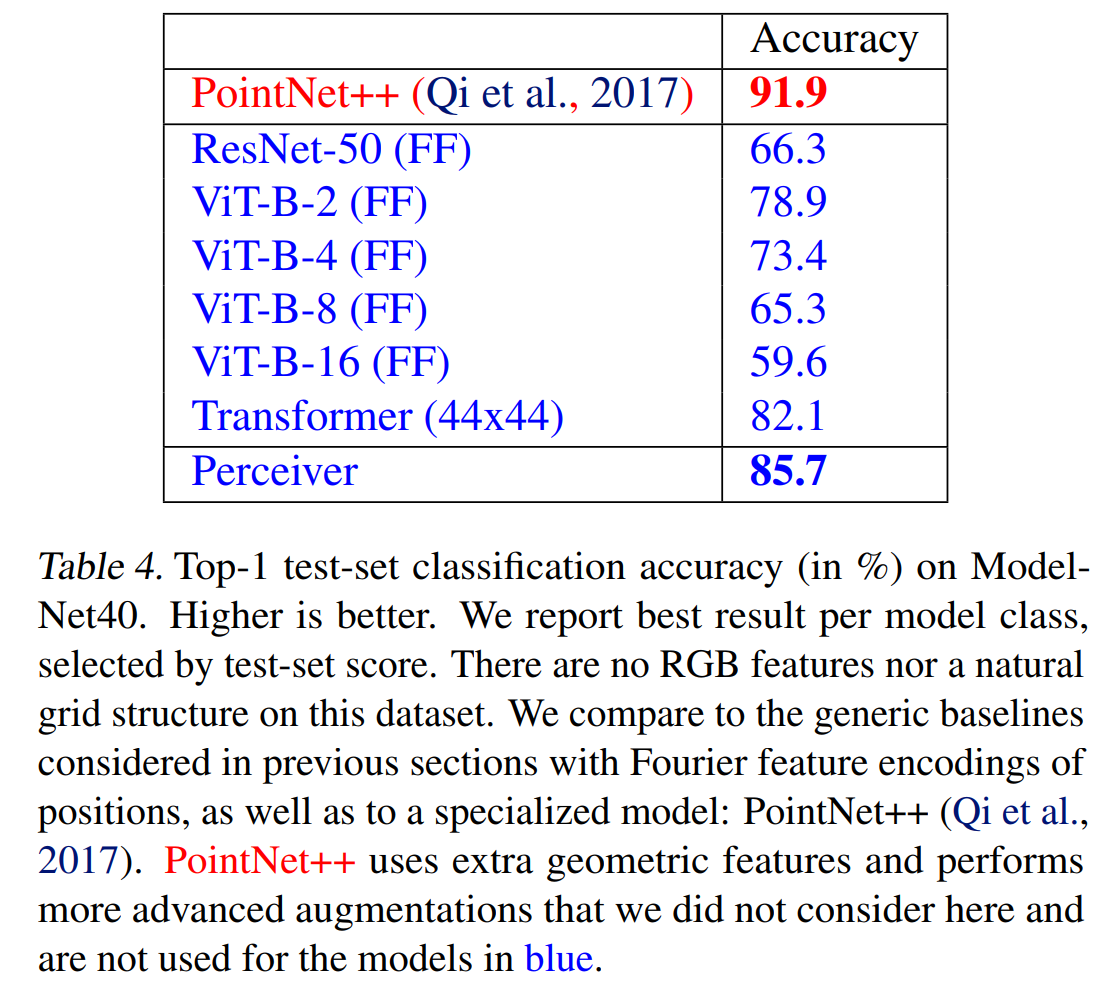]]>
<p>ICML 2021，来自DeepMind，目前大多数模型只能处理单模态，本文提出基于Transformer的Perceiver模型，适用于各种各样的输入，不需要过多的特定假设。模型利用非对称的注意力机制，将输入迭代地提取到一个很小的隐藏bottleneck，从而可以处理非常大的输入。</p> <p><img src="/2022/02/02/Perceiver-General-Perception-with-Iterative-Attention/image-20220202153941841.png" alt="image-20220202153941841" style="zoom: 50%;"></p>
Perceiver IO: A General Architecture for Structured Inputs & Outputs https://entropy2333.github.io/2022/02/02/Perceiver-IO-A-General-Architecture-for-Structured-Inputs-Outputs/ 2022-02-02T07:20:20.000Z 2022-02-02T09:11:01.985Z 来自DeepMind，Perceiver的续作，不再局限于分类任务，在NLP、CV、多模态甚至星际争霸二上取得了不错的成绩。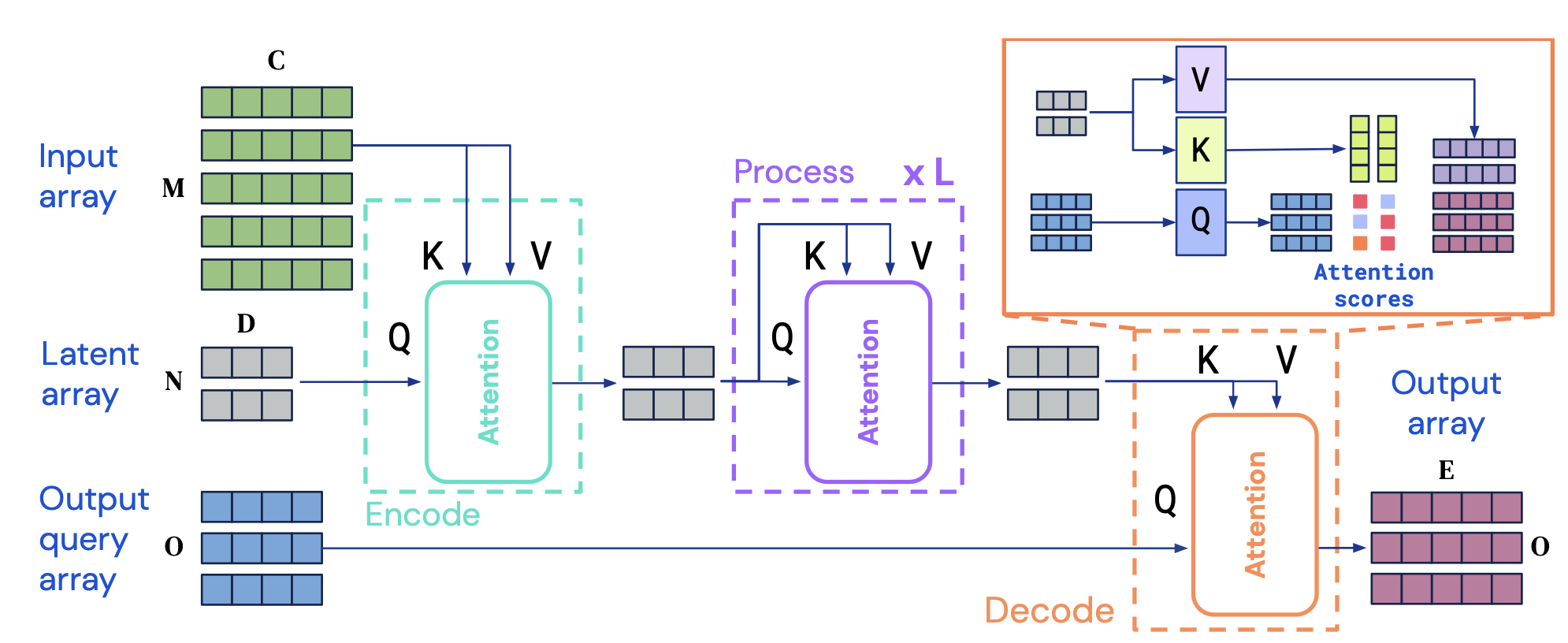## Overview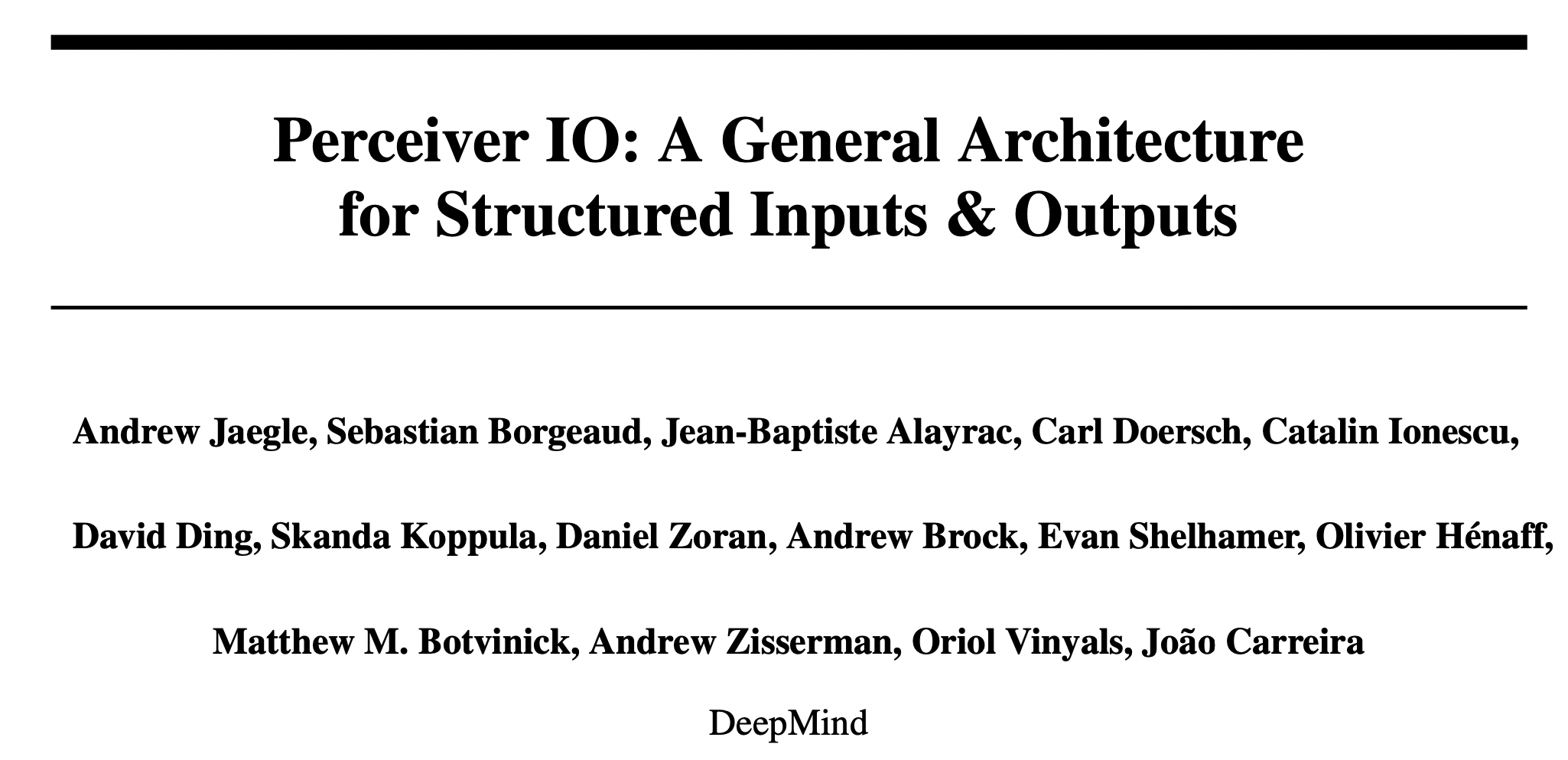## Background

Perceiver只能处理分类任务，本文提出了一种用于解码结构化输出的机制，使得模型能够处理大量新的任务而不需要领域特有的处理方法。

Perceiver IO是一个纯注意力的架构，输入编码到潜在空间，潜在的表示通过多层处理，经过解码得到最终的输出。

## Method

### Decoding with a query array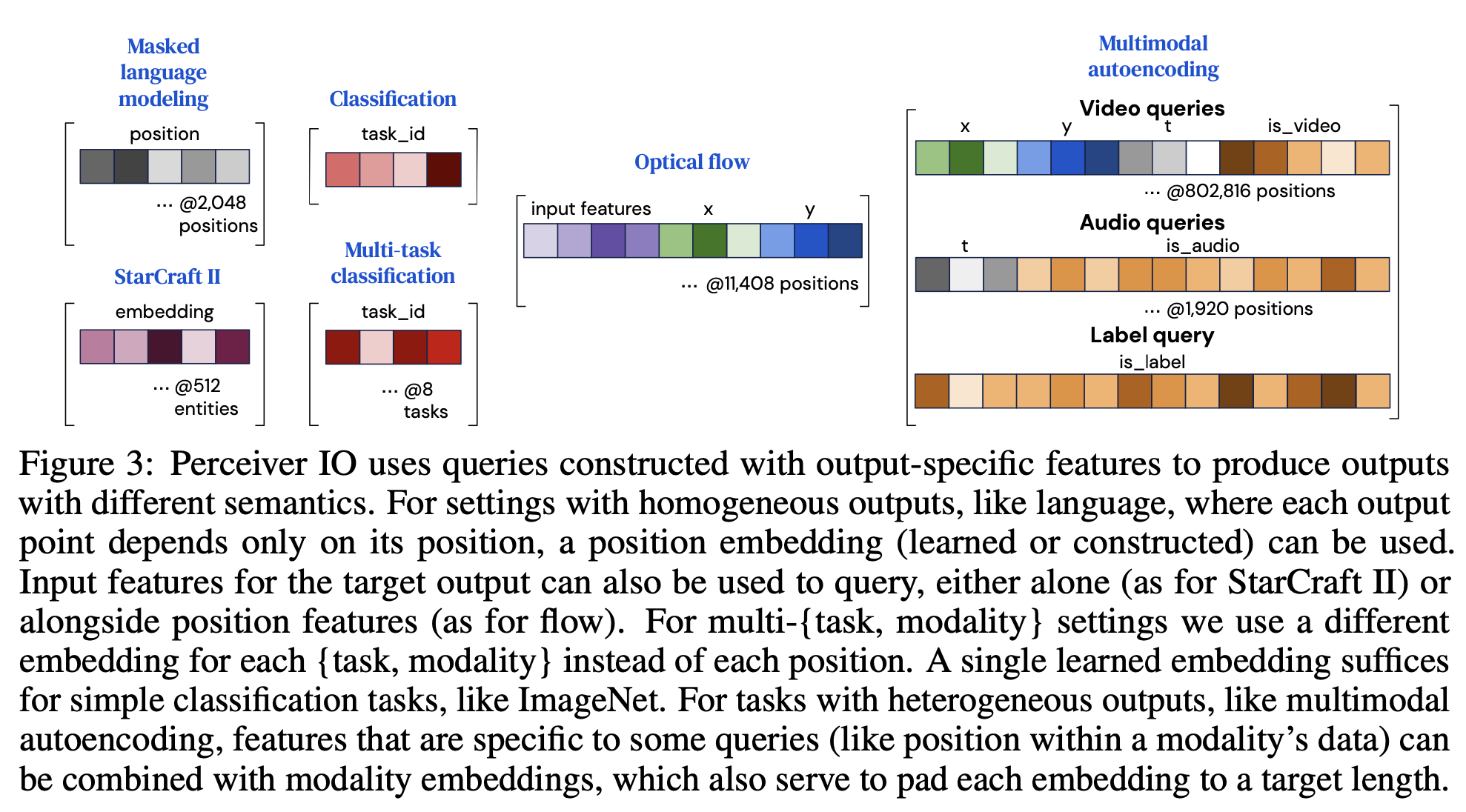## Experiment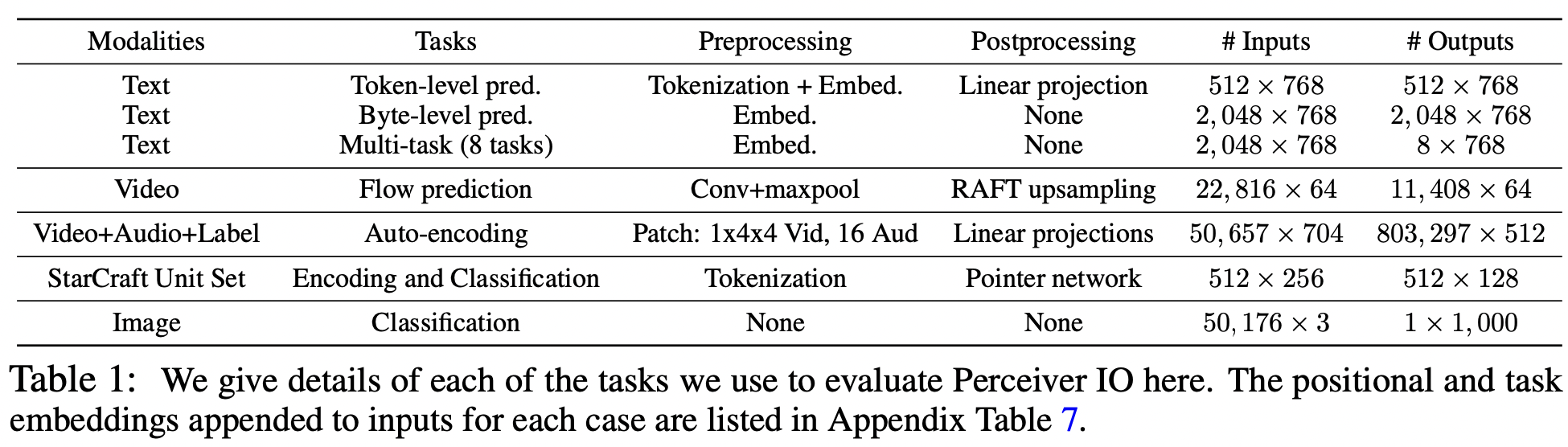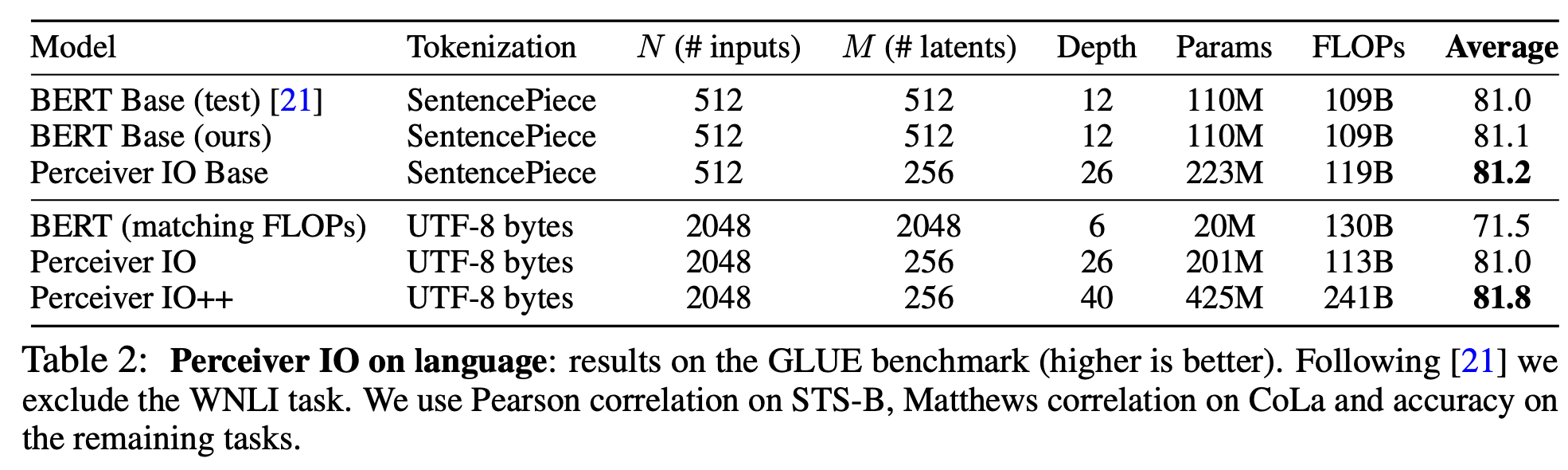## Conclusion

]]>
<p>来自DeepMind，Perceiver的续作，不再局限于分类任务，在NLP、CV、多模态甚至星际争霸二上取得了不错的成绩。</p> <p><img src="/2022/02/02/Perceiver-IO-A-General-Architecture-for-Structured-Inputs-Outputs/image-20220202164605712.png" alt="image-20220202164605712" style="zoom:50%;"></p>
A novel reasoning mechanism for multi-label text classification https://entropy2333.github.io/2022/01/27/A-novel-reasoning-mechanism-for-multi-label-text-classification/ 2022-01-27T04:37:03.000Z 2022-01-28T04:53:18.481Z Information Processing and Management 2021（CCF-B）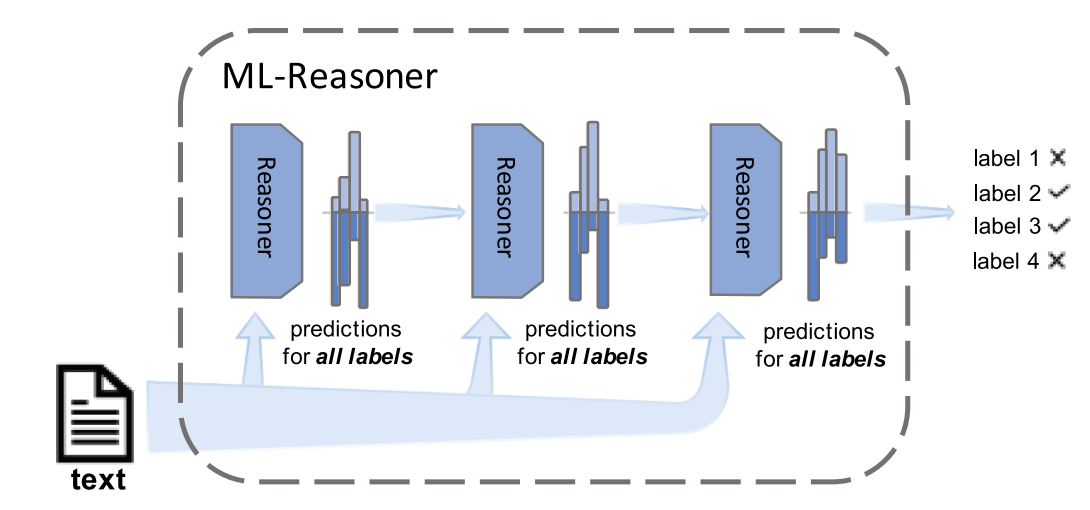## Overview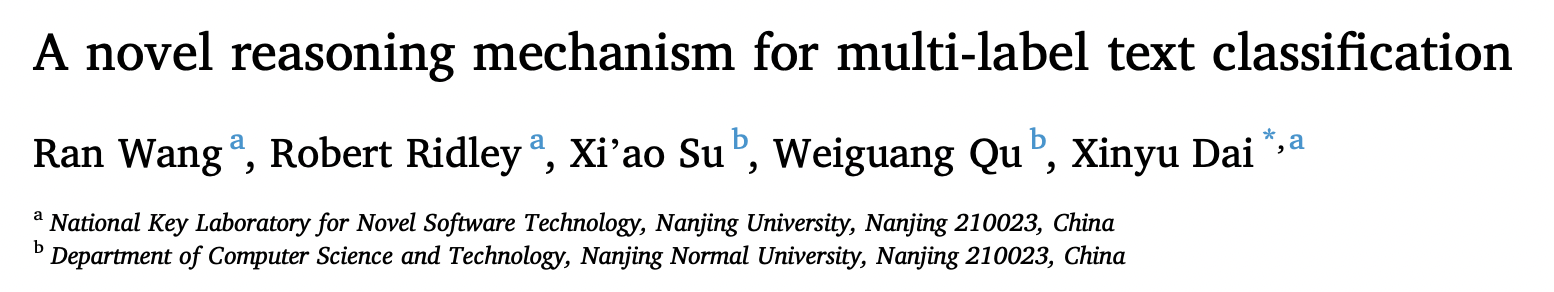## Method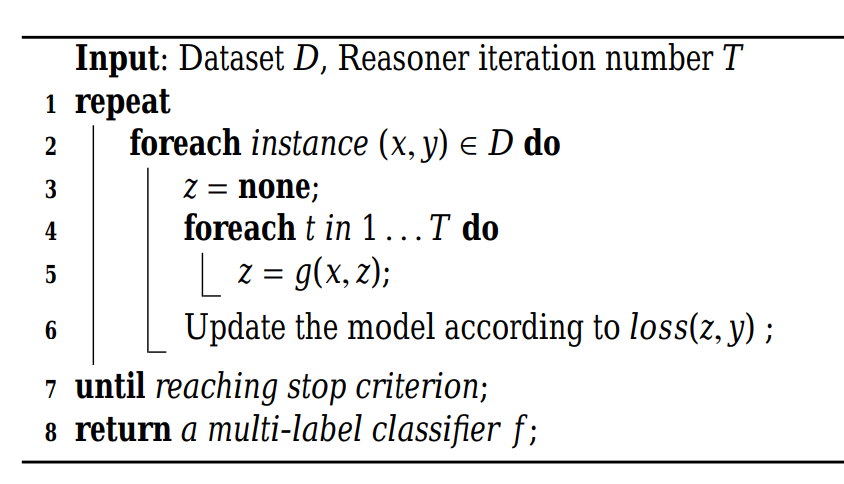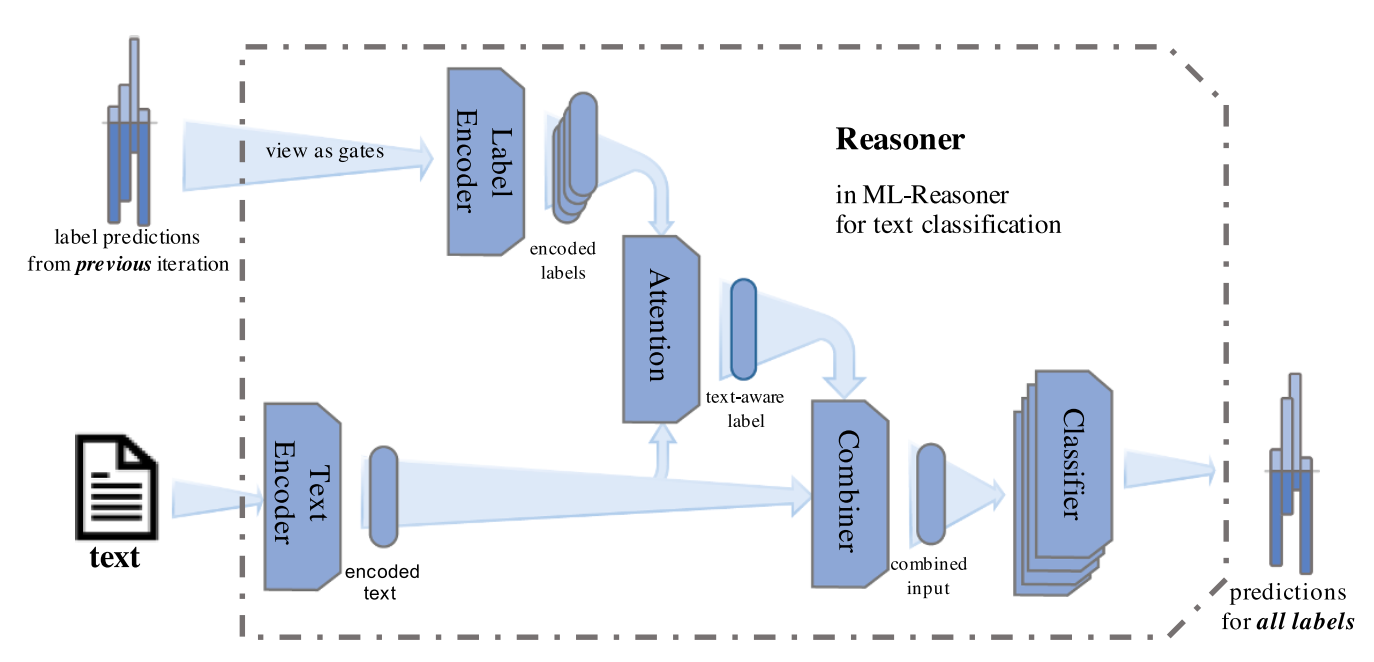Reasoner模块有点像Transformer的Decoder部分，分别对text和label进行embedding，并计算attention后分类。

## Experiment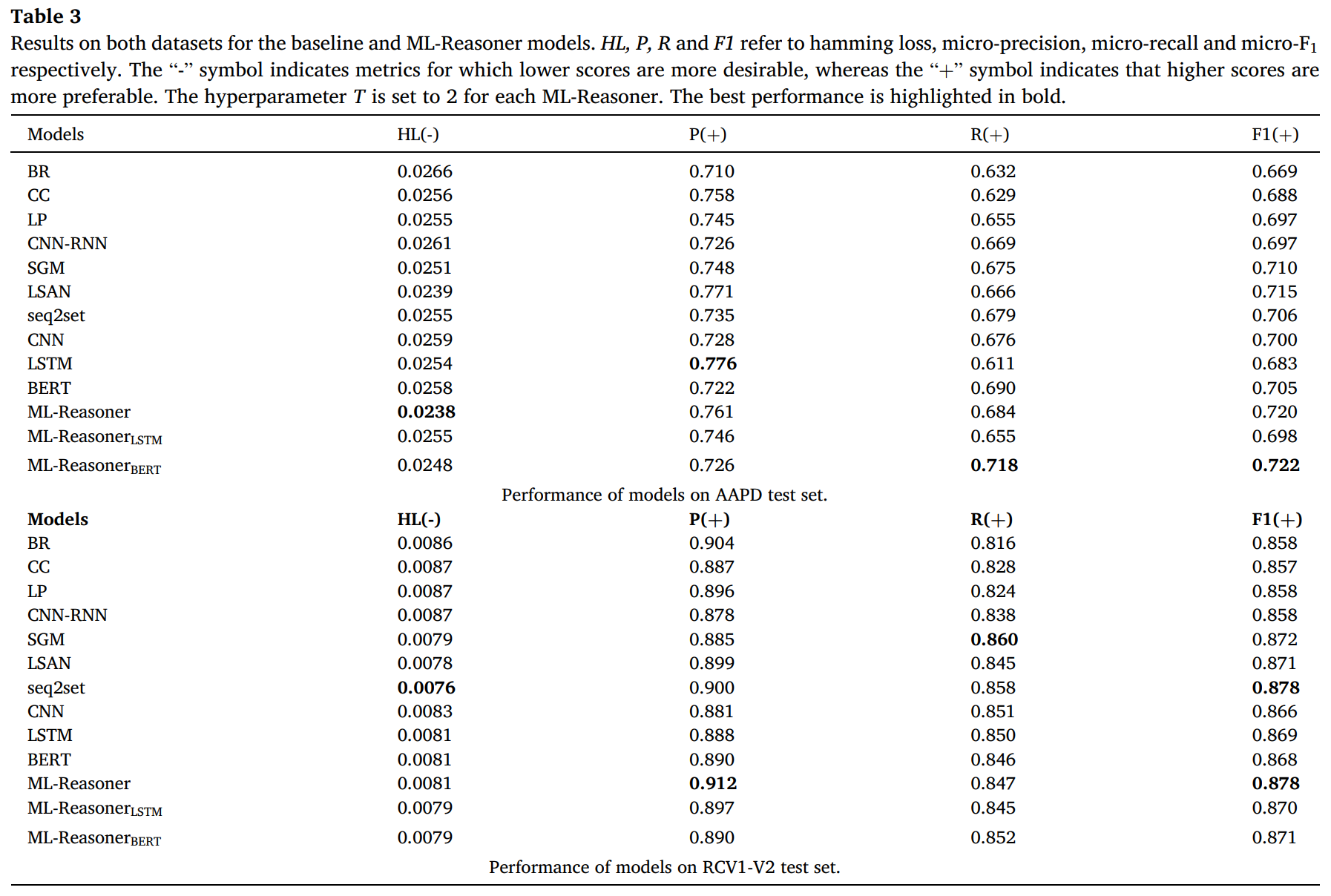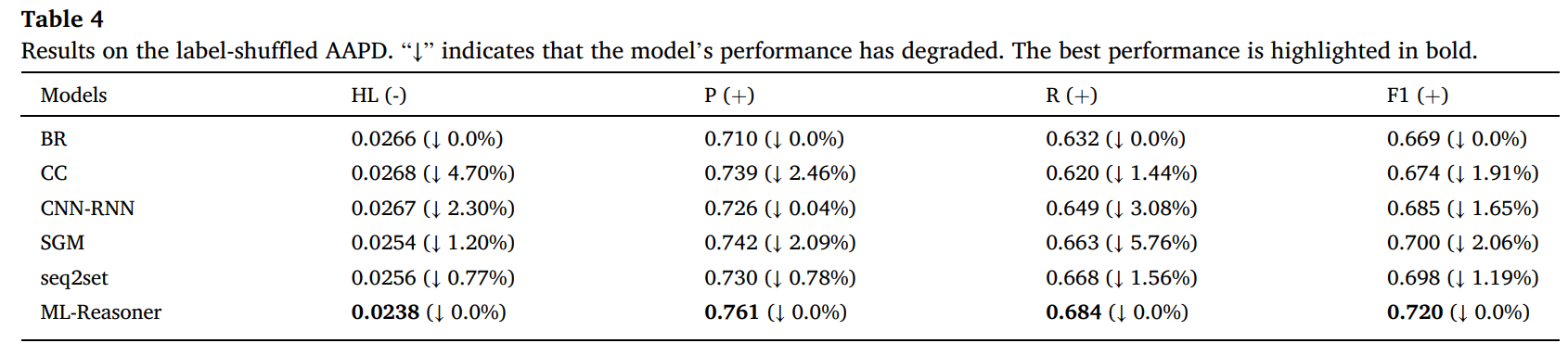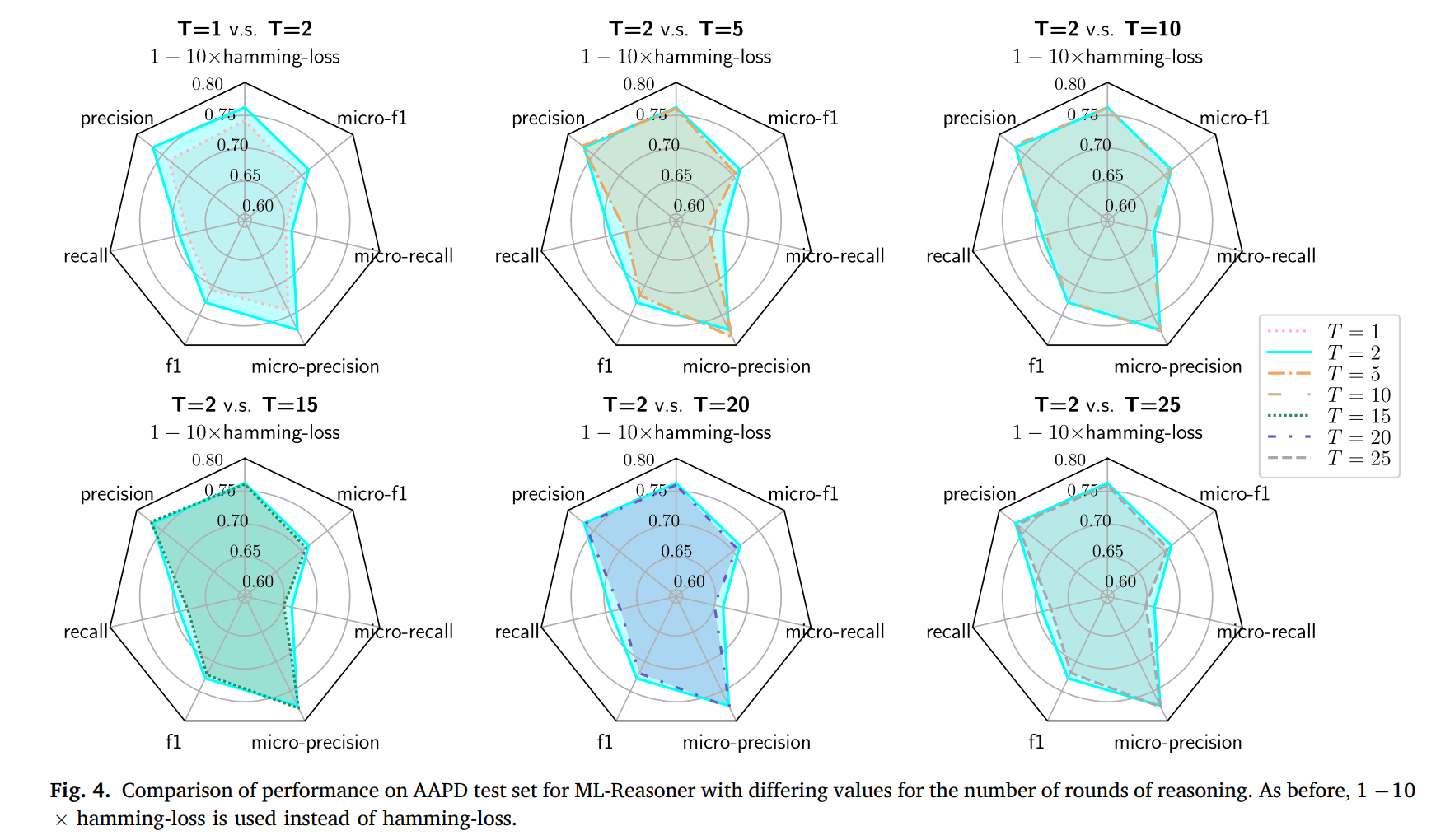]]>
<p>Information Processing and Management 2021（CCF-B）</p> <p><img src="/2022/01/27/A-novel-reasoning-mechanism-for-multi-label-text-classification/image-20220127133117985.png" alt="image-20220127133117985" style="zoom:50%;"></p>
Balancing Methods for Multi-label Text Classification with Long-Tailed Class Distribution https://entropy2333.github.io/2022/01/25/Balancing-Methods-for-Multi-label-Text-Classification-with-Long-Tailed-Class-Distribution/ 2022-01-25T06:06:28.000Z 2022-01-25T06:53:15.546Z EMNLP 2021，损失函数大杂烩。

## Overview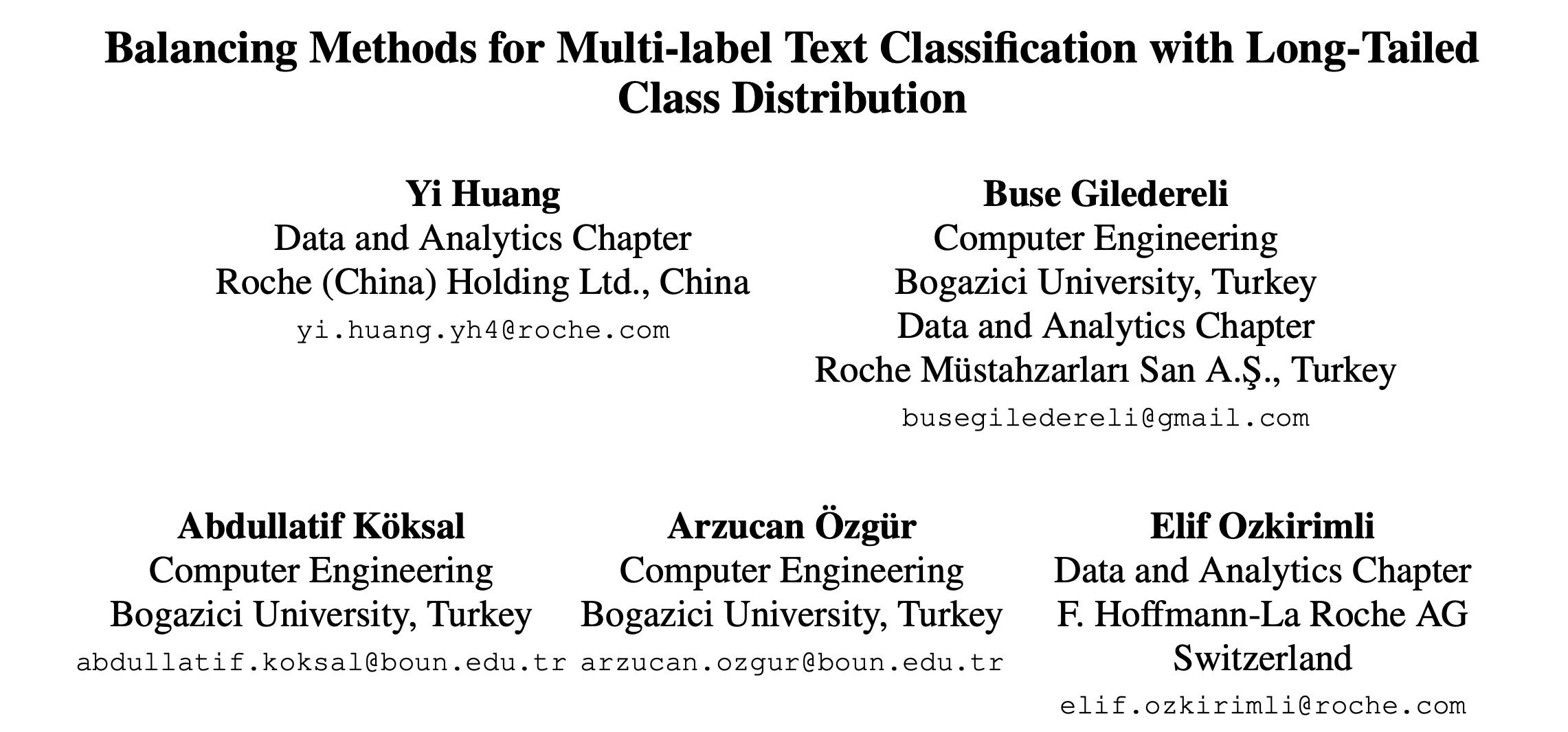## Method

### Binary Cross Entropy (BCE)

BCE是最基础的损失函数，其中$$p_i^k = \sigma(z_i^k)$$$L_{BCE} = \begin{cases}-\log(p_i^k) & \text{if}\ y_i^k = 1 \\-\log(1-p_i^k) & \text{otherwise}\end{cases}$

### Focal Loss (FL)

Focal Loss由恺明大神提出，在更难分类的样本上增加了损失权重。 $L_{FL} = \begin{cases}-(1-p_i^k)^\gamma\log(p_i^k) & \text{if}\ y_i^k = 1 \\-(p_i^k)^\gamma\log(1-p_i^k) & \text{otherwise}\end{cases}$

## Experiment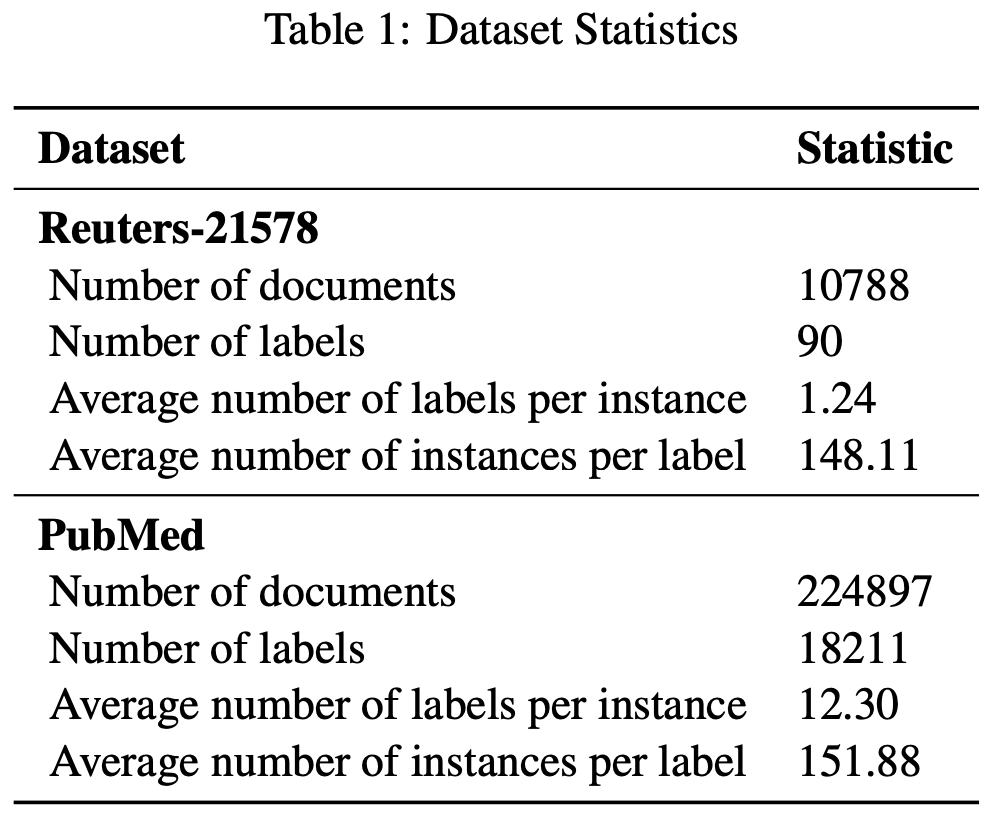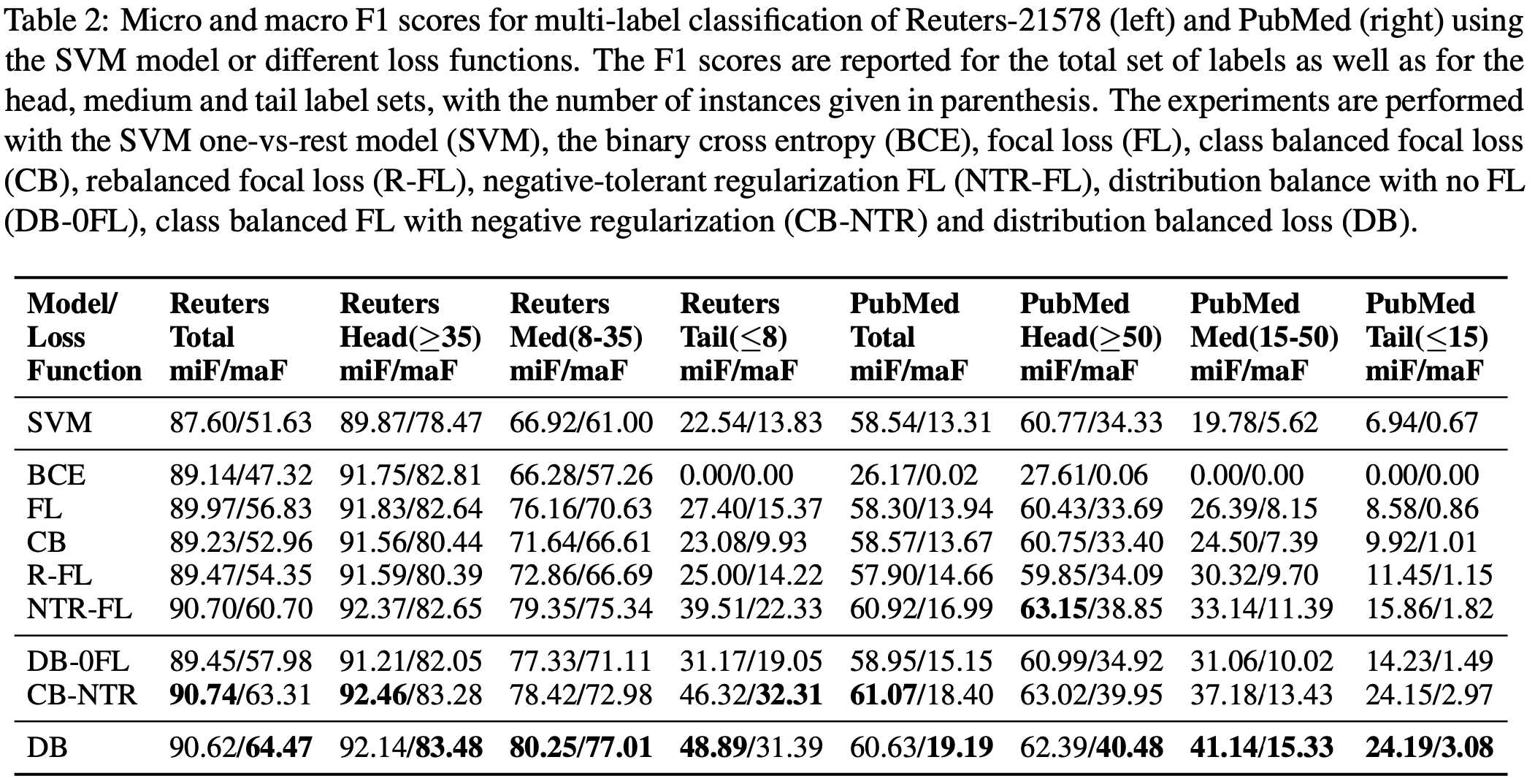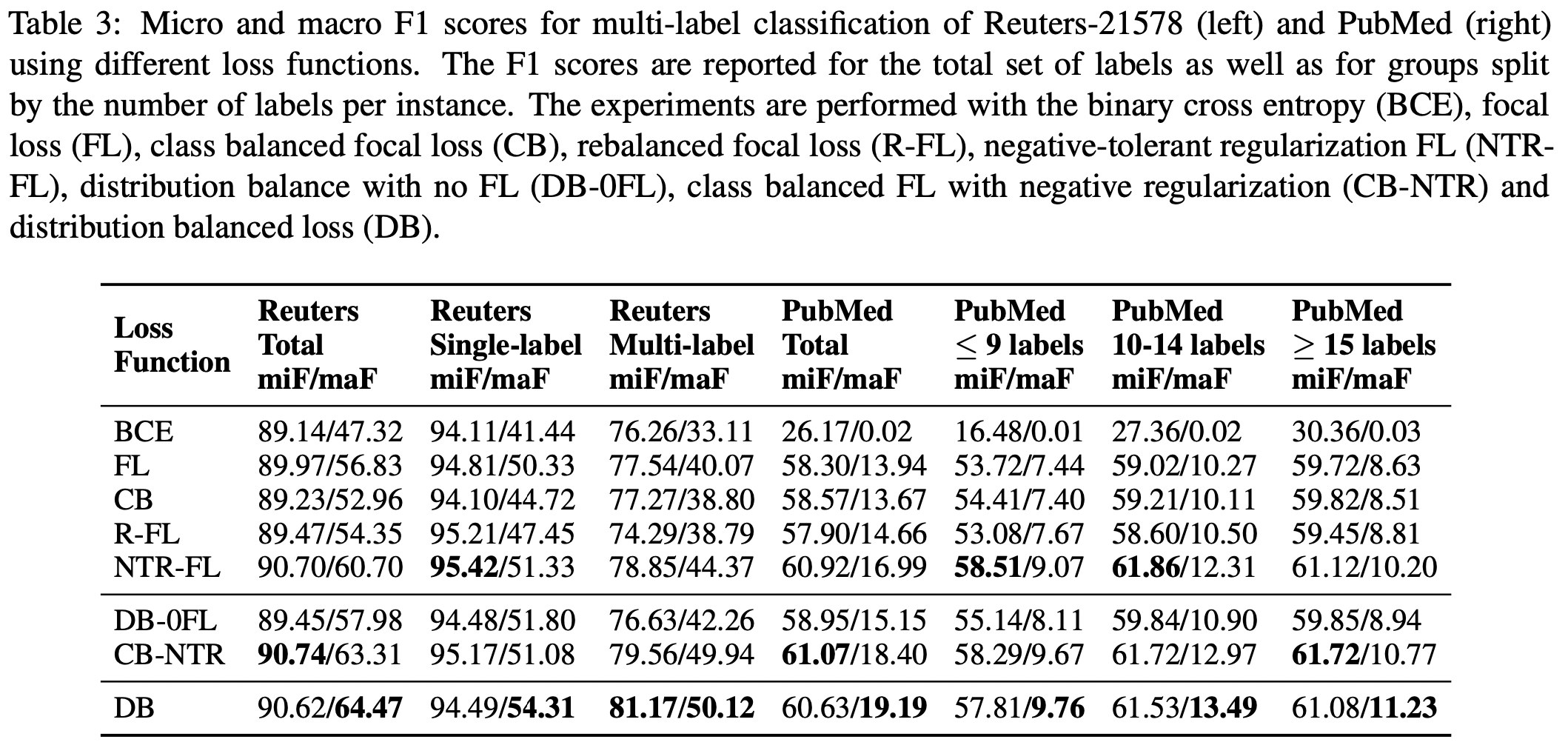]]>
<p>EMNLP 2021，损失函数大杂烩。</p>